# RD Sharma Solutions for Class 8 Maths Chapter 4 - Cubes and Cube Roots

Chapter 4 – Cubes and Cube Roots of RD Sharma Solutions for Class 8 Maths are provided here. RD Sharma Solutions help students secure good score in their examination, as they provide extensive knowledge about the subject, Class 8 being a critical stage in their academic career. BYJU’S expert team has solved the questions from the RD Sharma Class 8 textbook in a step by step format in detail, which helps students strengthen their concepts.

Chapter 4- Cubes and Cube Roots contain five exercises and the RD Sharma Class 8 Solutions present in this page provides solutions for the questions present in each exercise. Now, let us have a look at the concepts discussed in this chapter.

• Cube of a number – A natural number is said to be a perfect cube if it is a cube of some natural number.
• Finding a cube of a two-digit number by column method.
• Cubes of Negative Integers.
• Cubes of Rational Numbers.
• Cube root of a Natural Number.
• Cube root of a negative integral perfect cube.
• Cube root of the product of integers.
• Finding cube roots using cube root tables.

## Download the pdf of RD Sharma Solutions For Class 8 Maths Chapter 4 Cubes and Cube Roots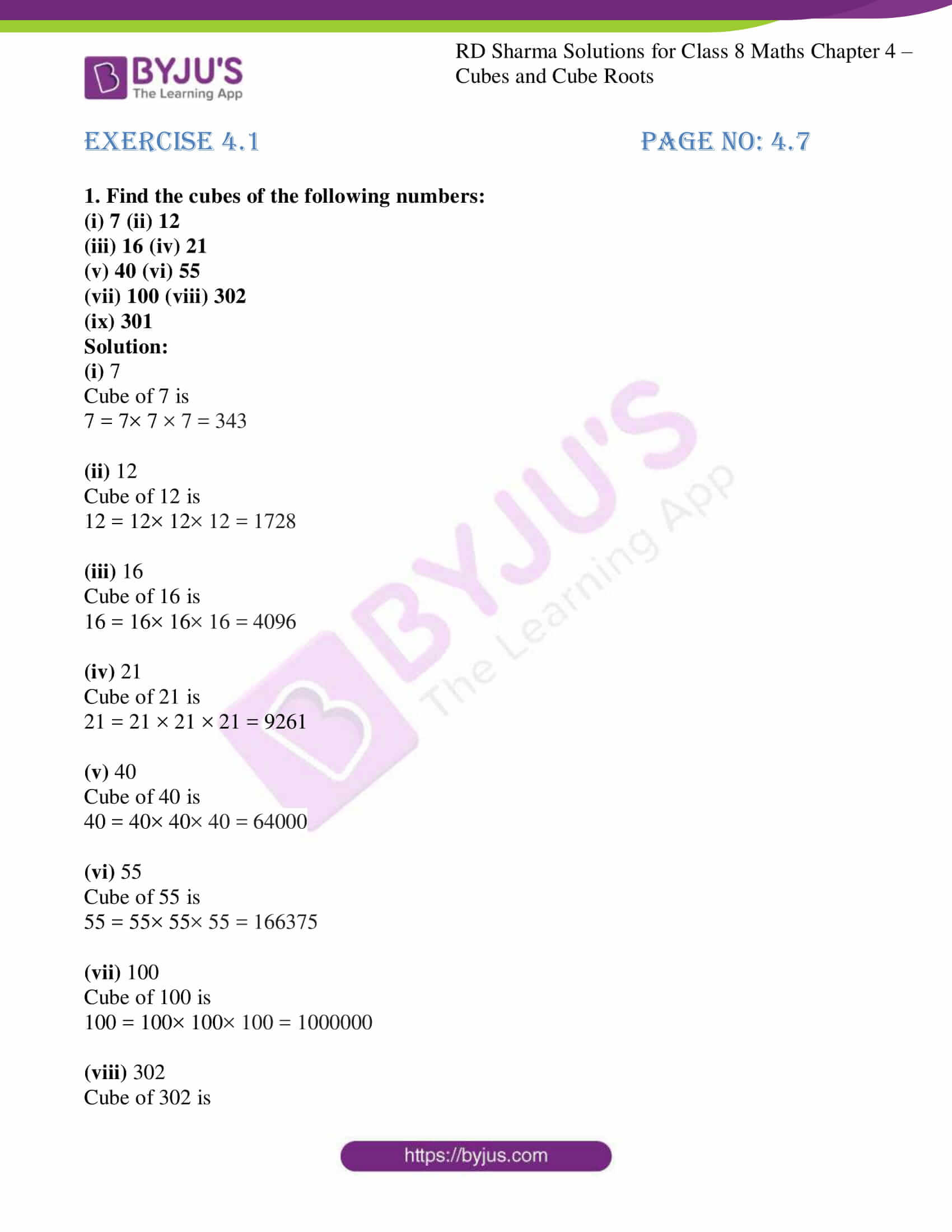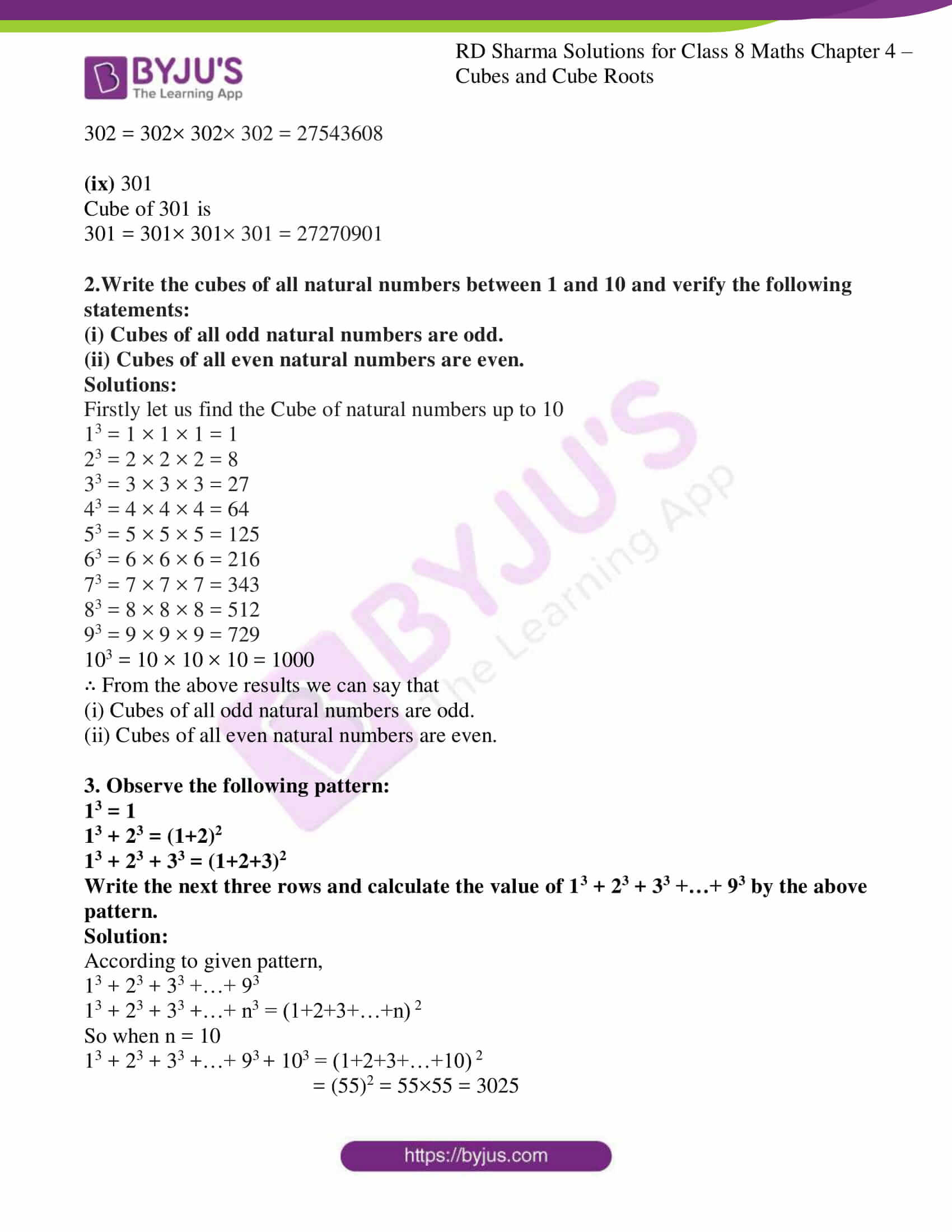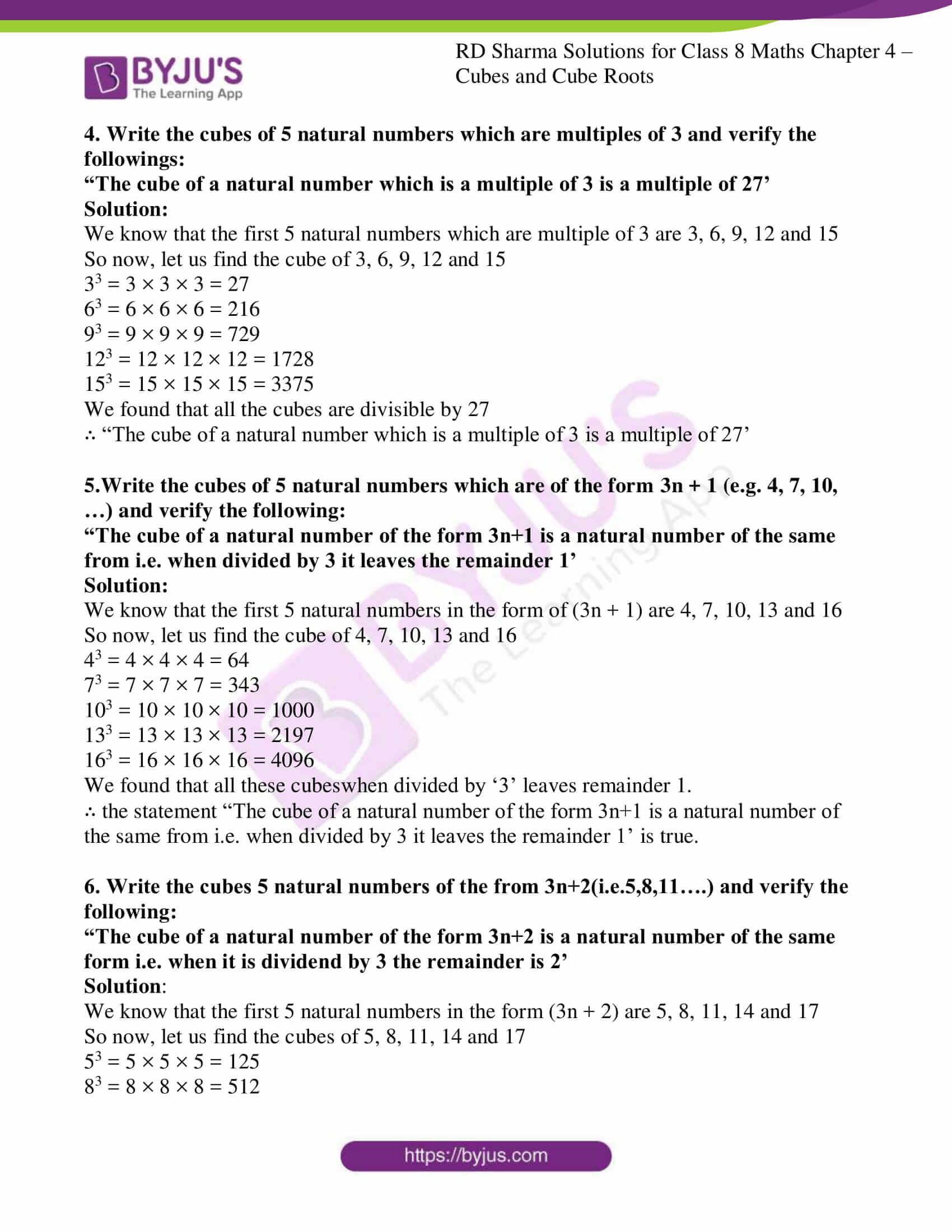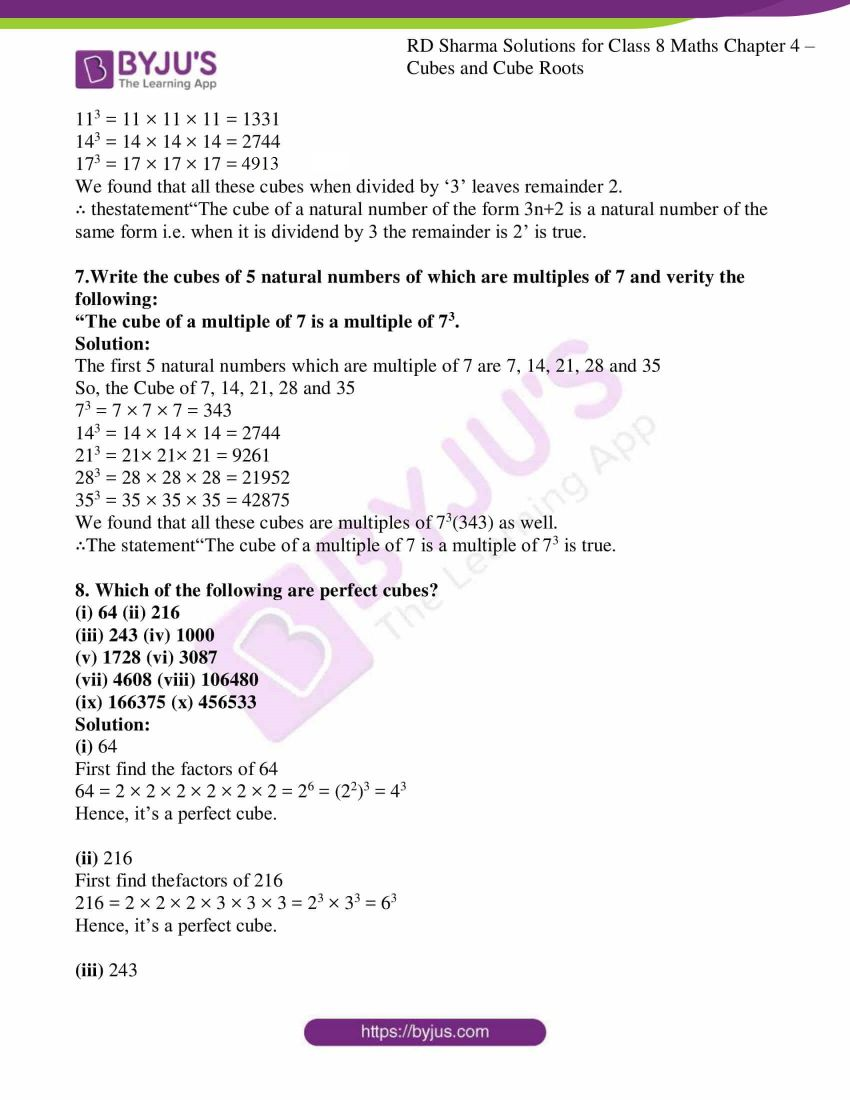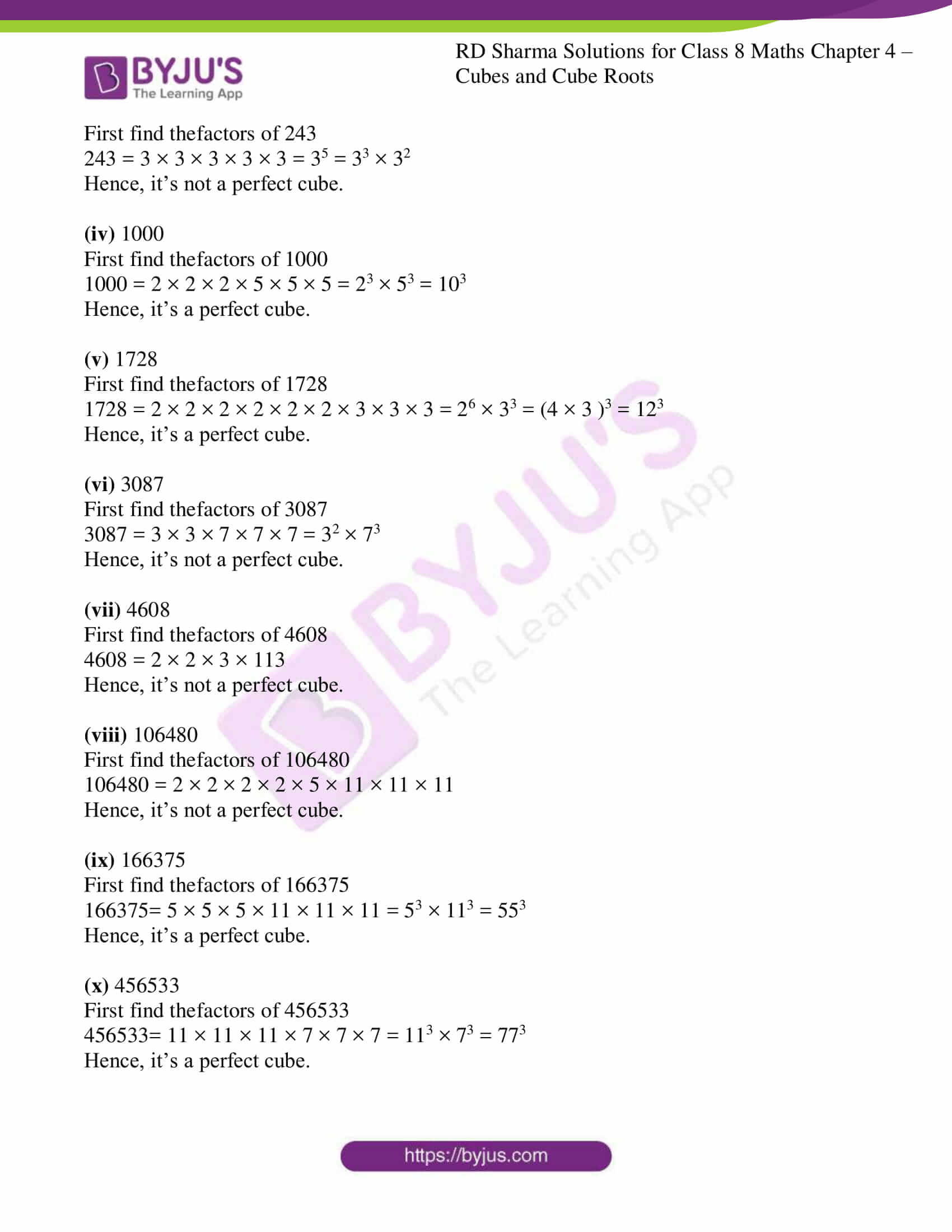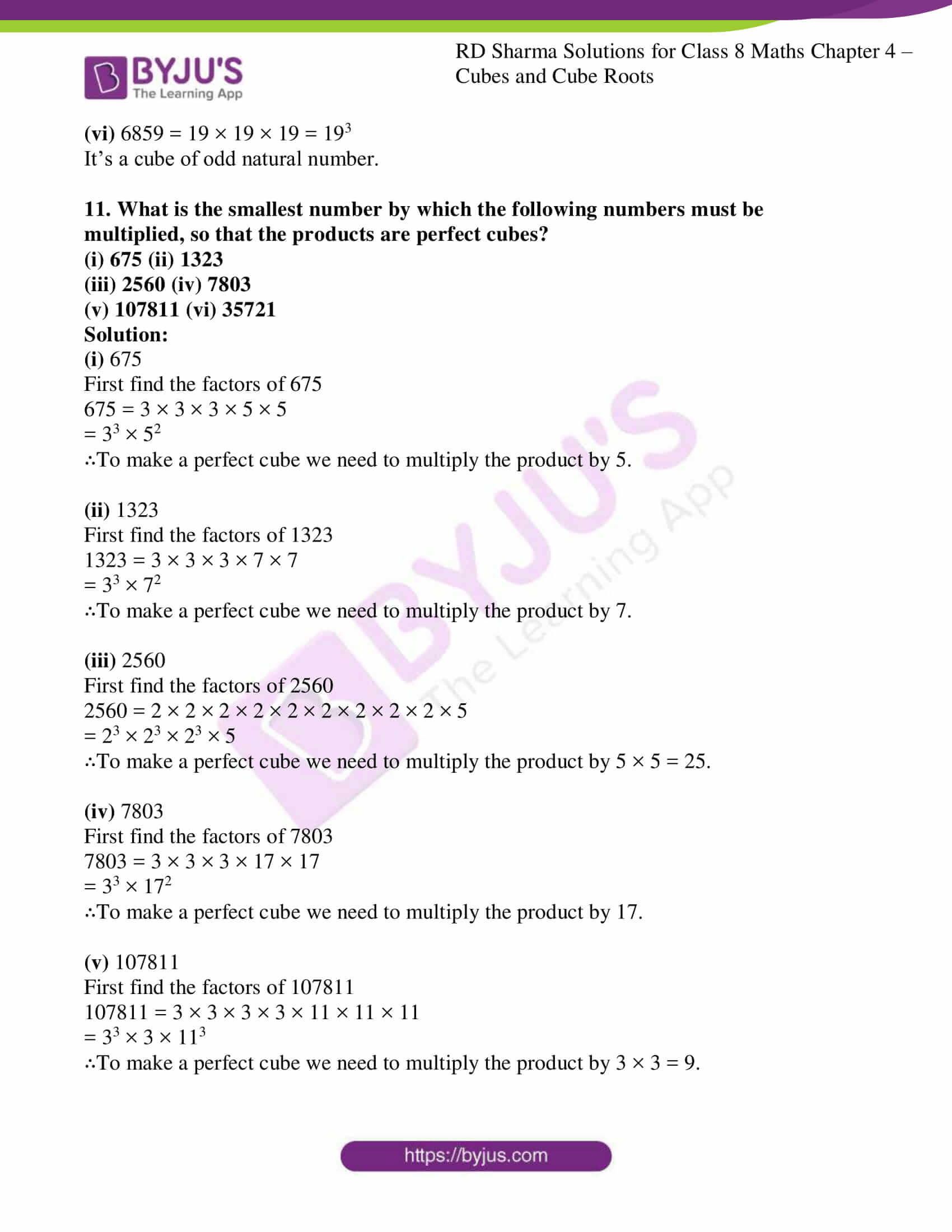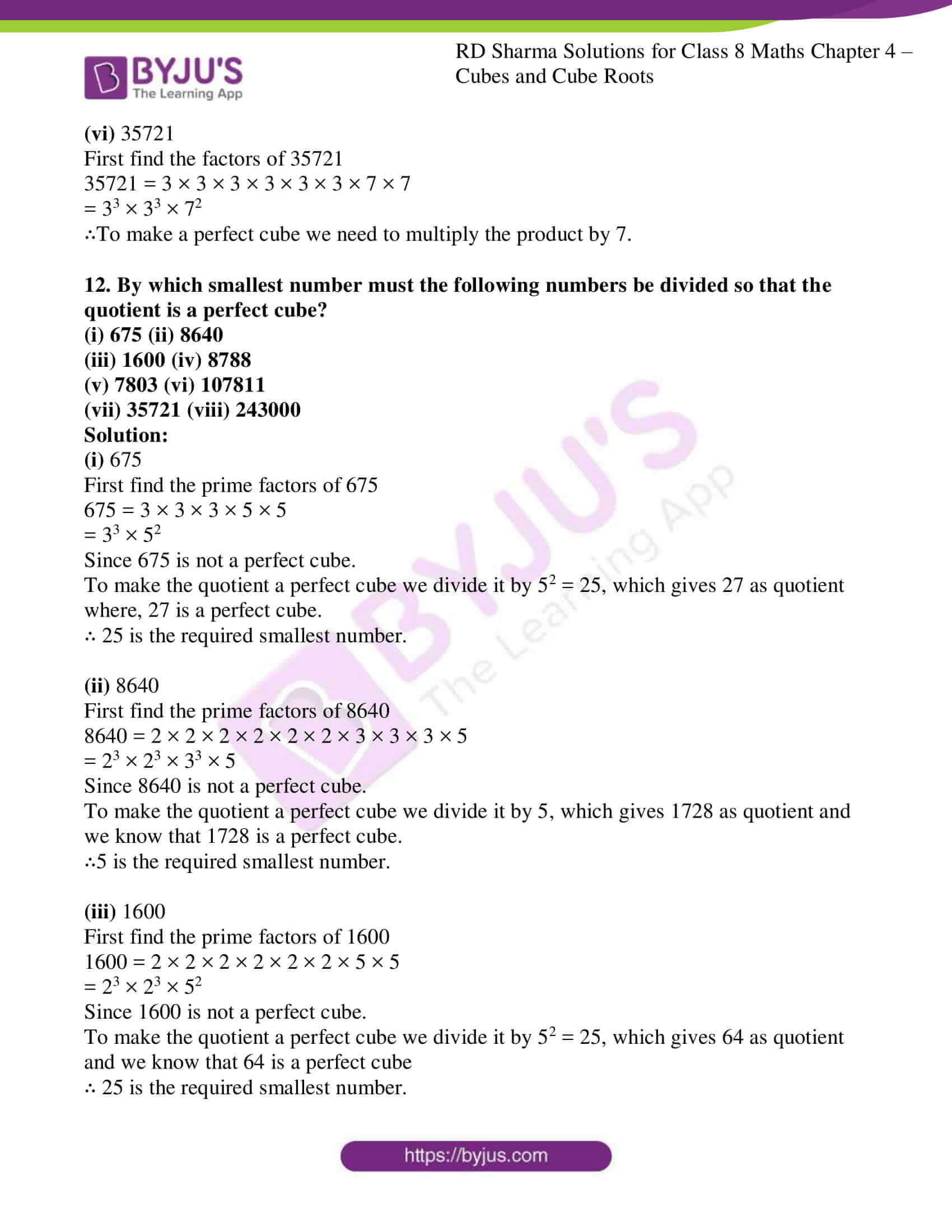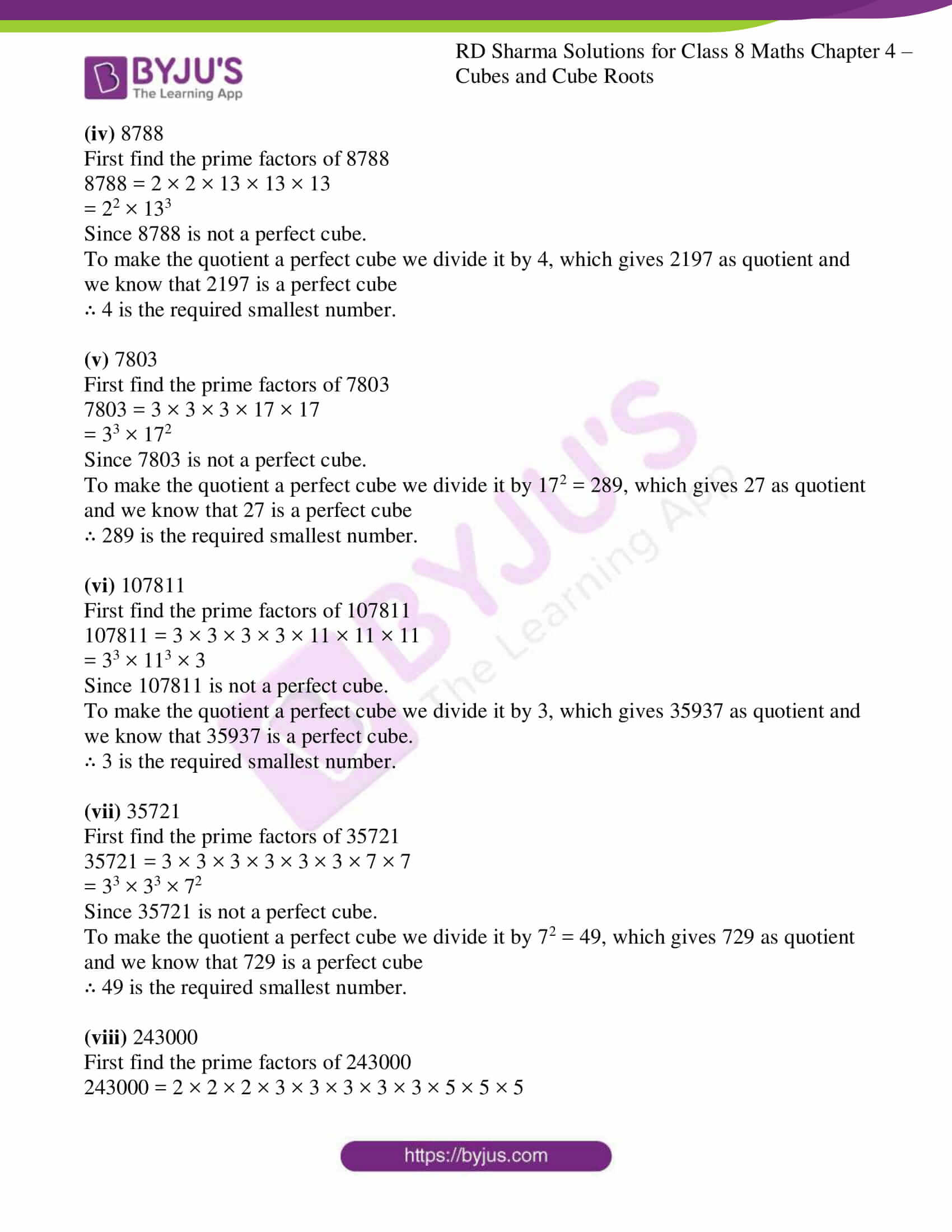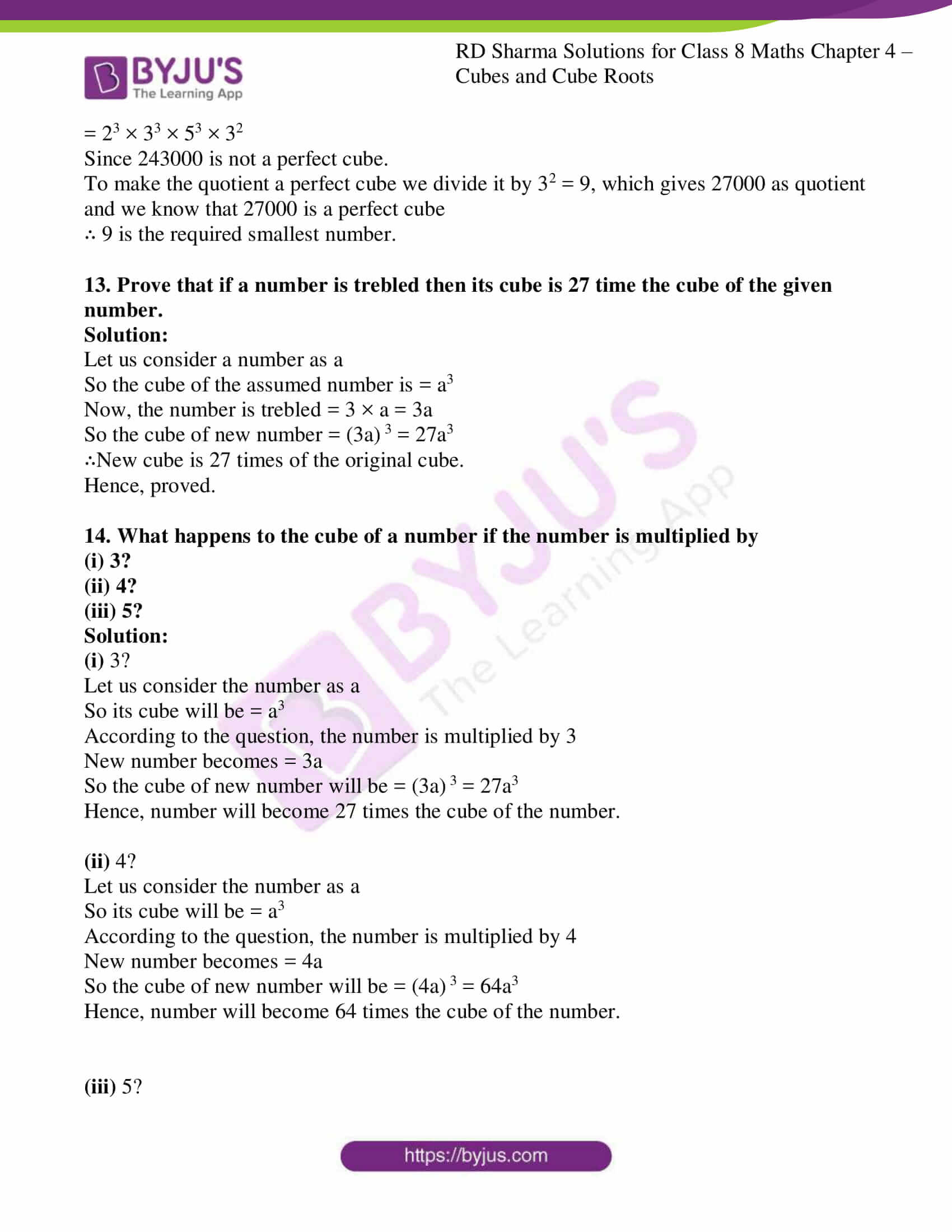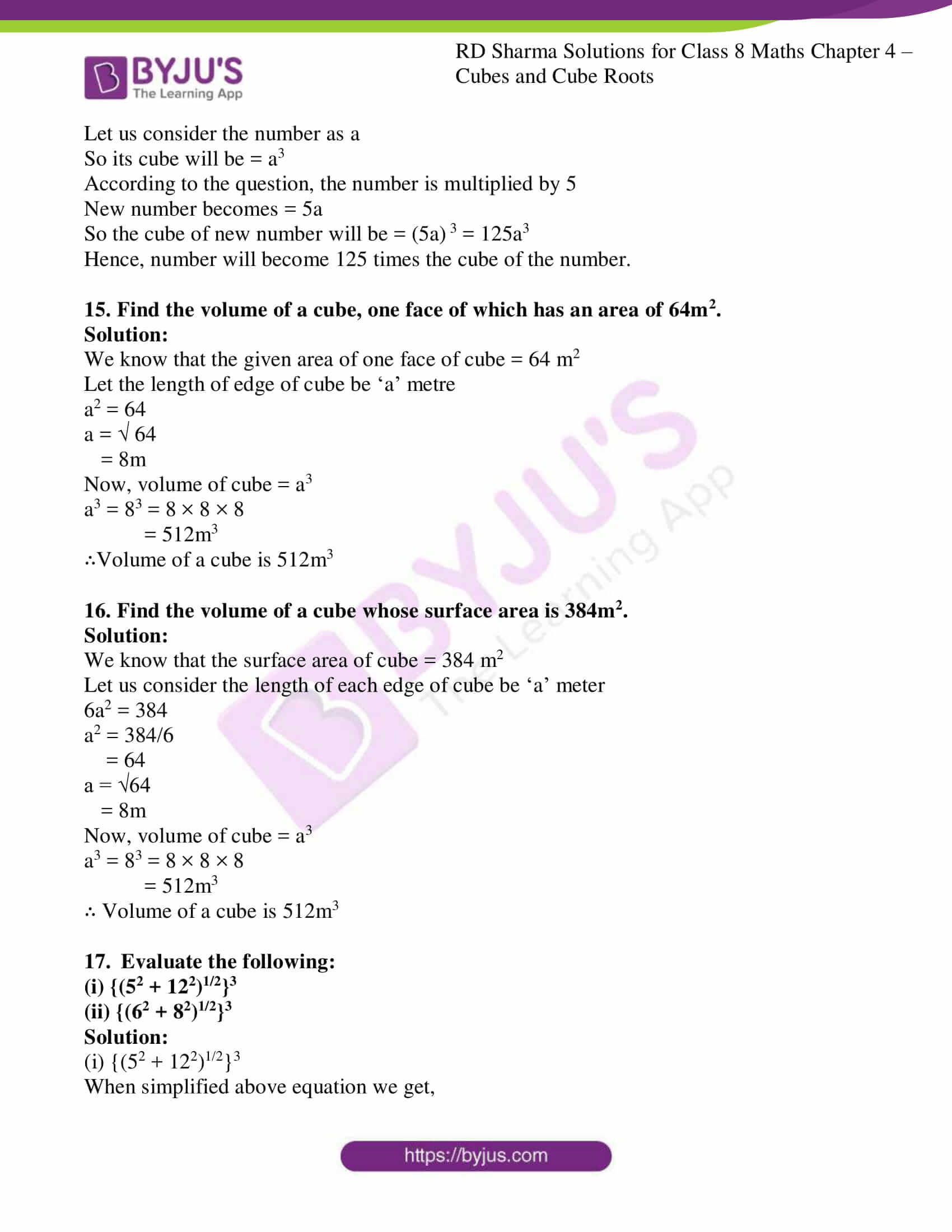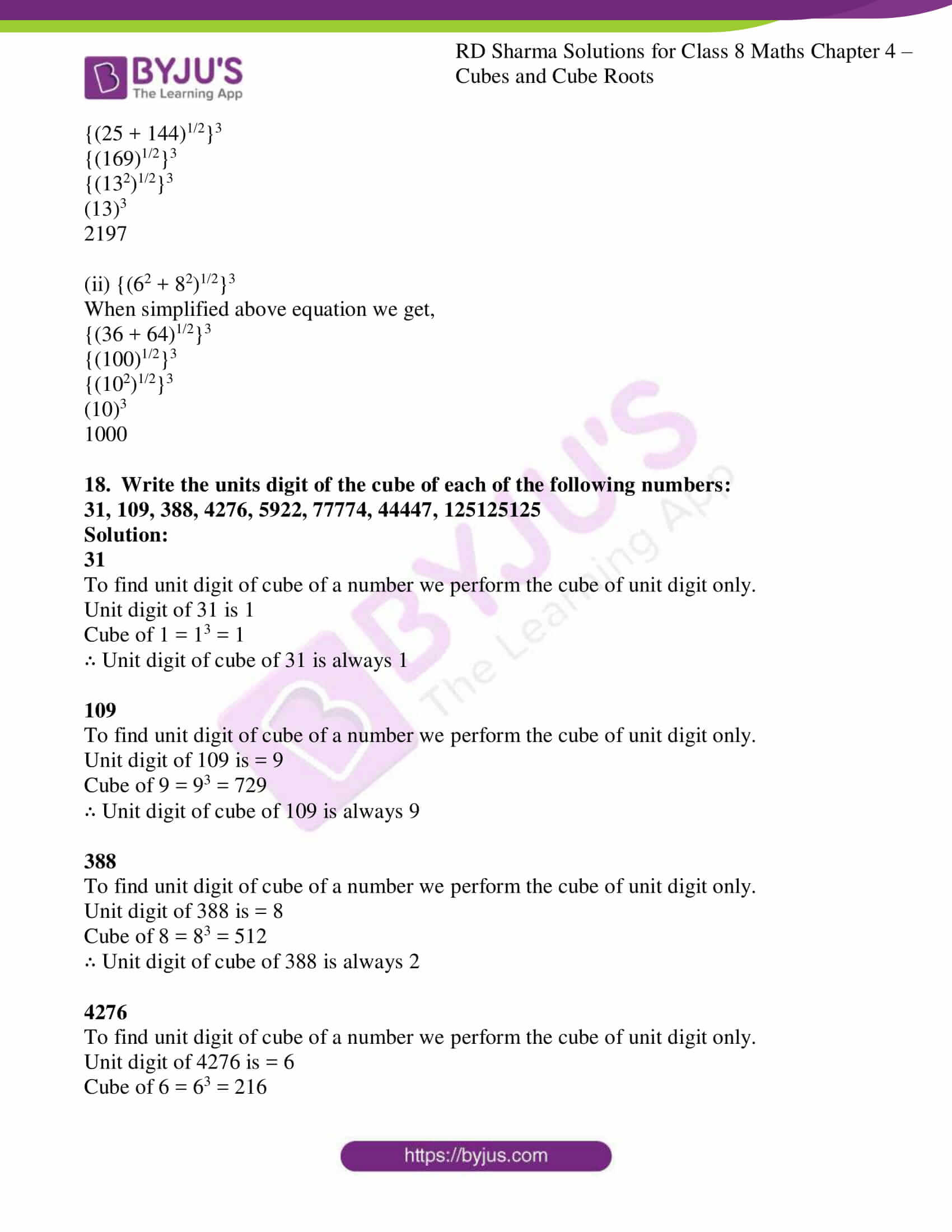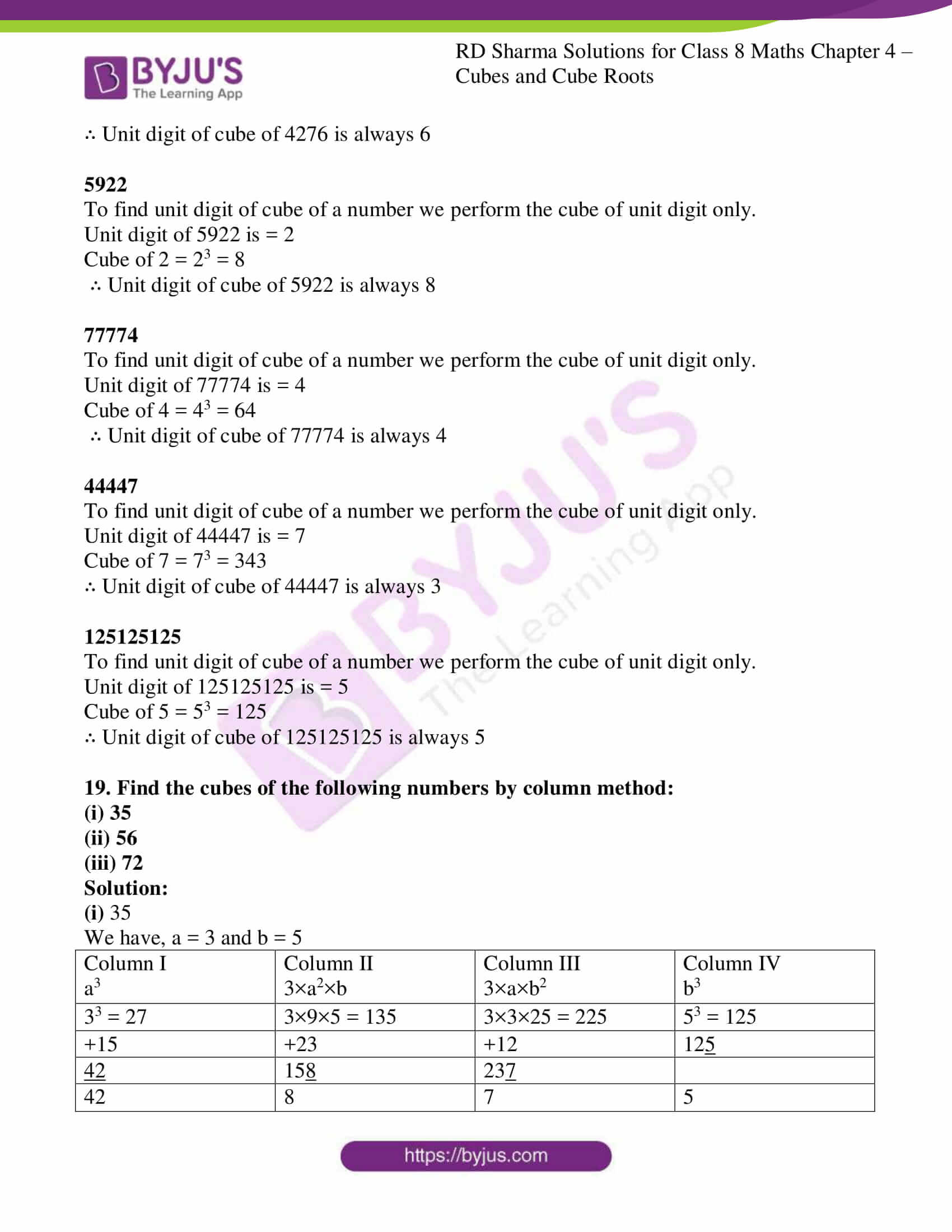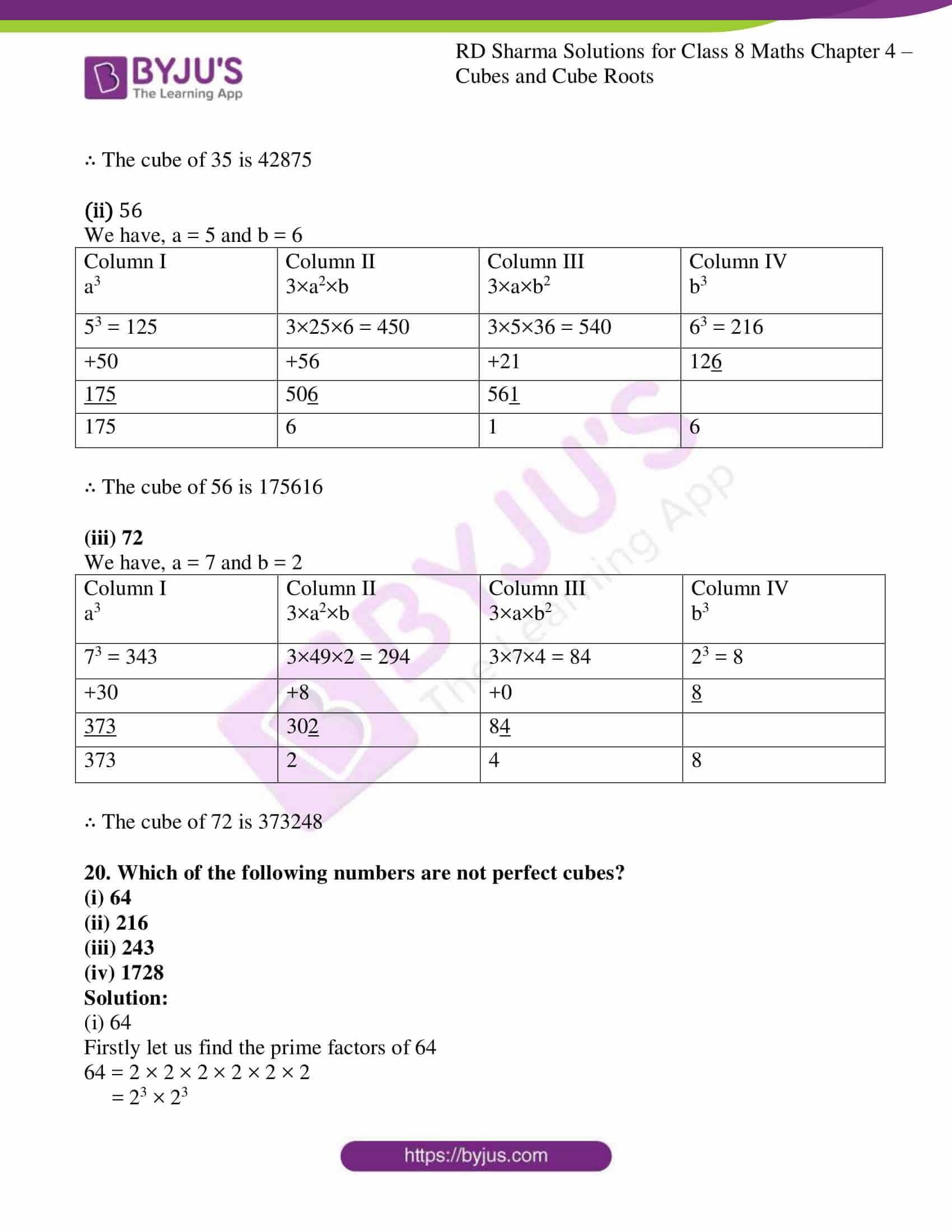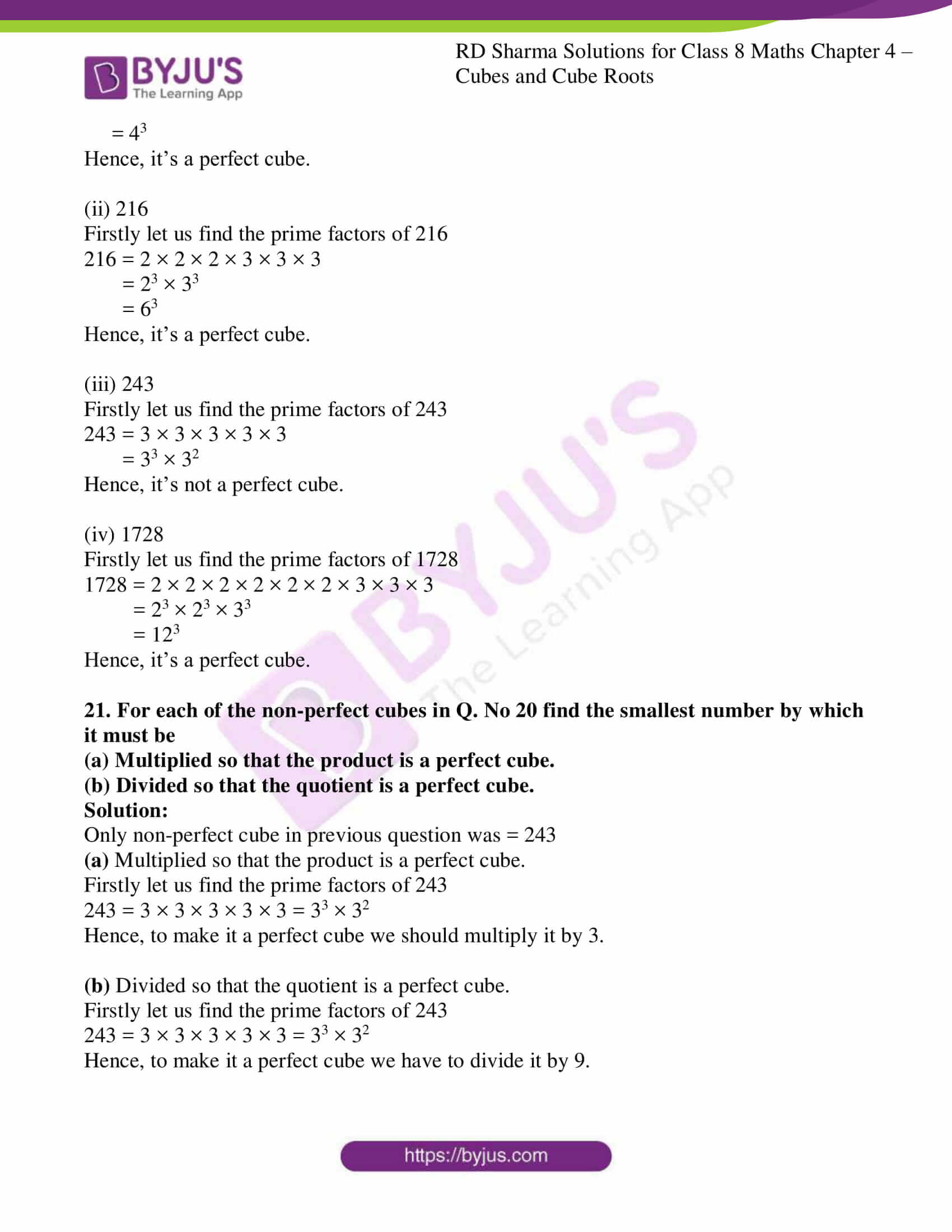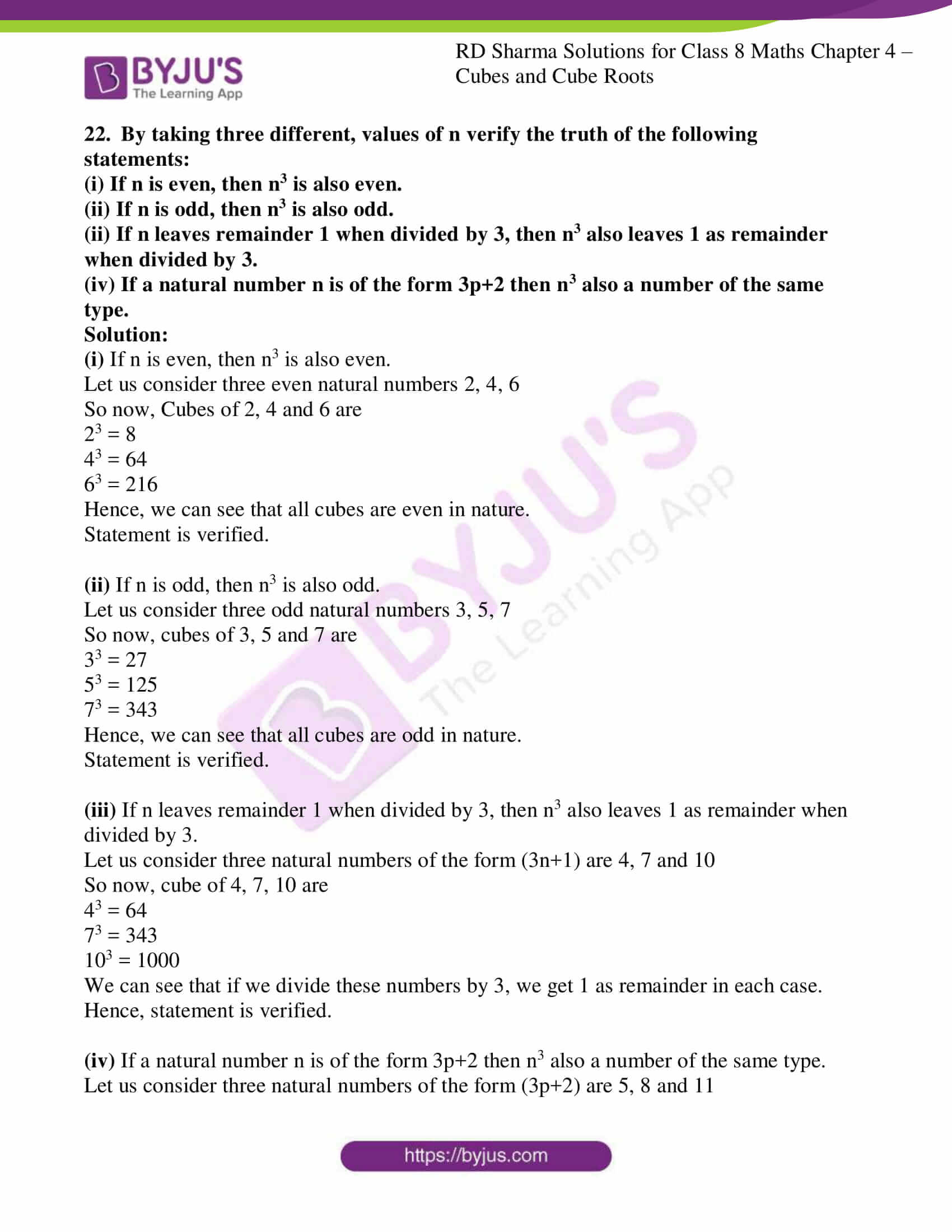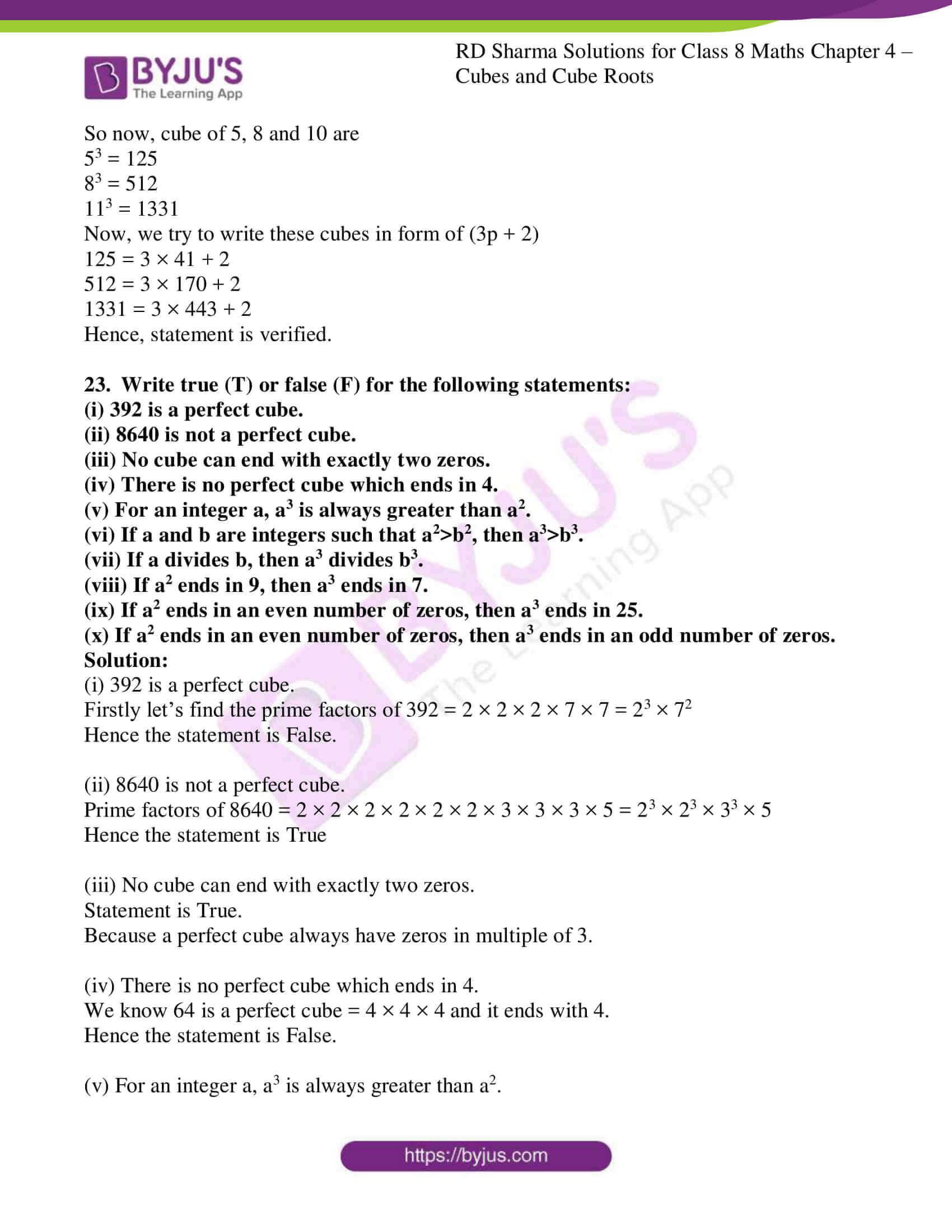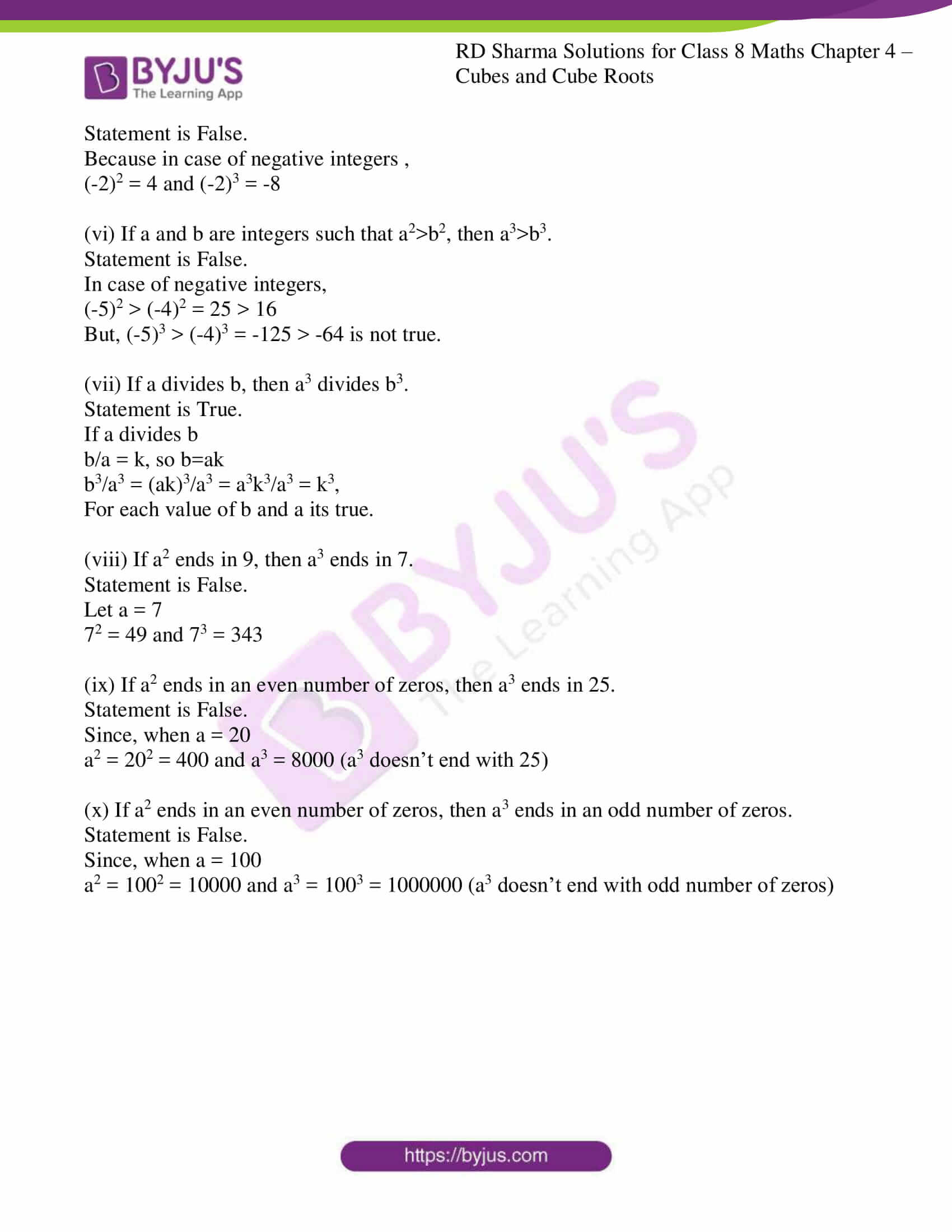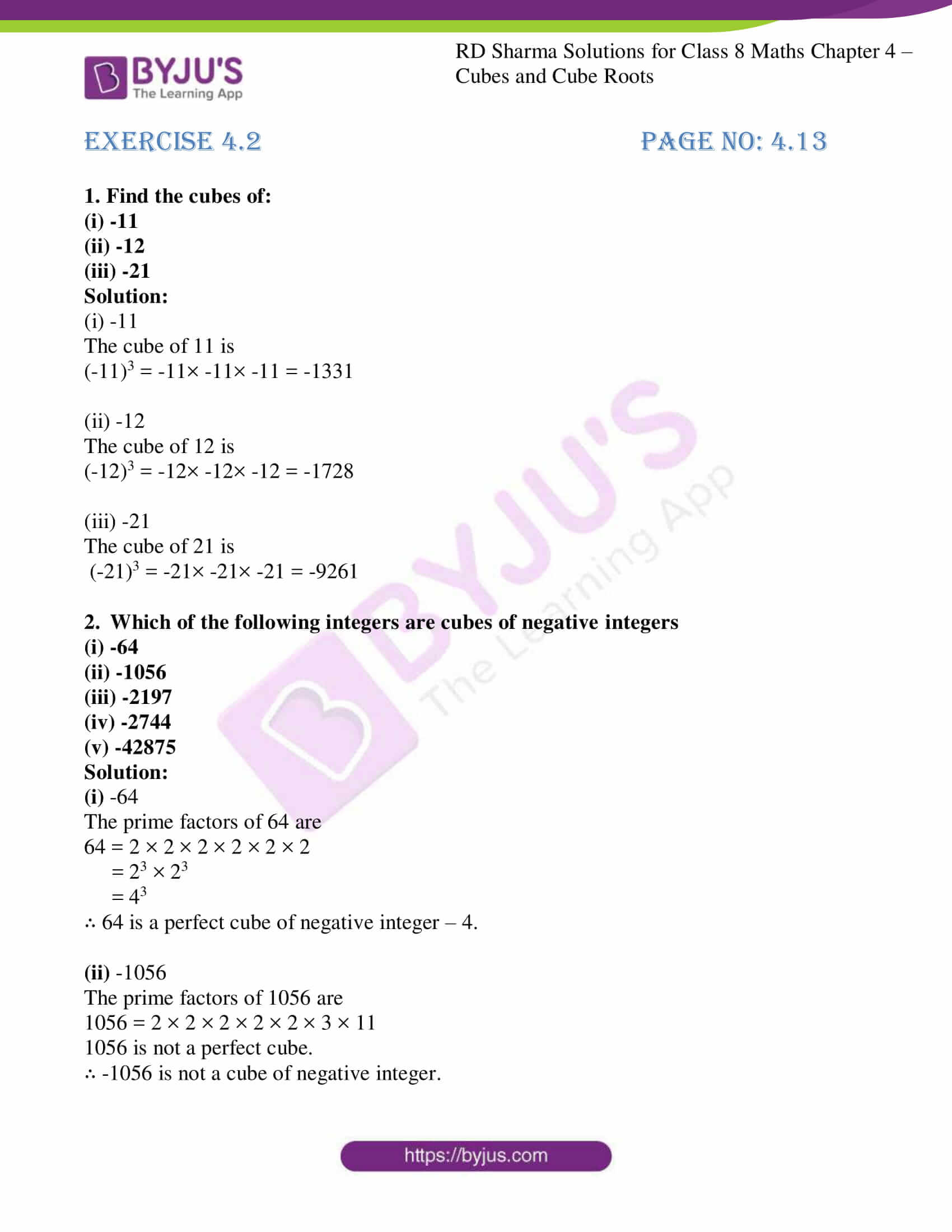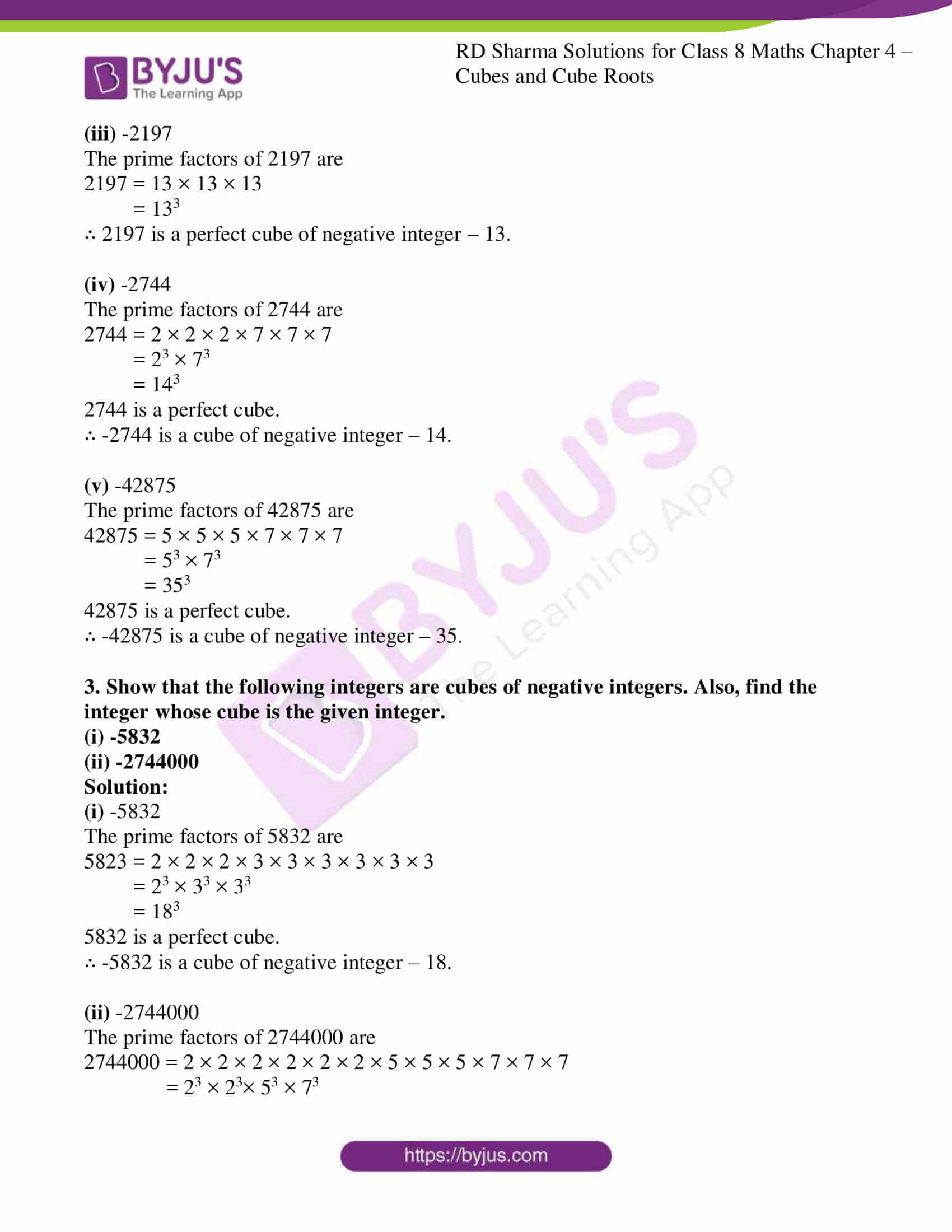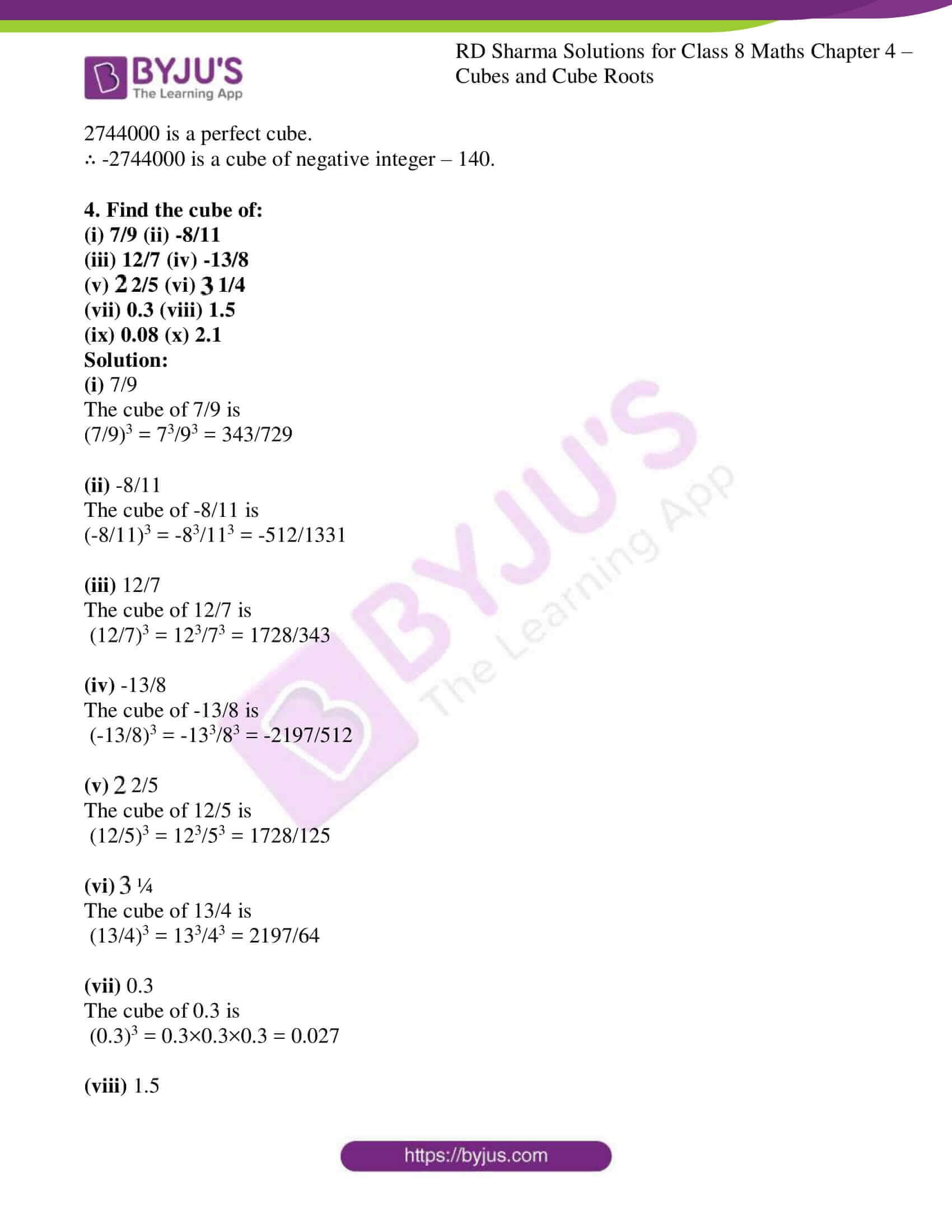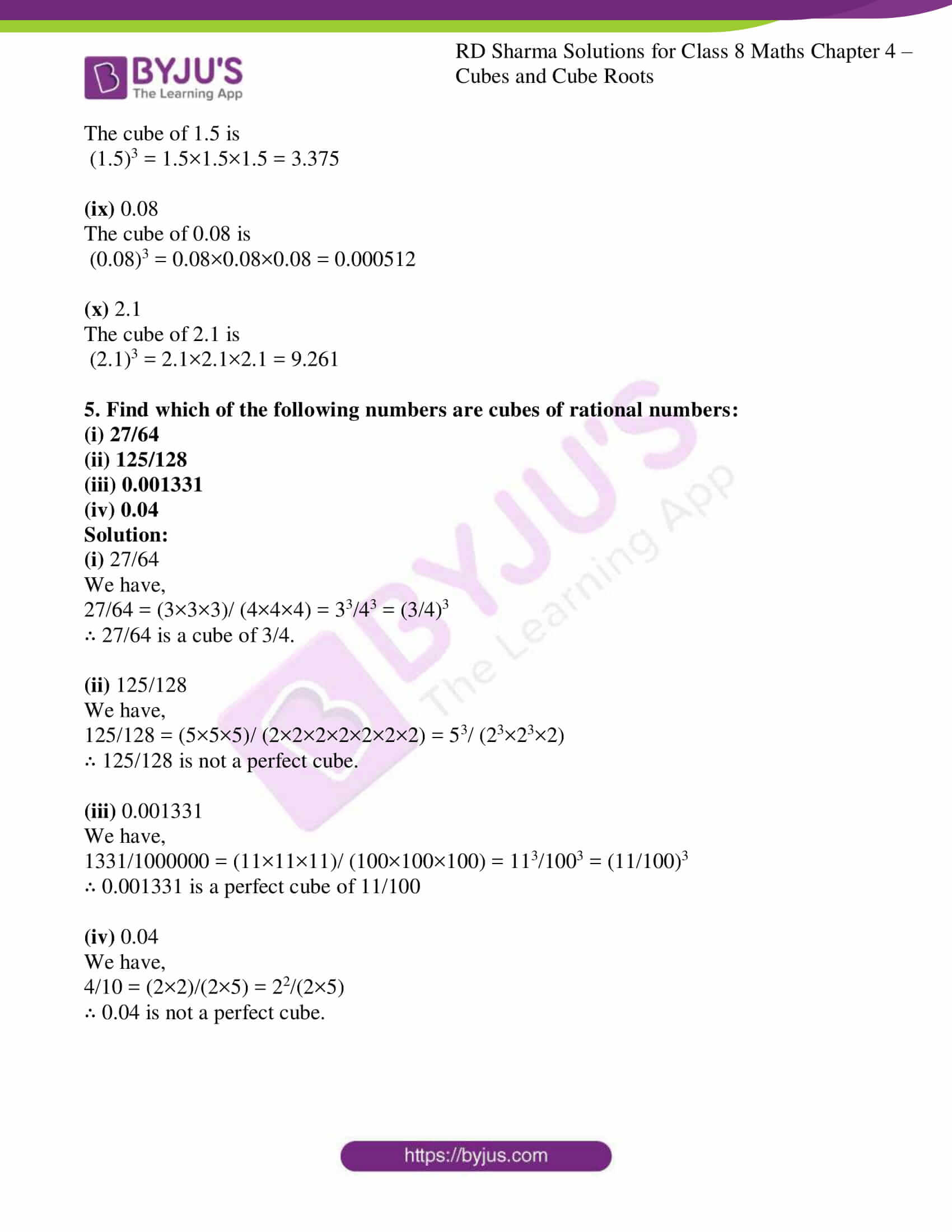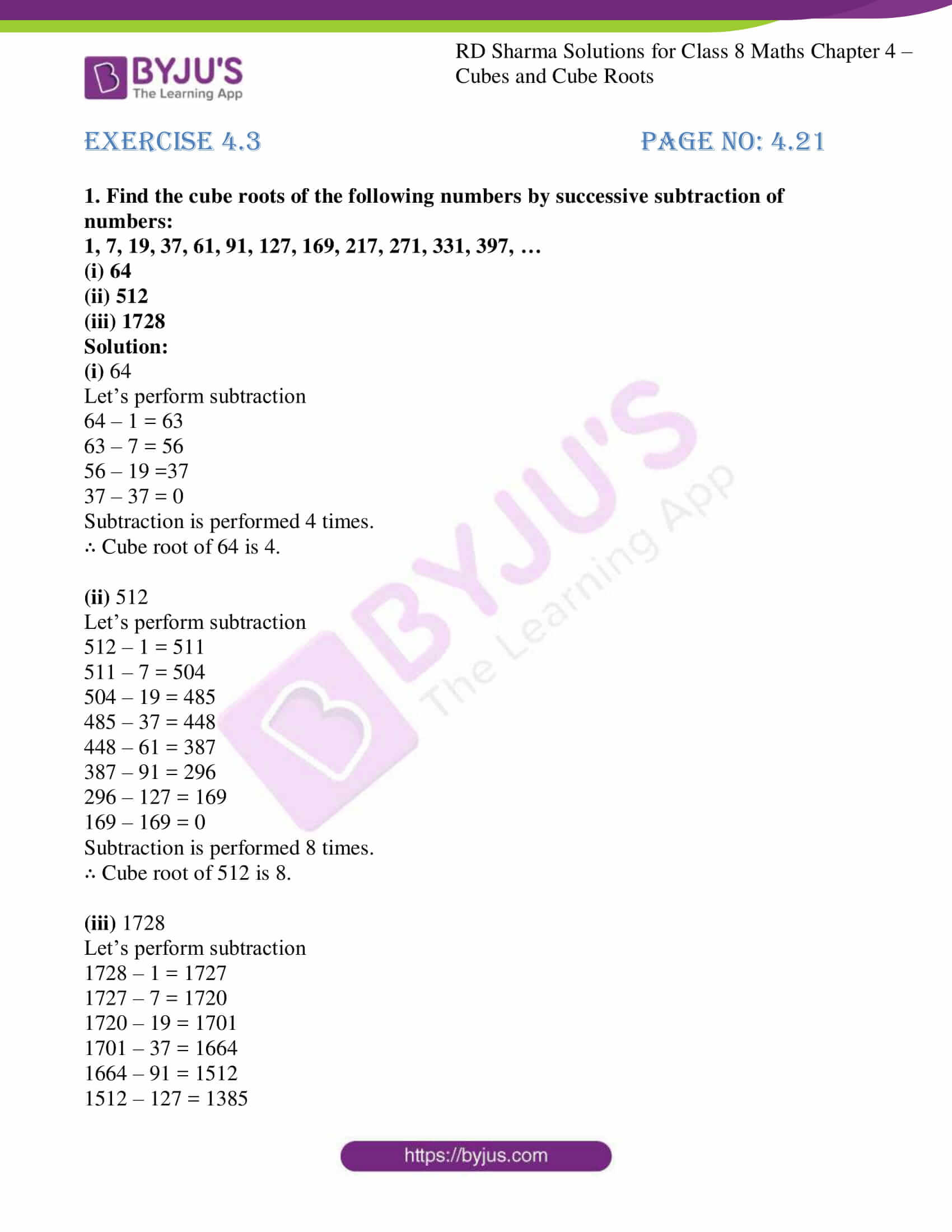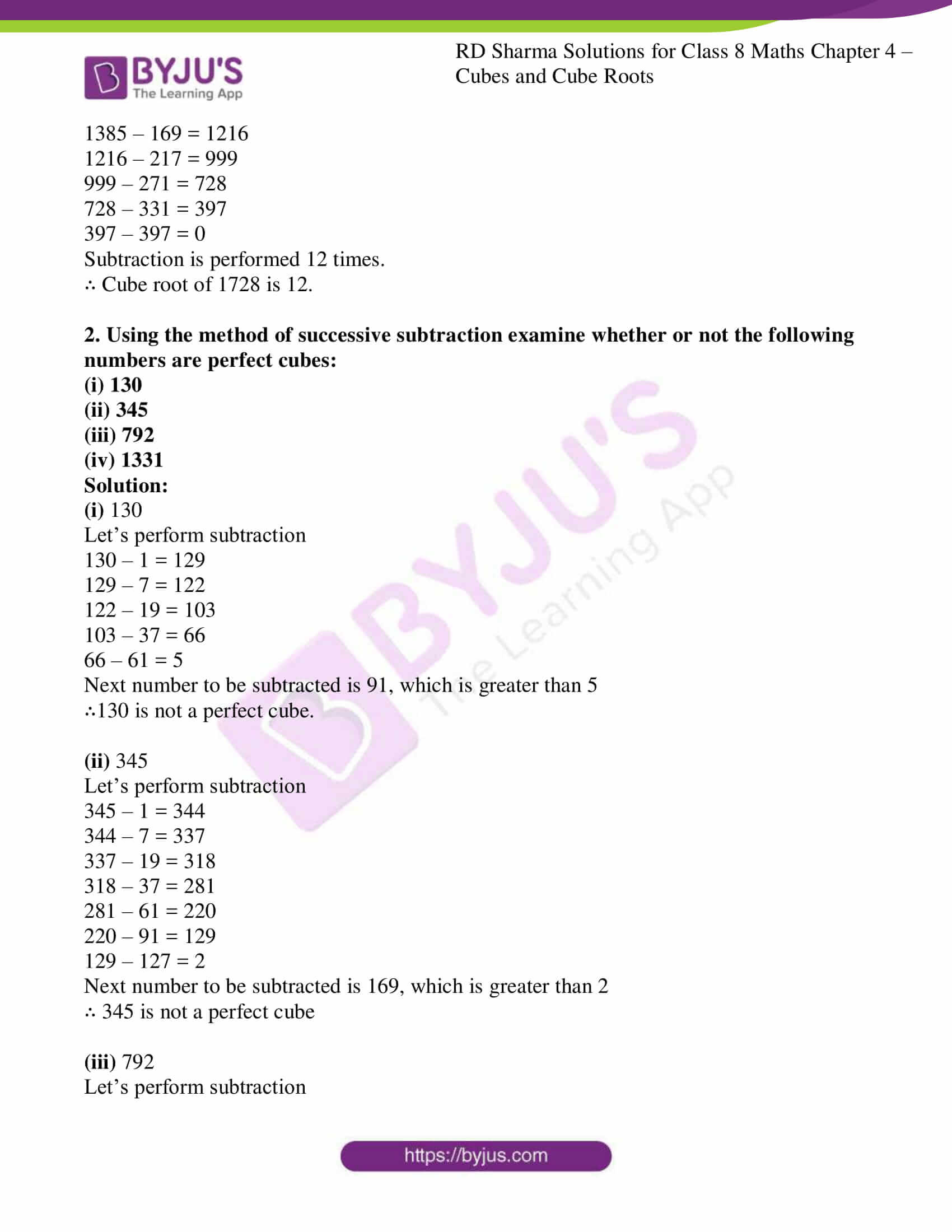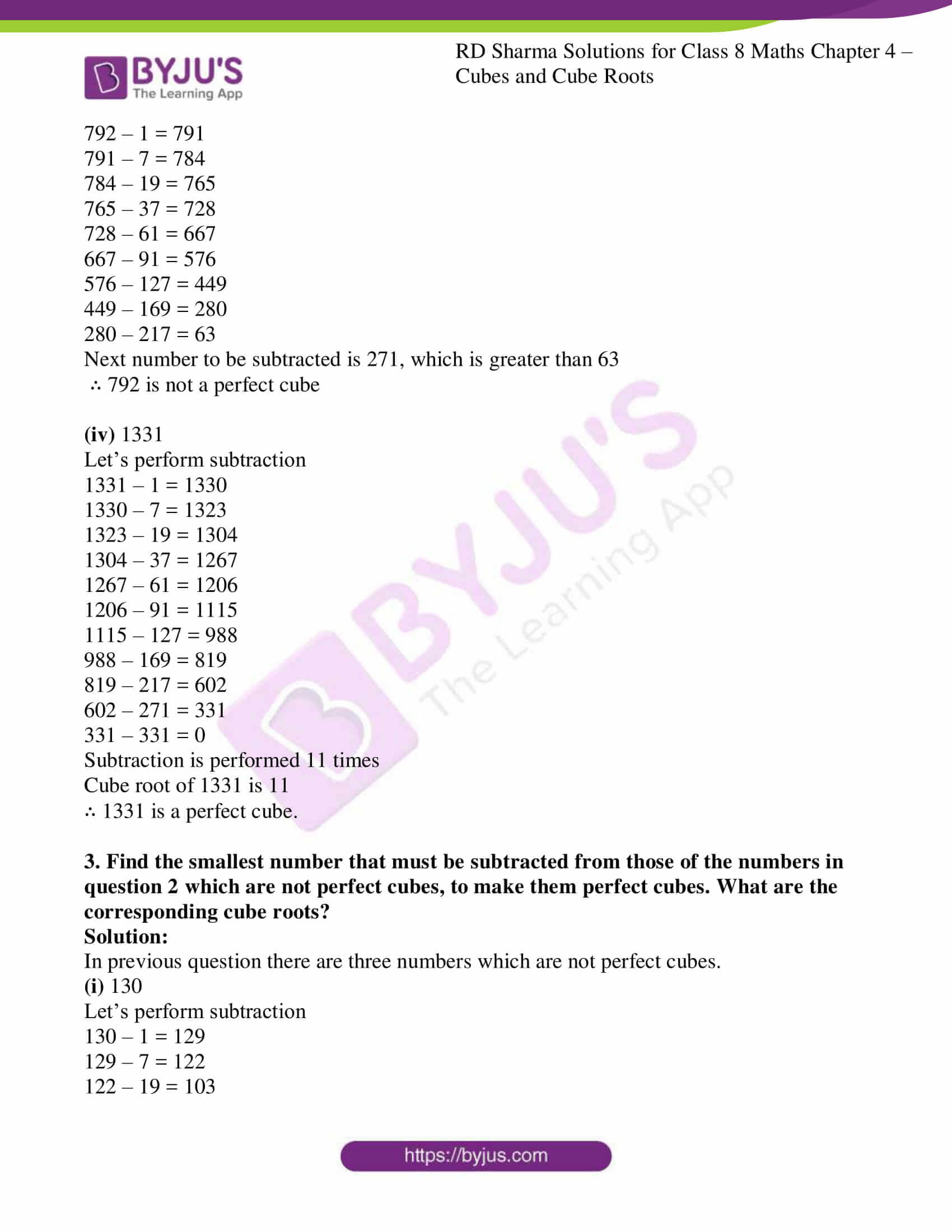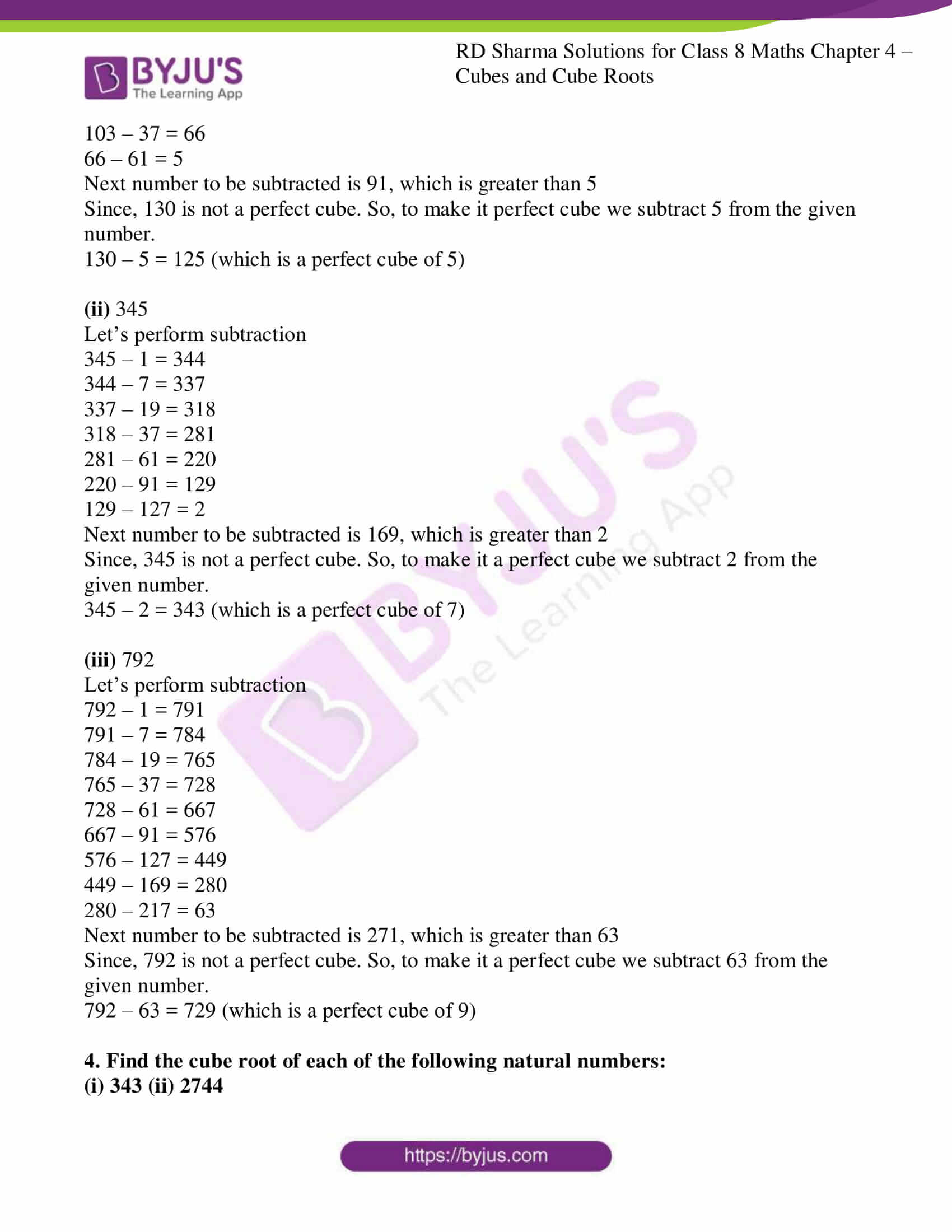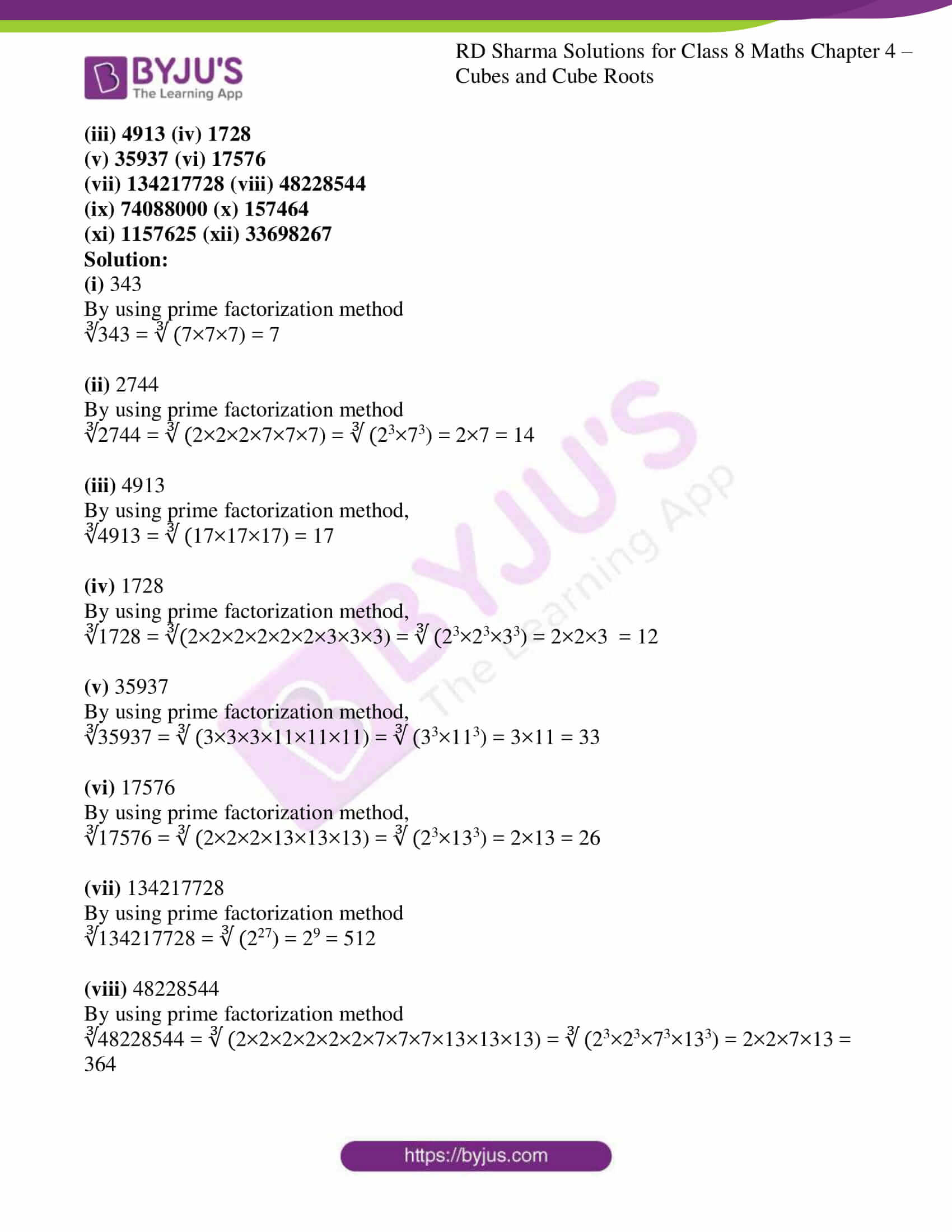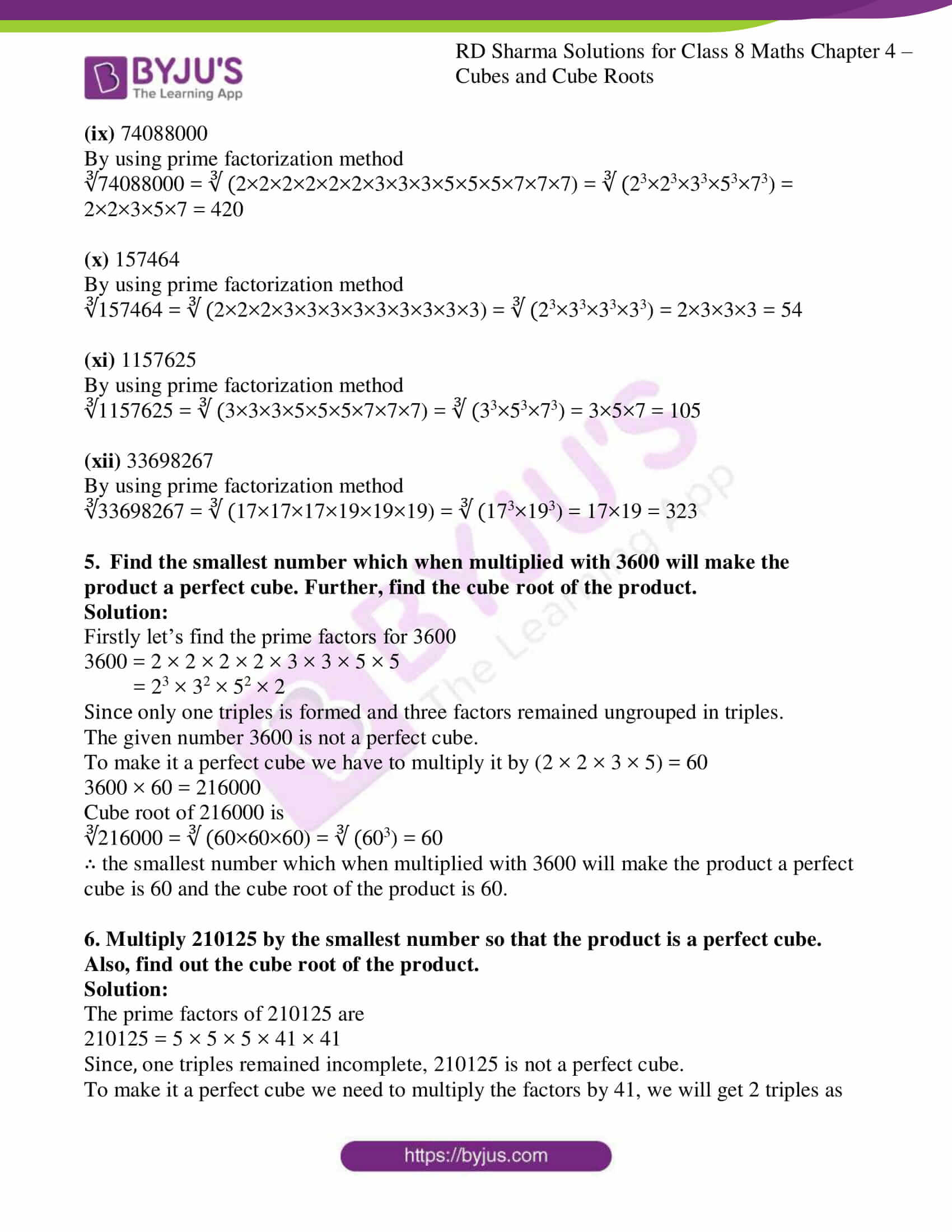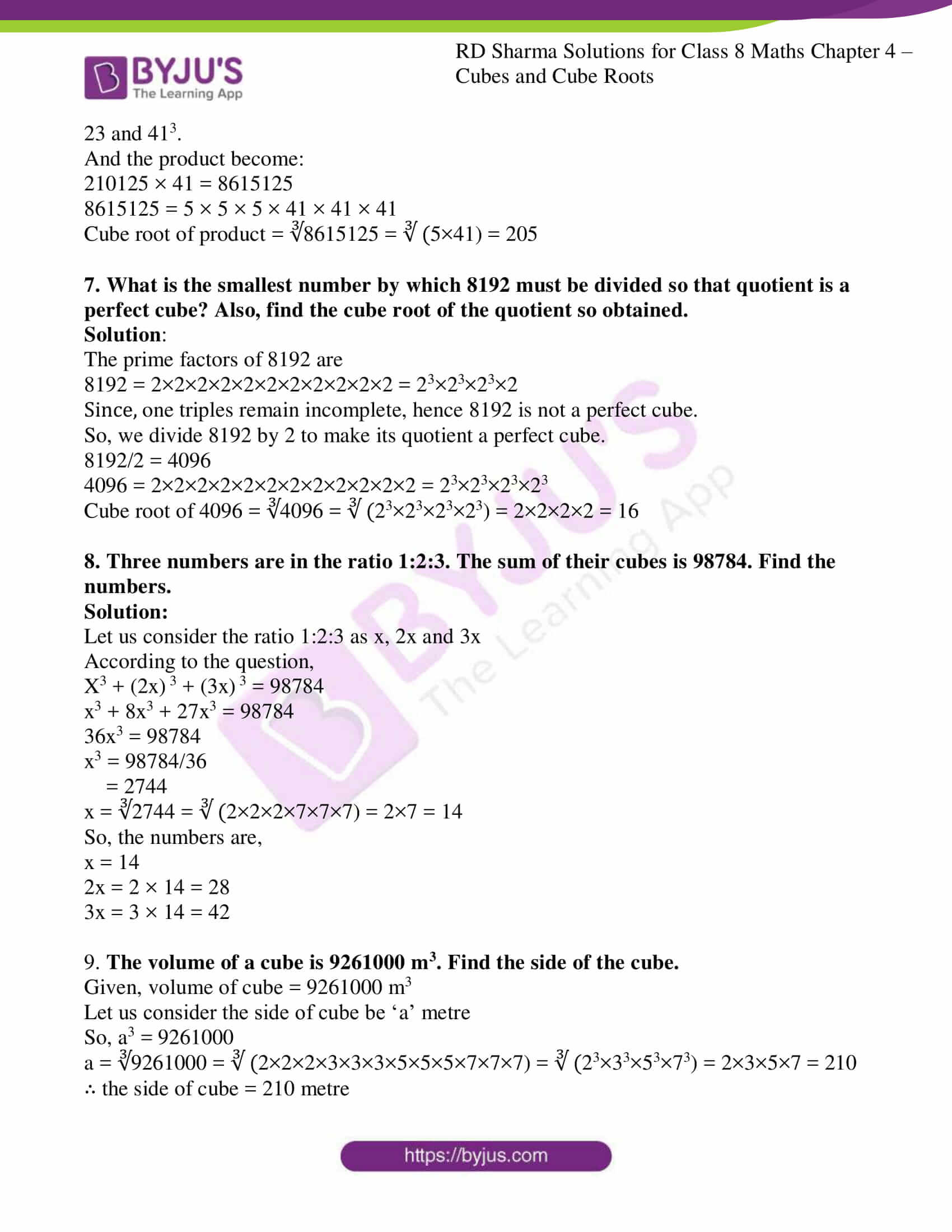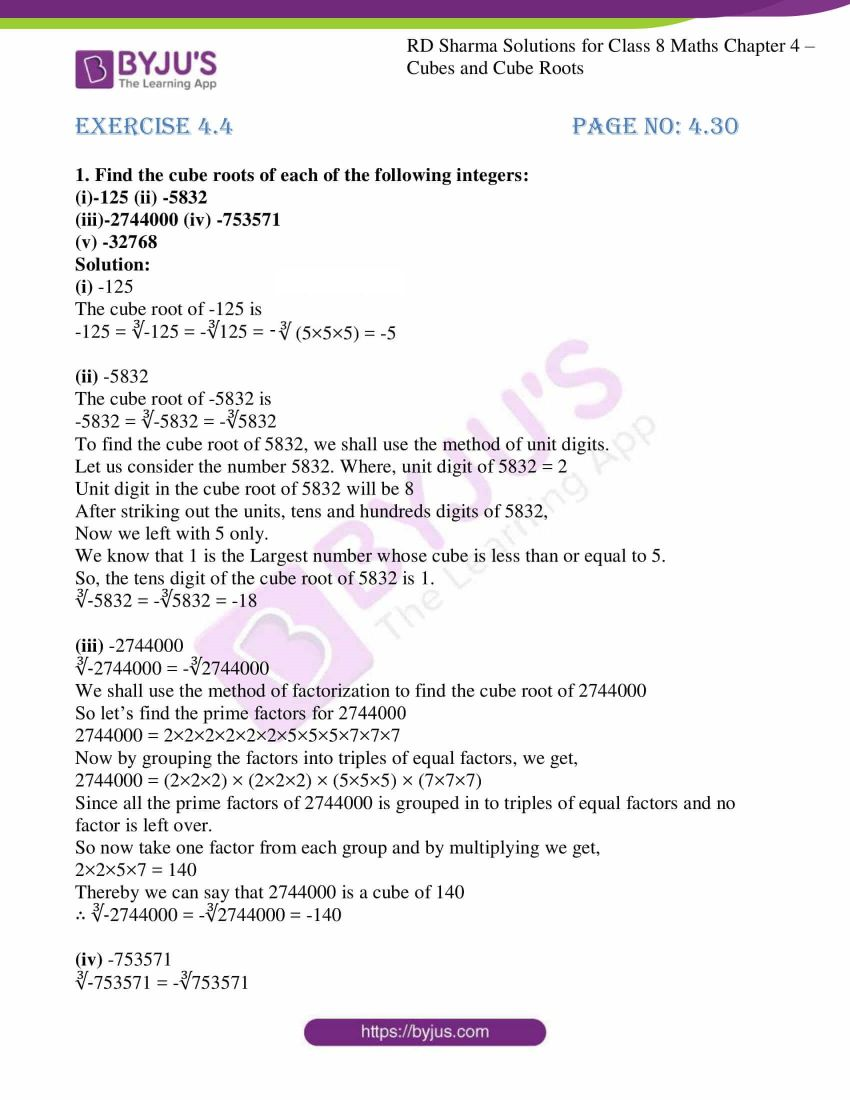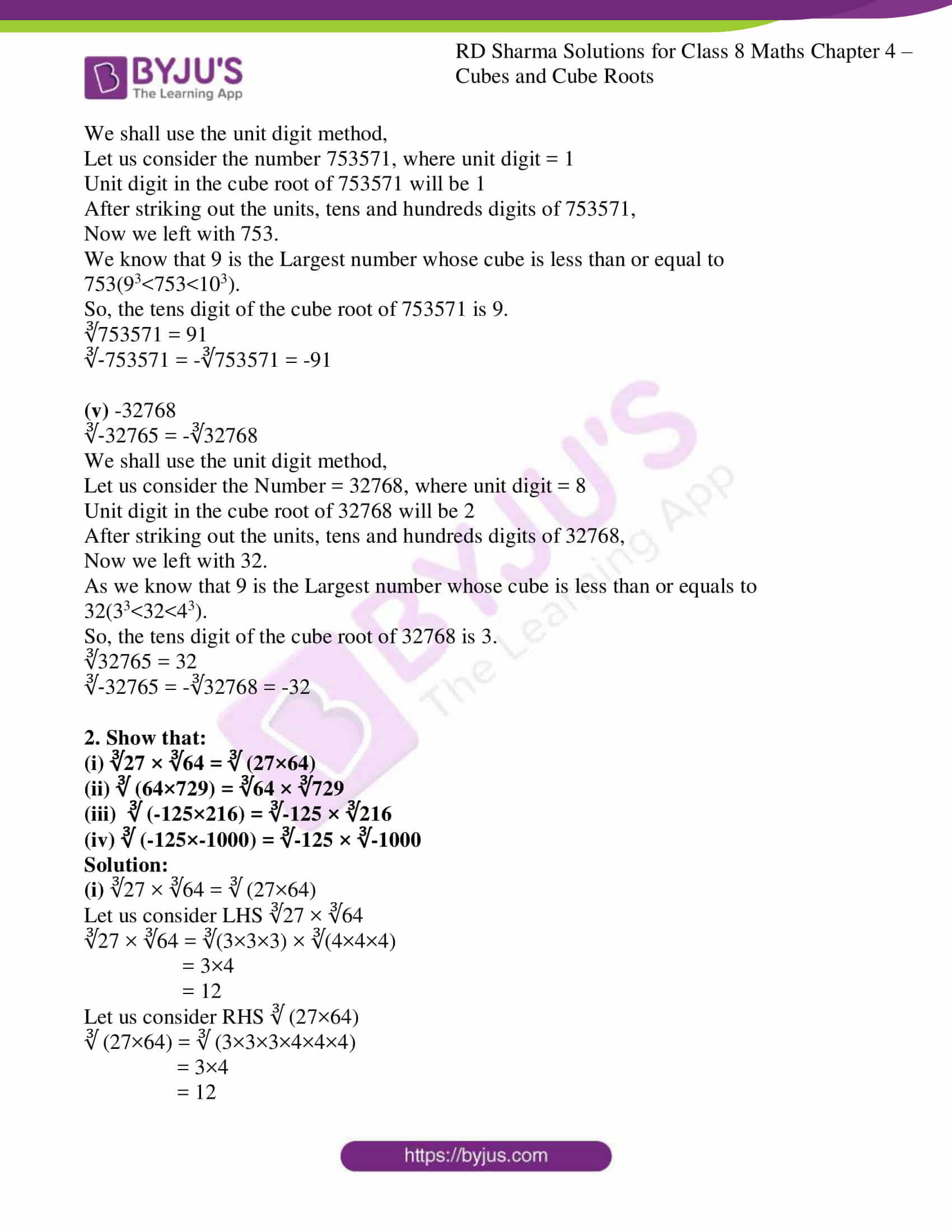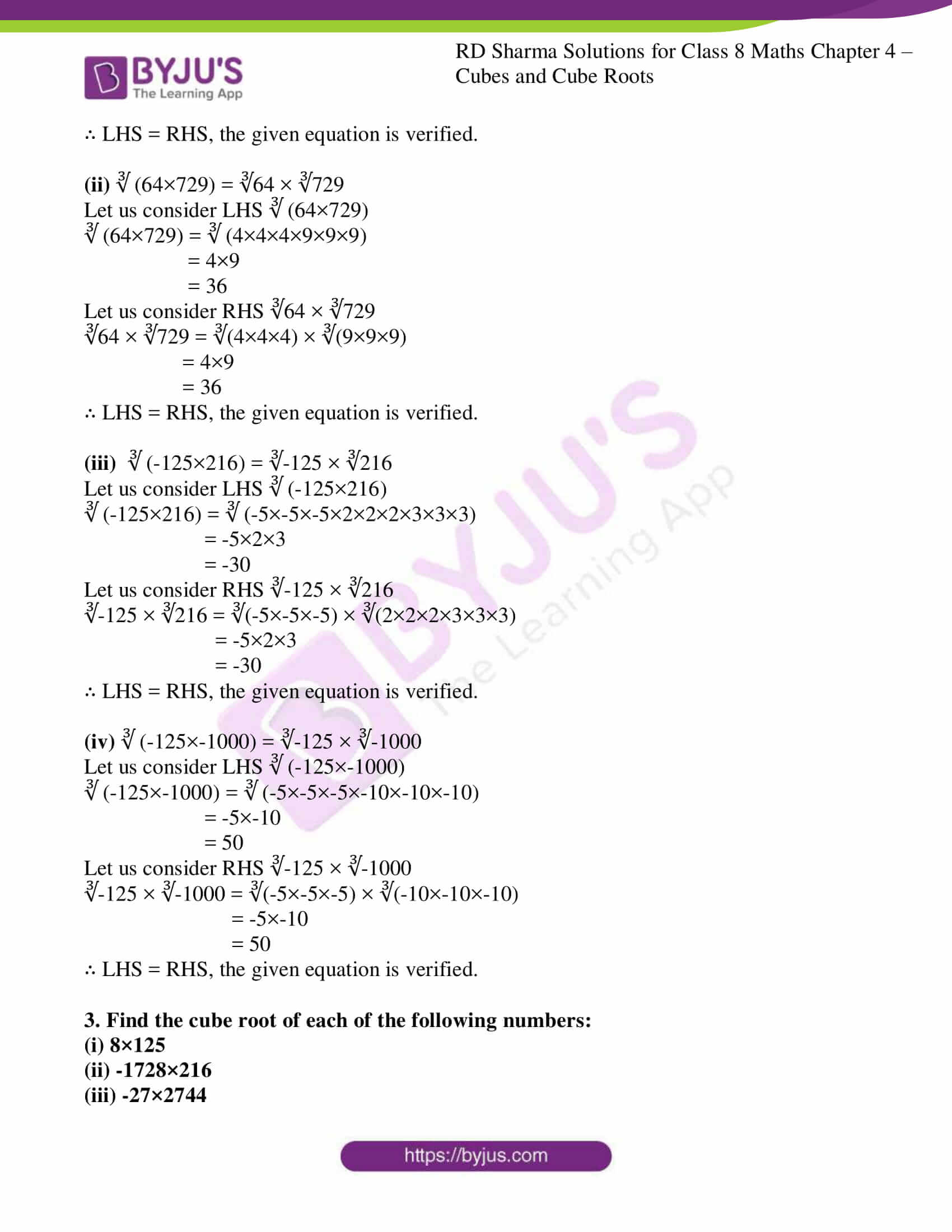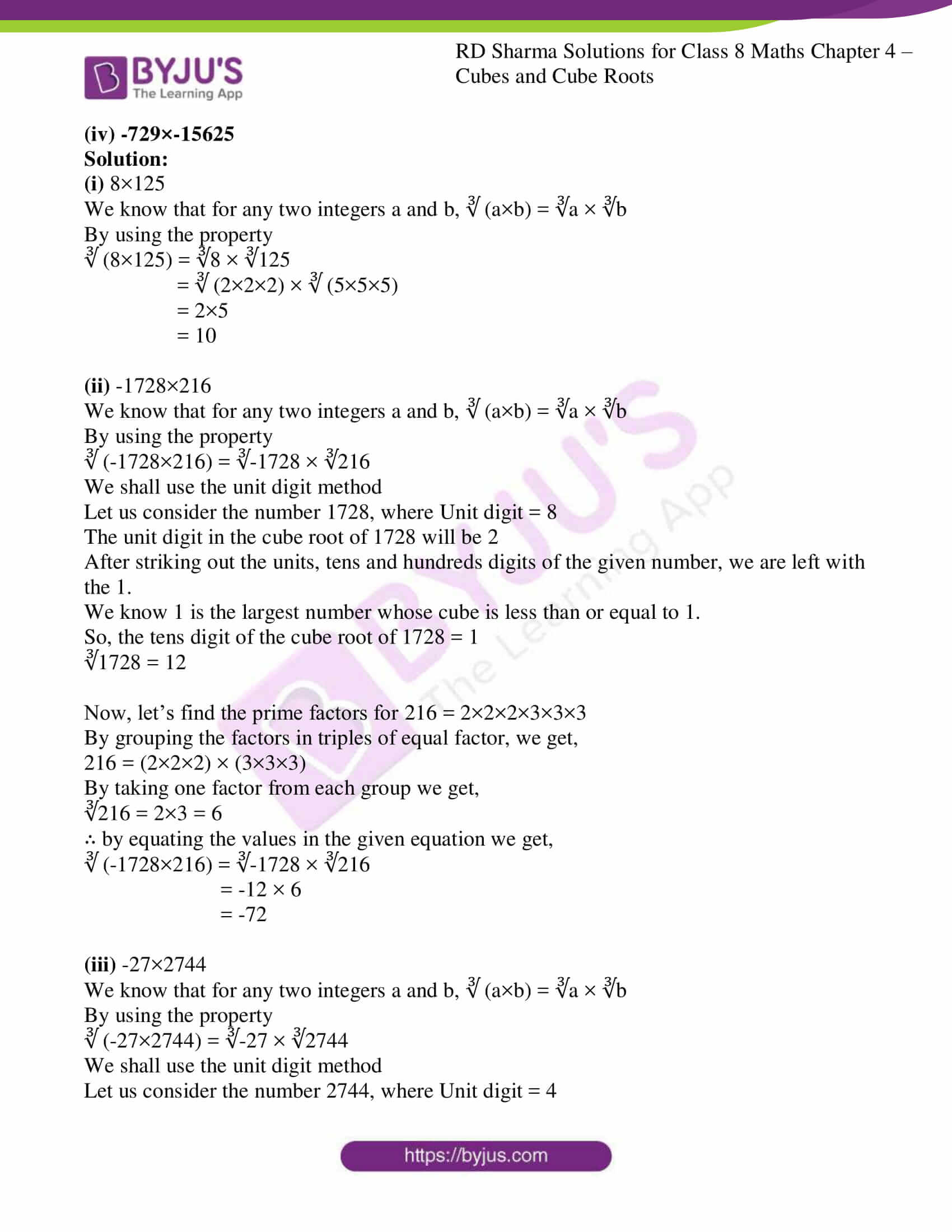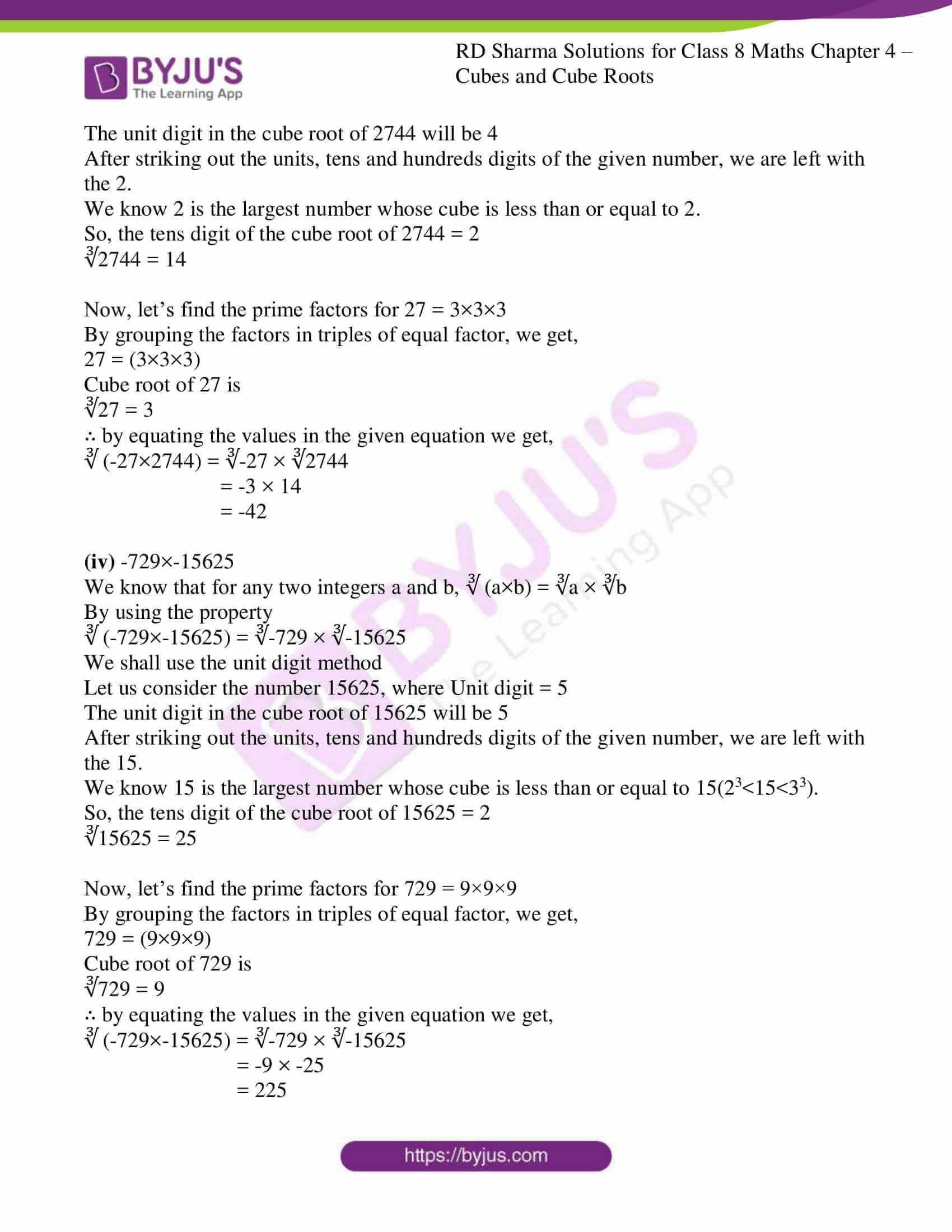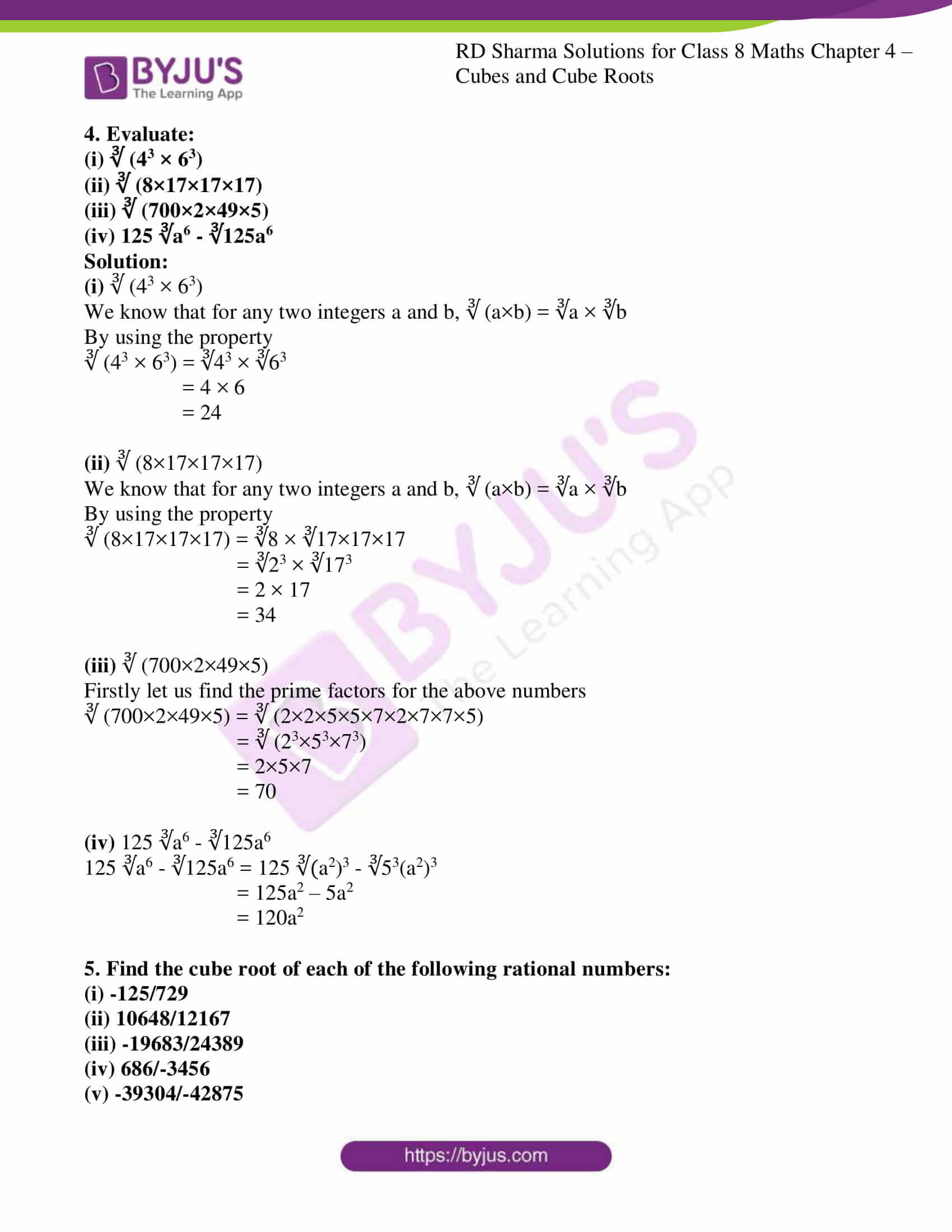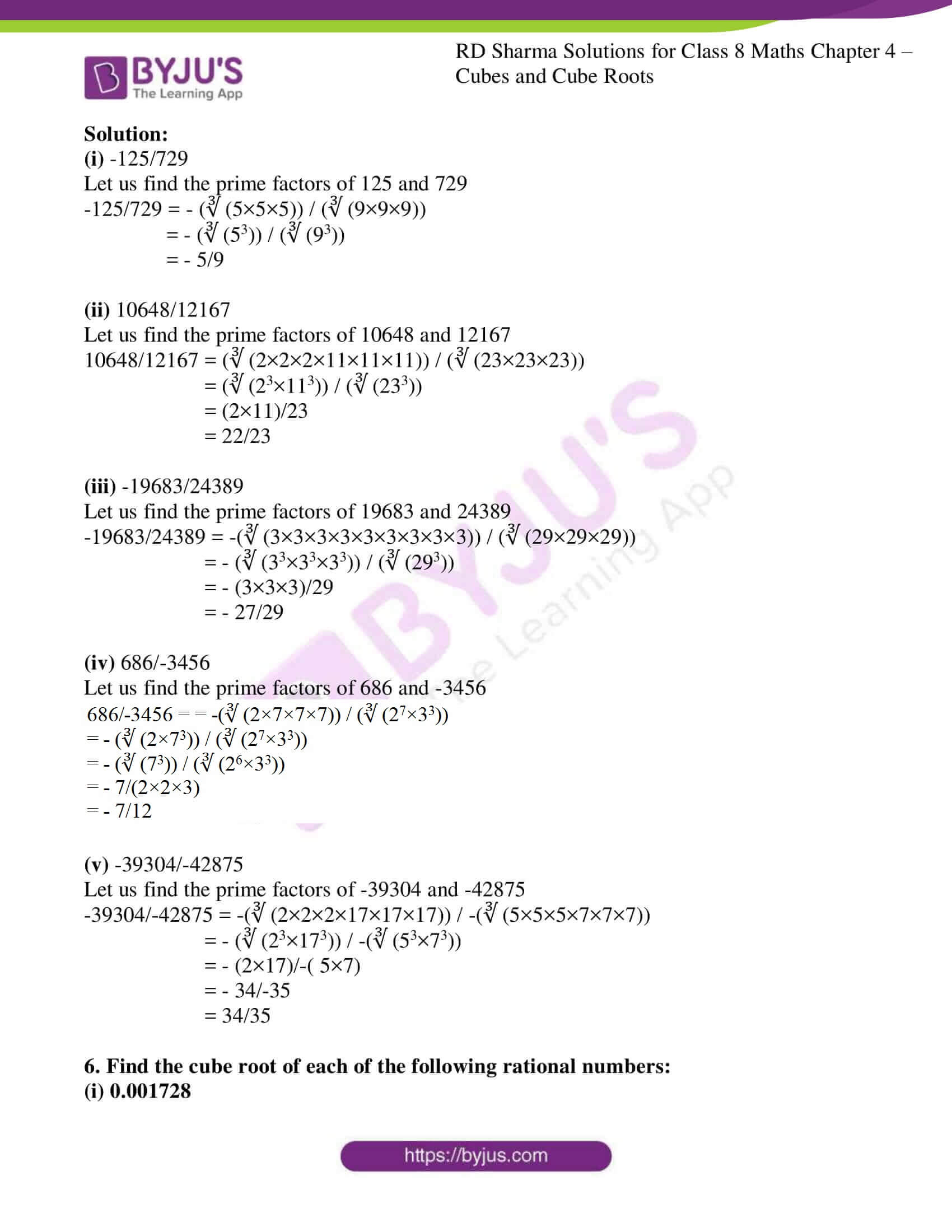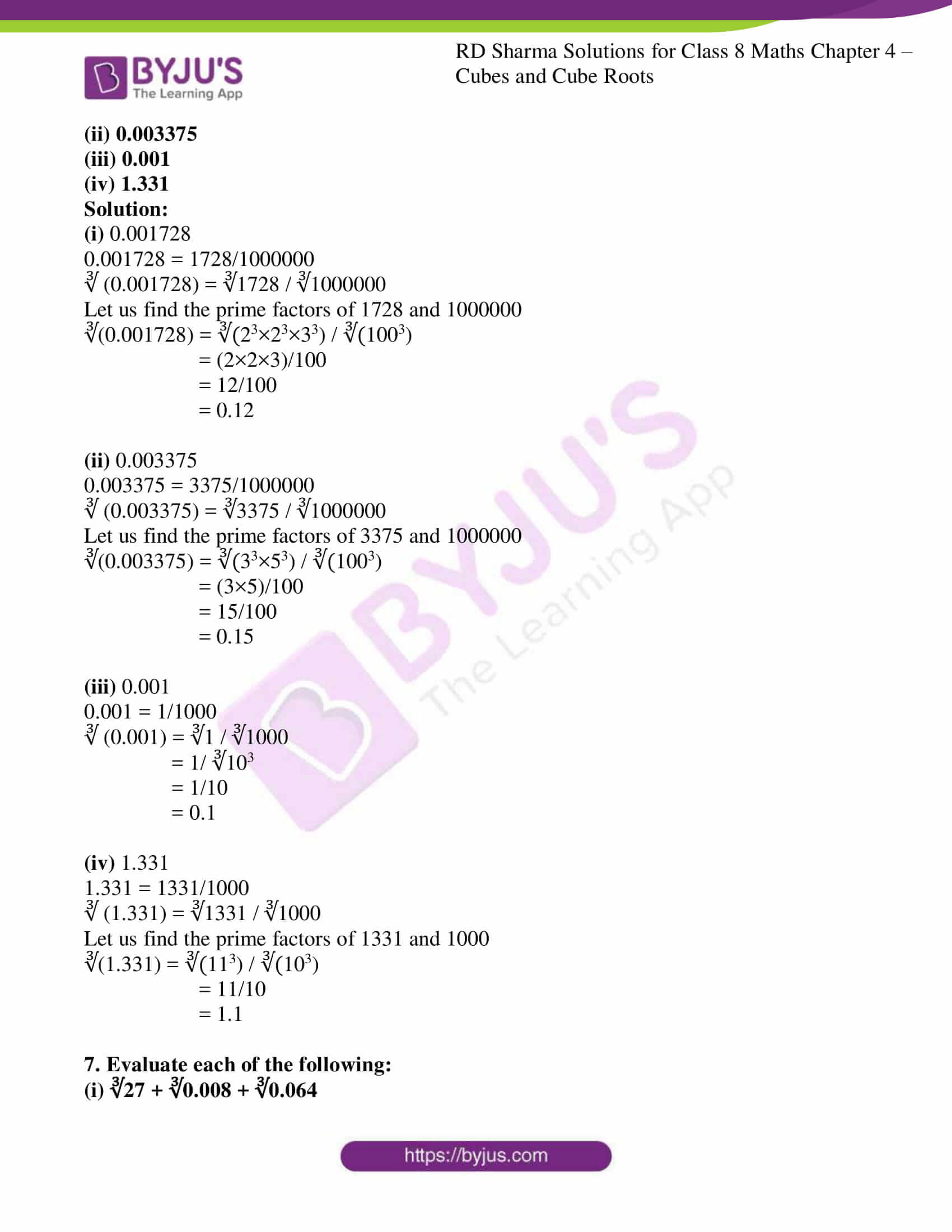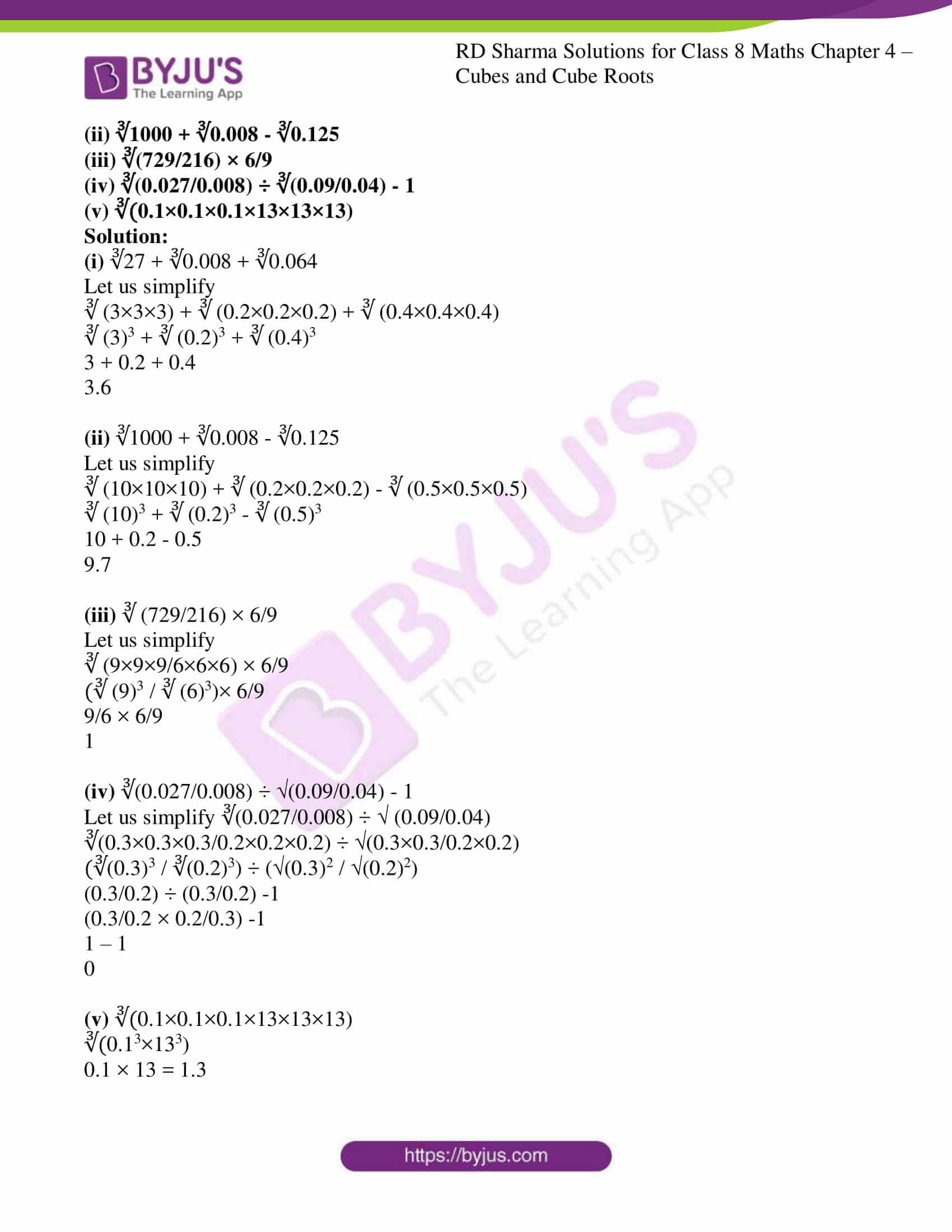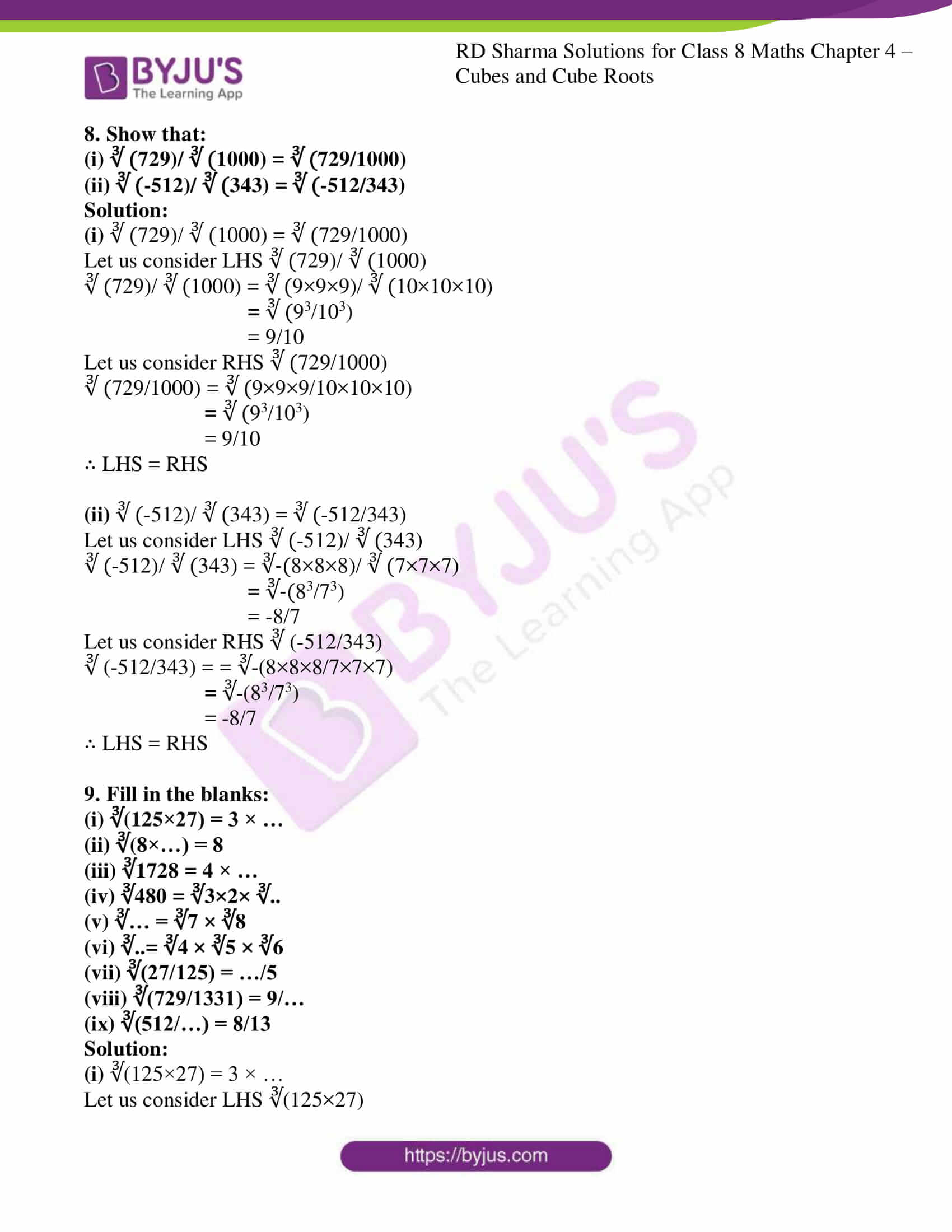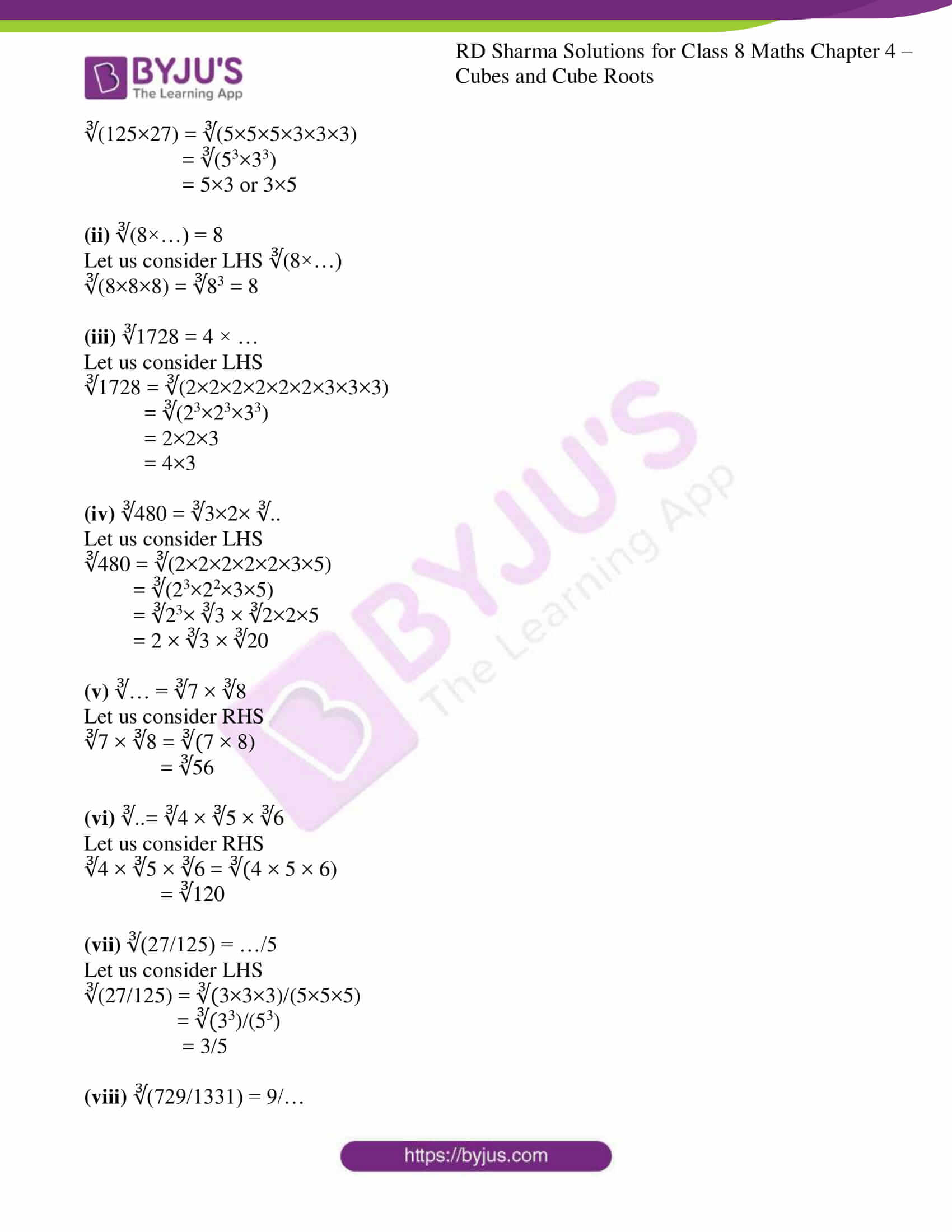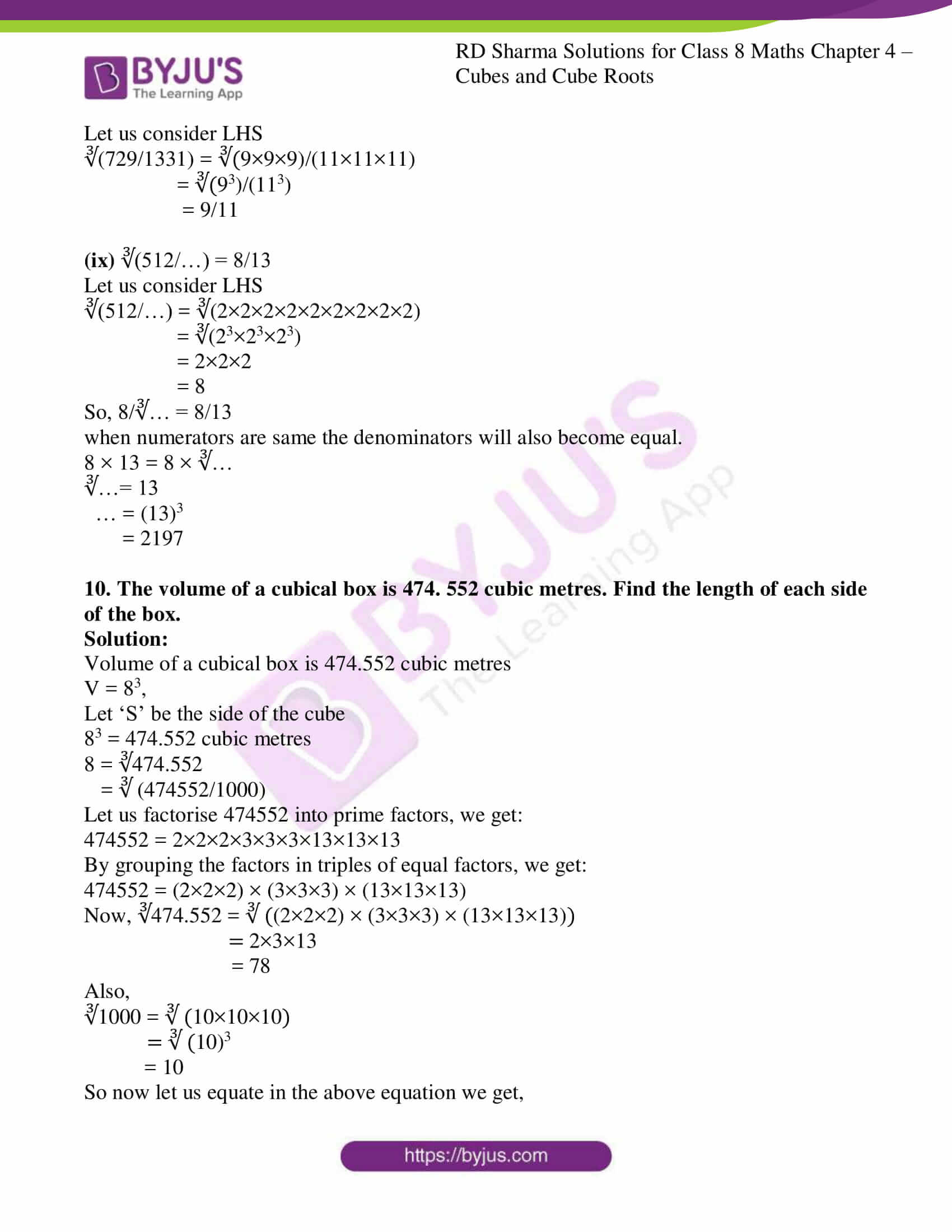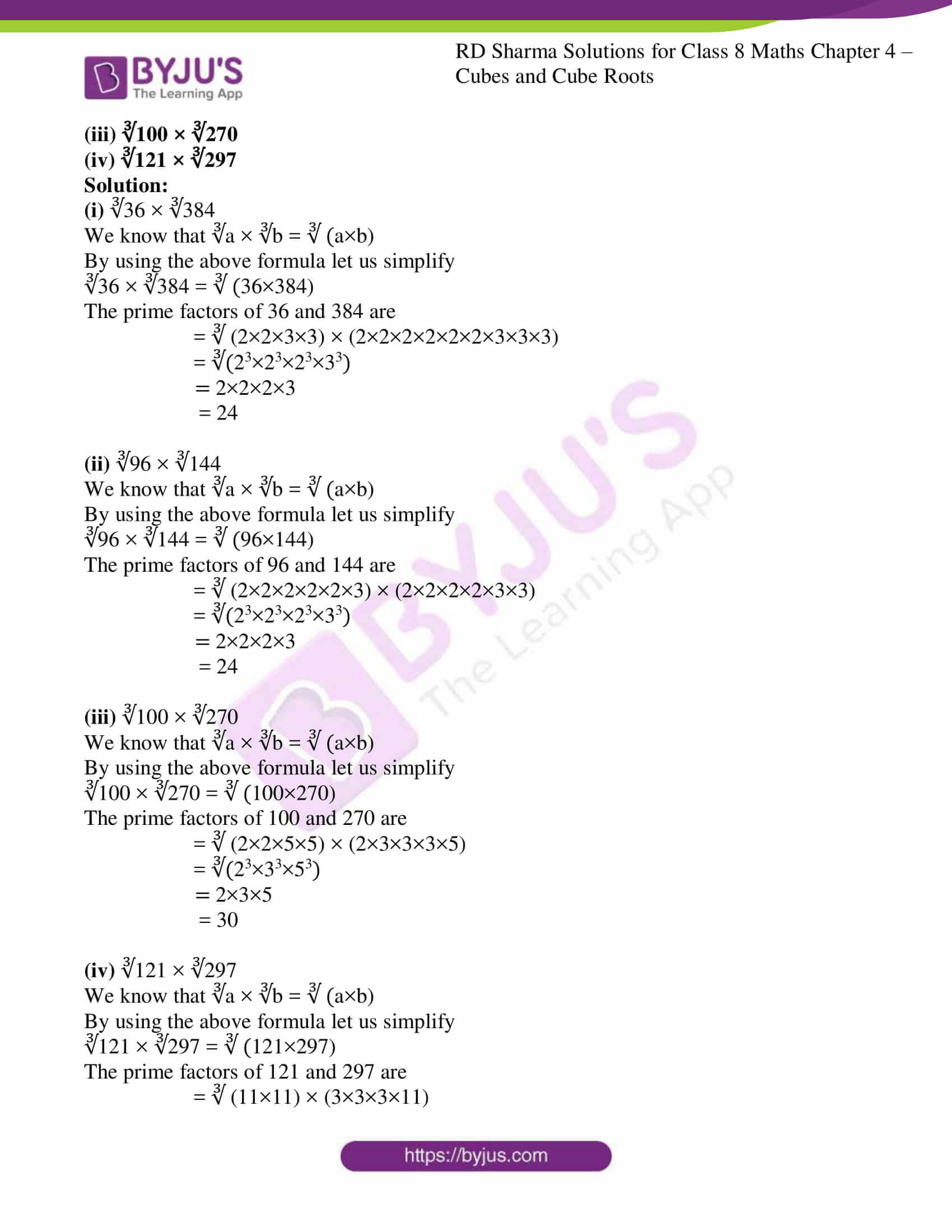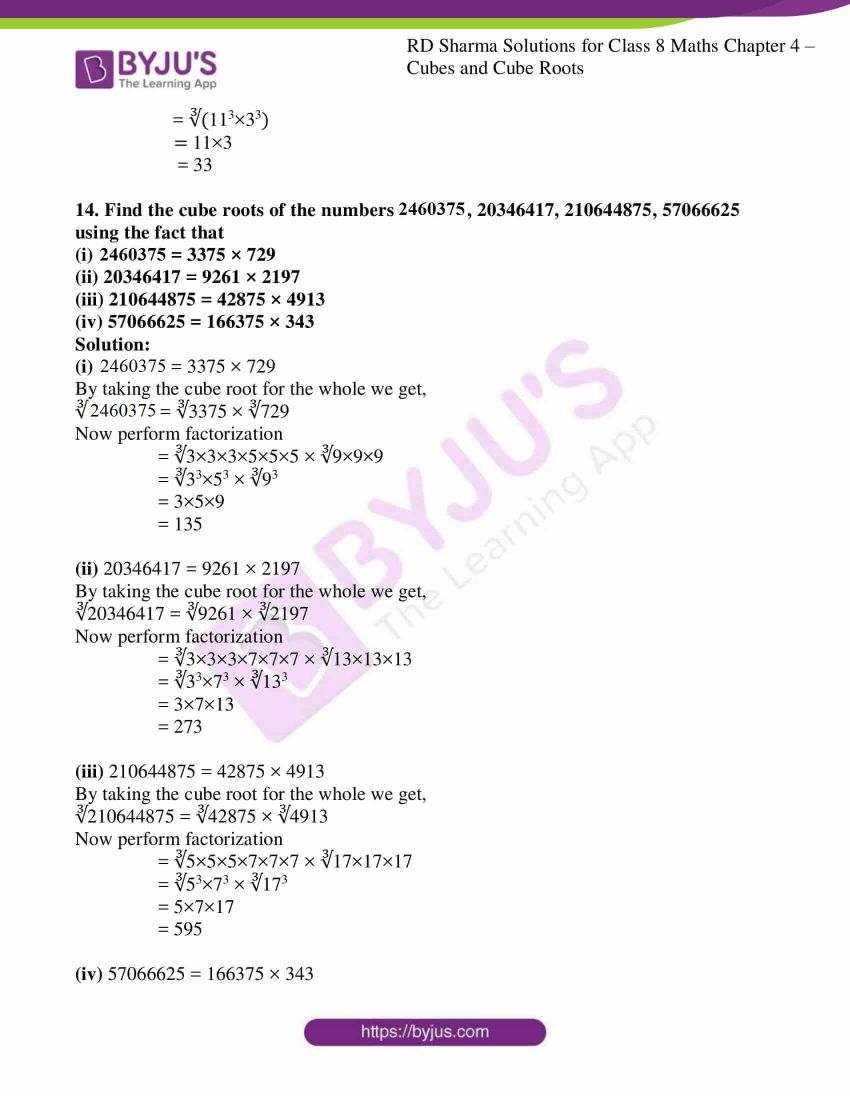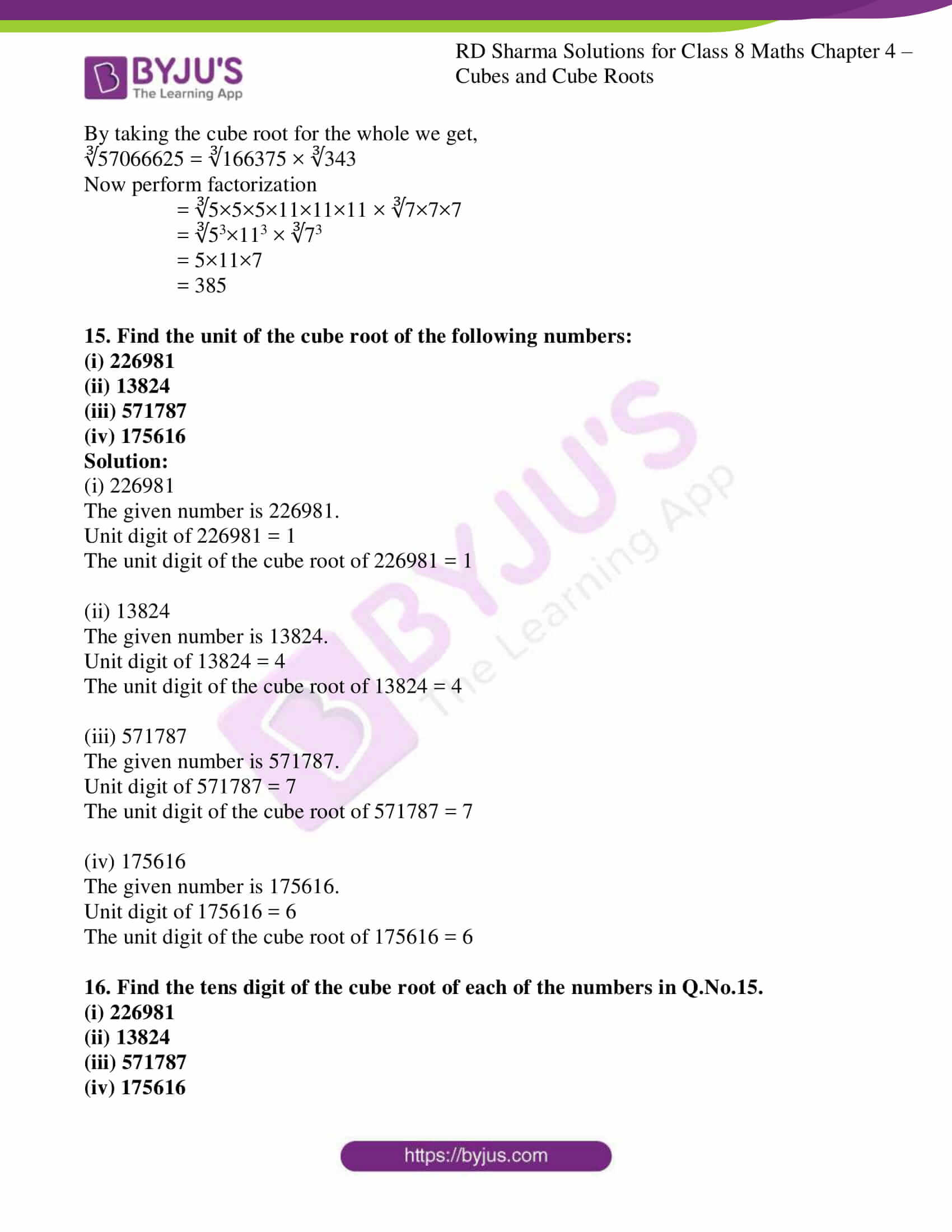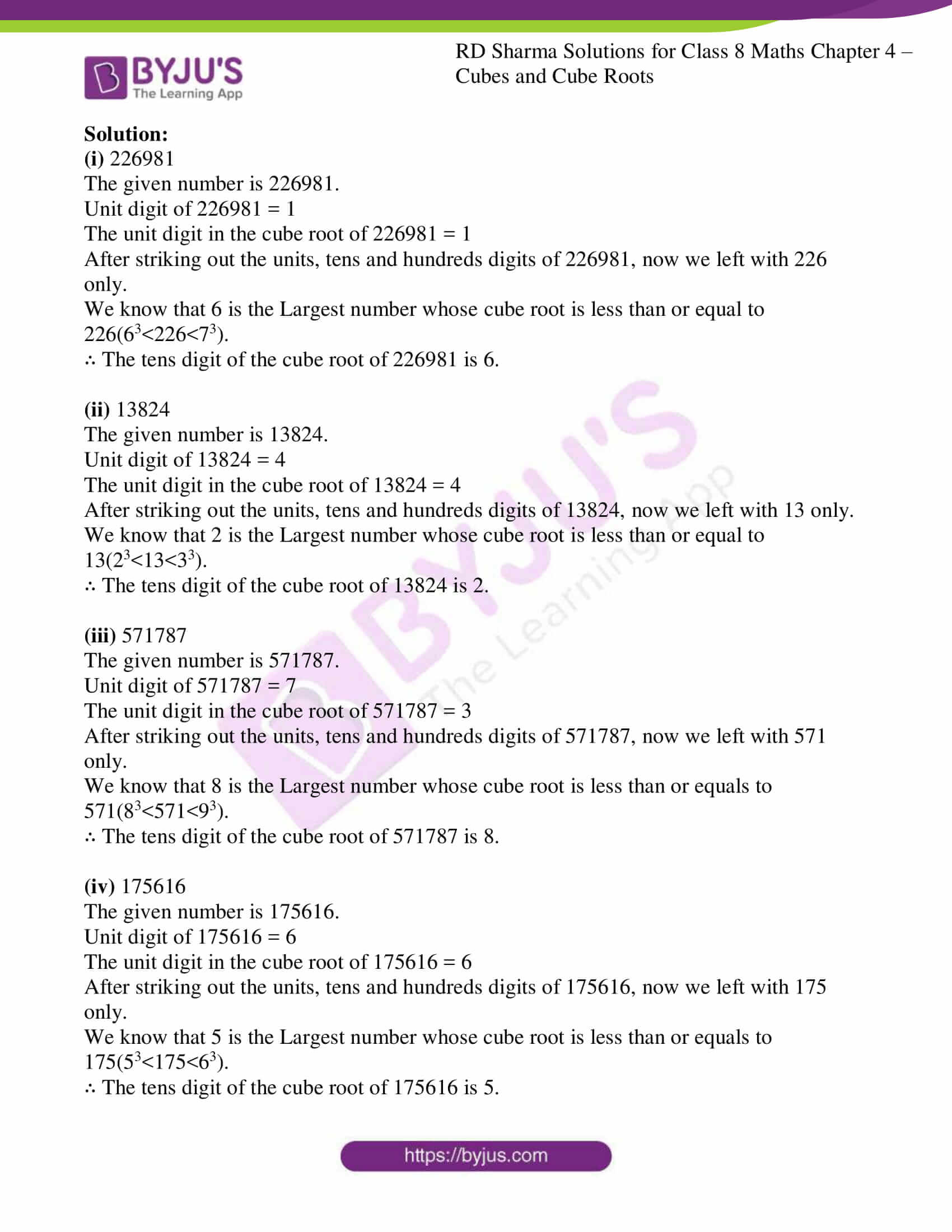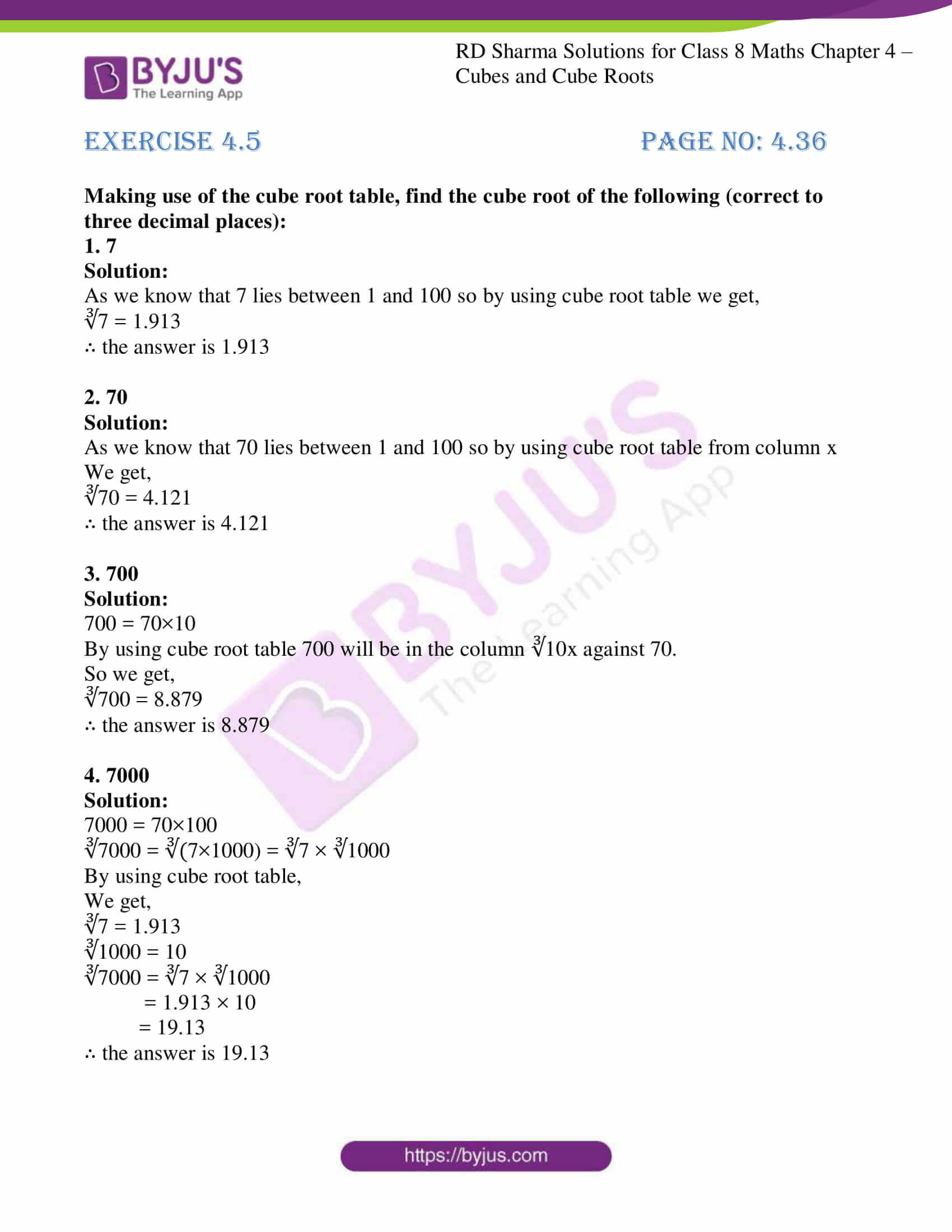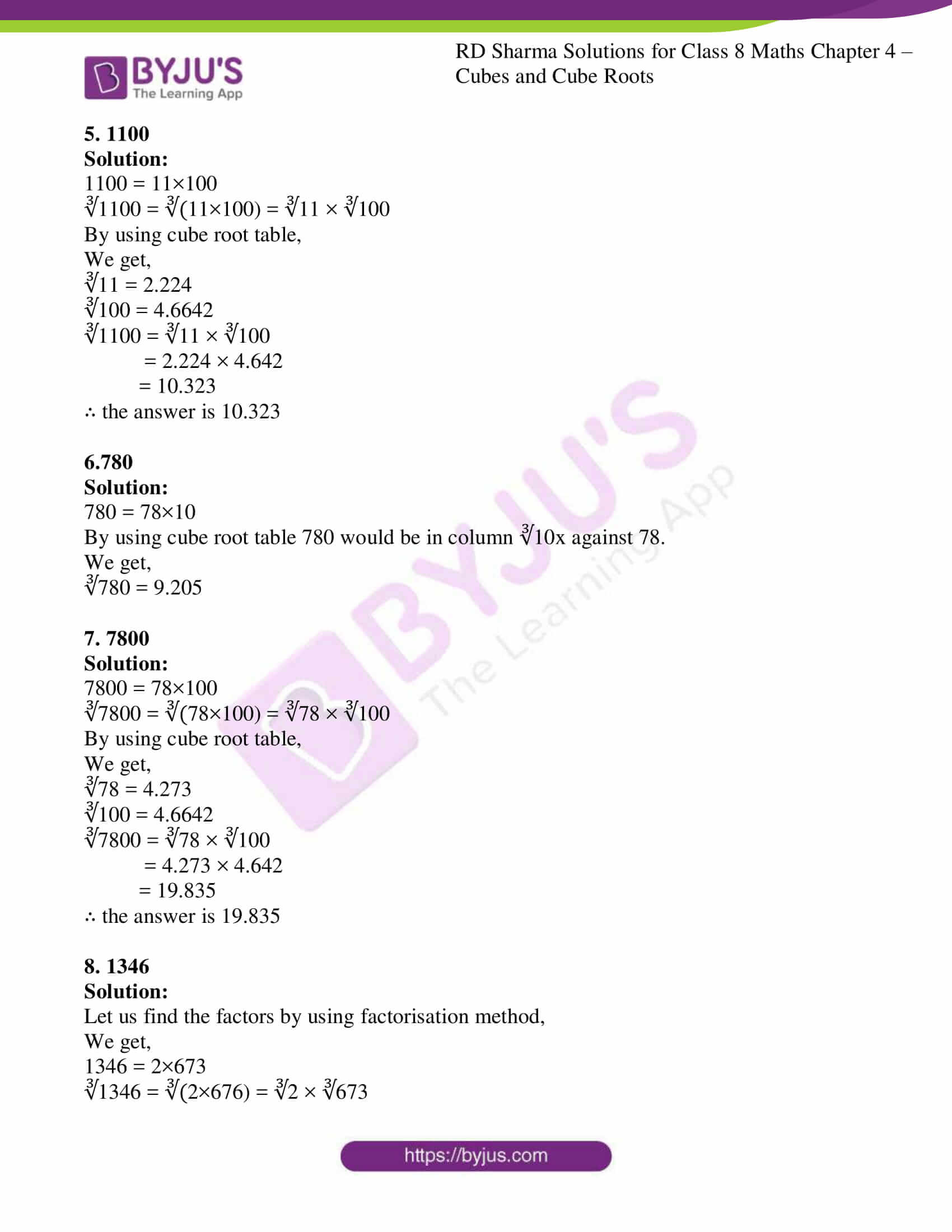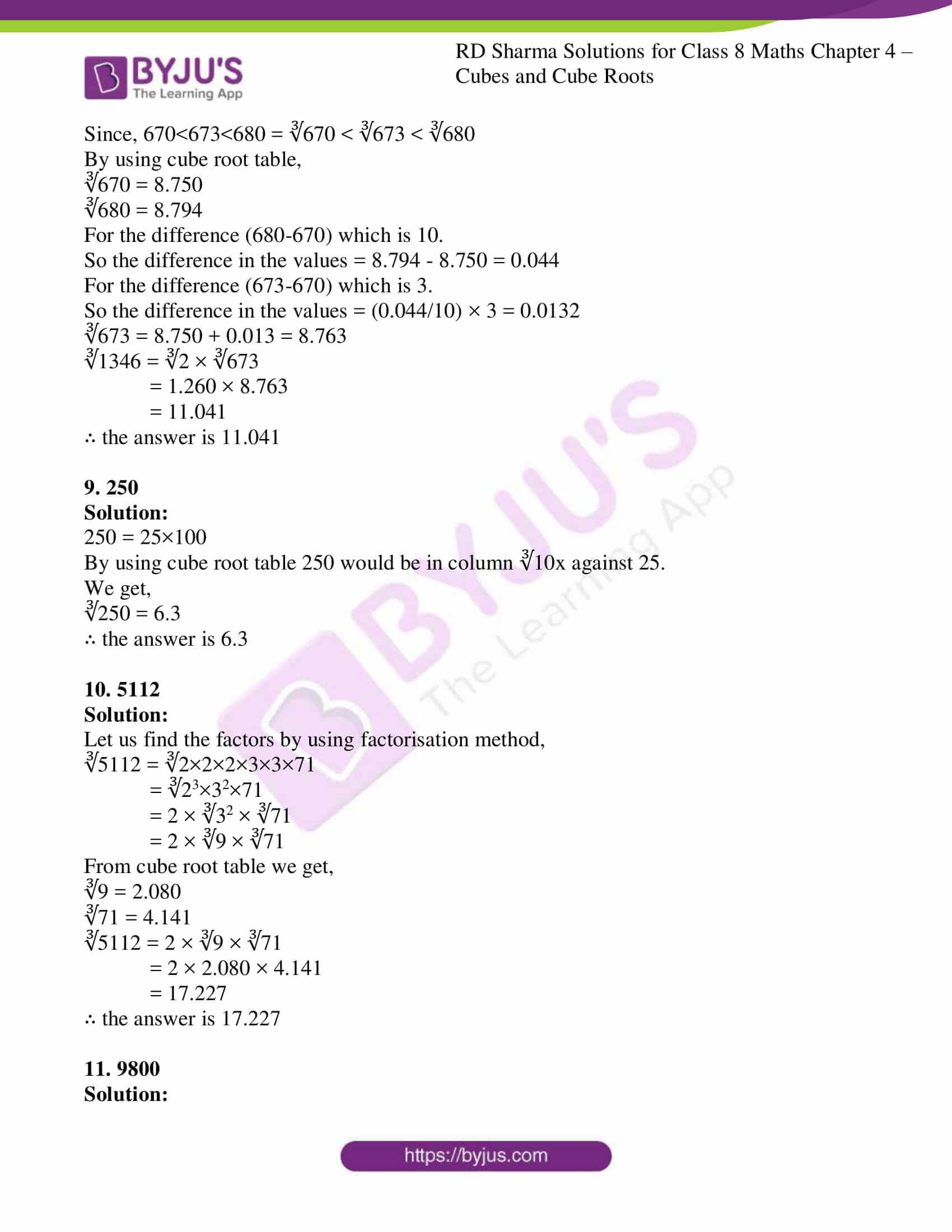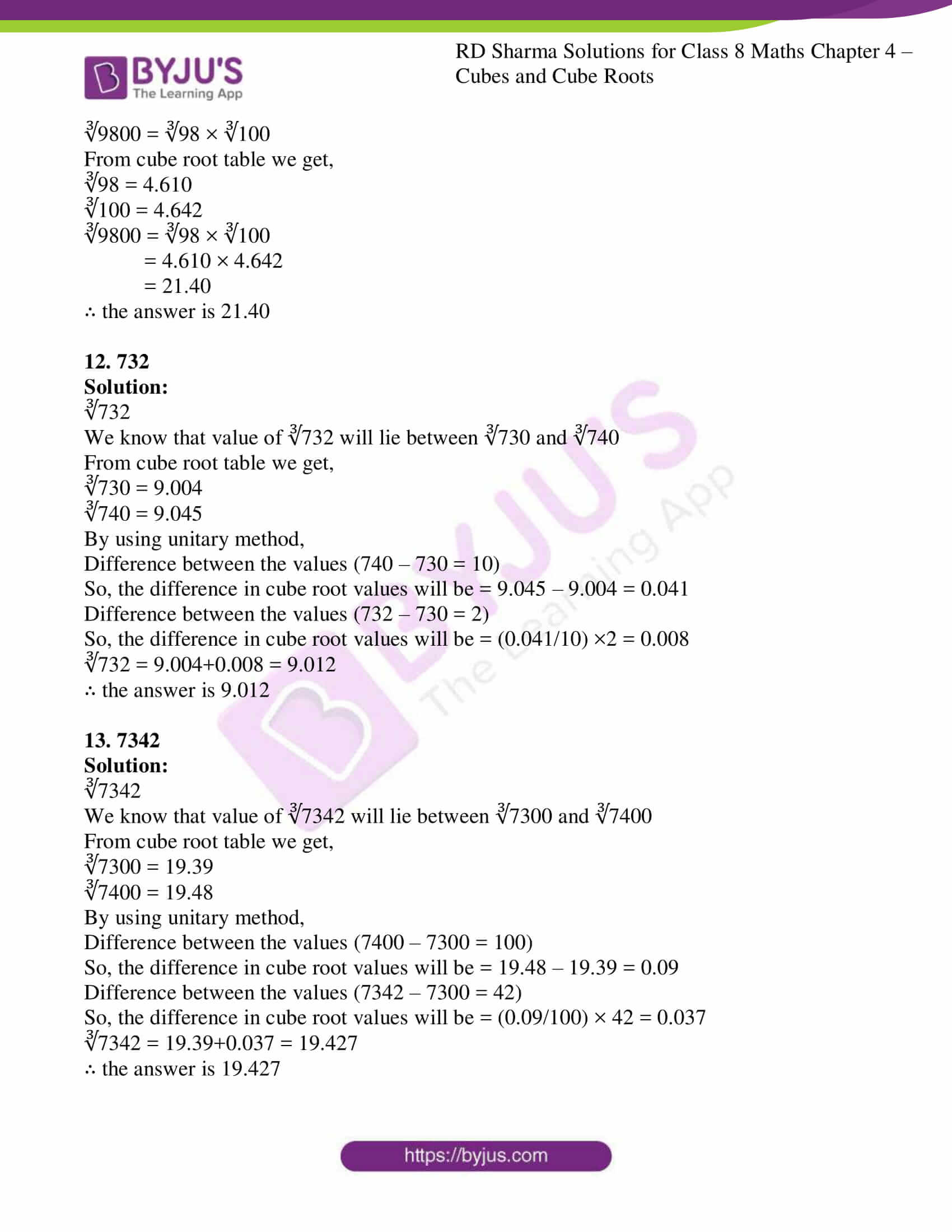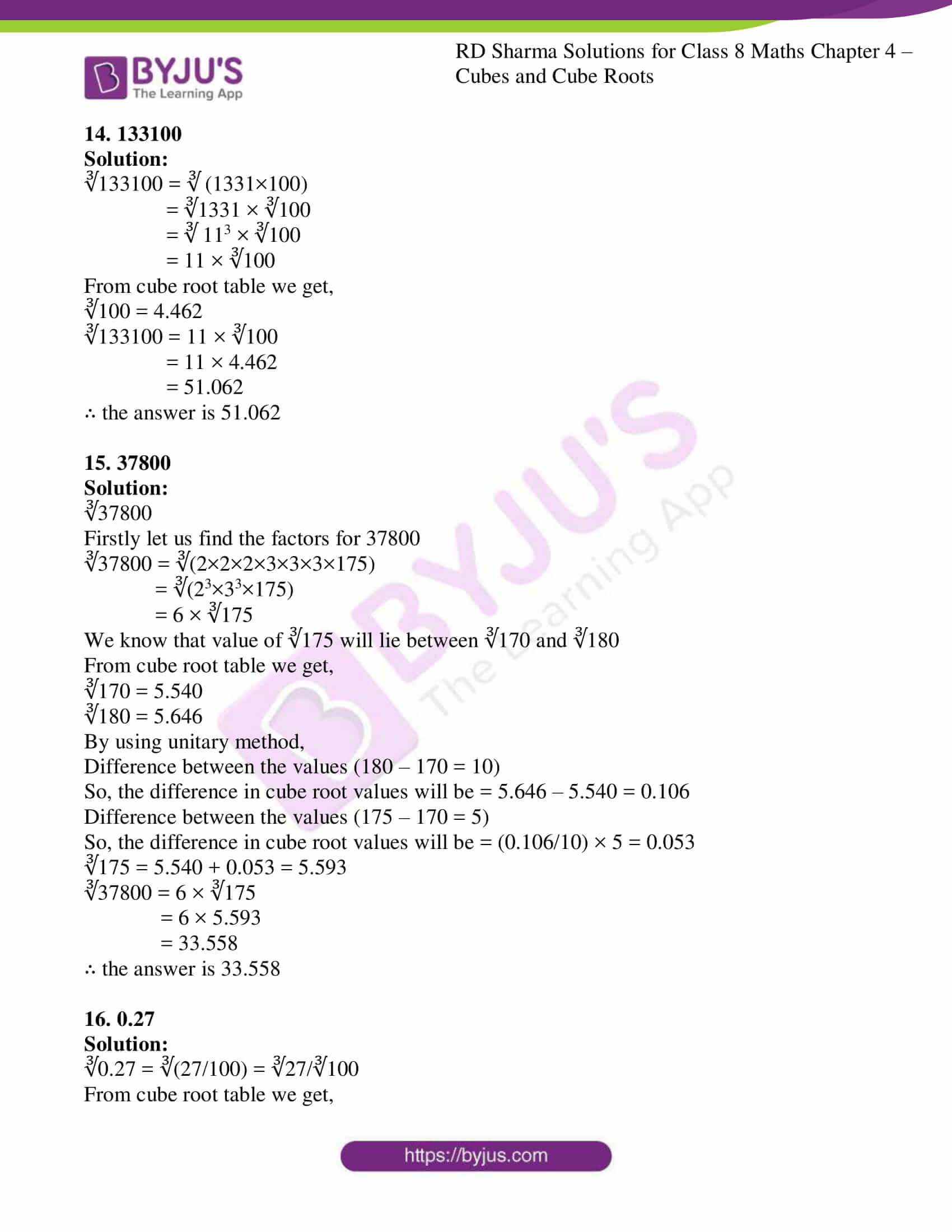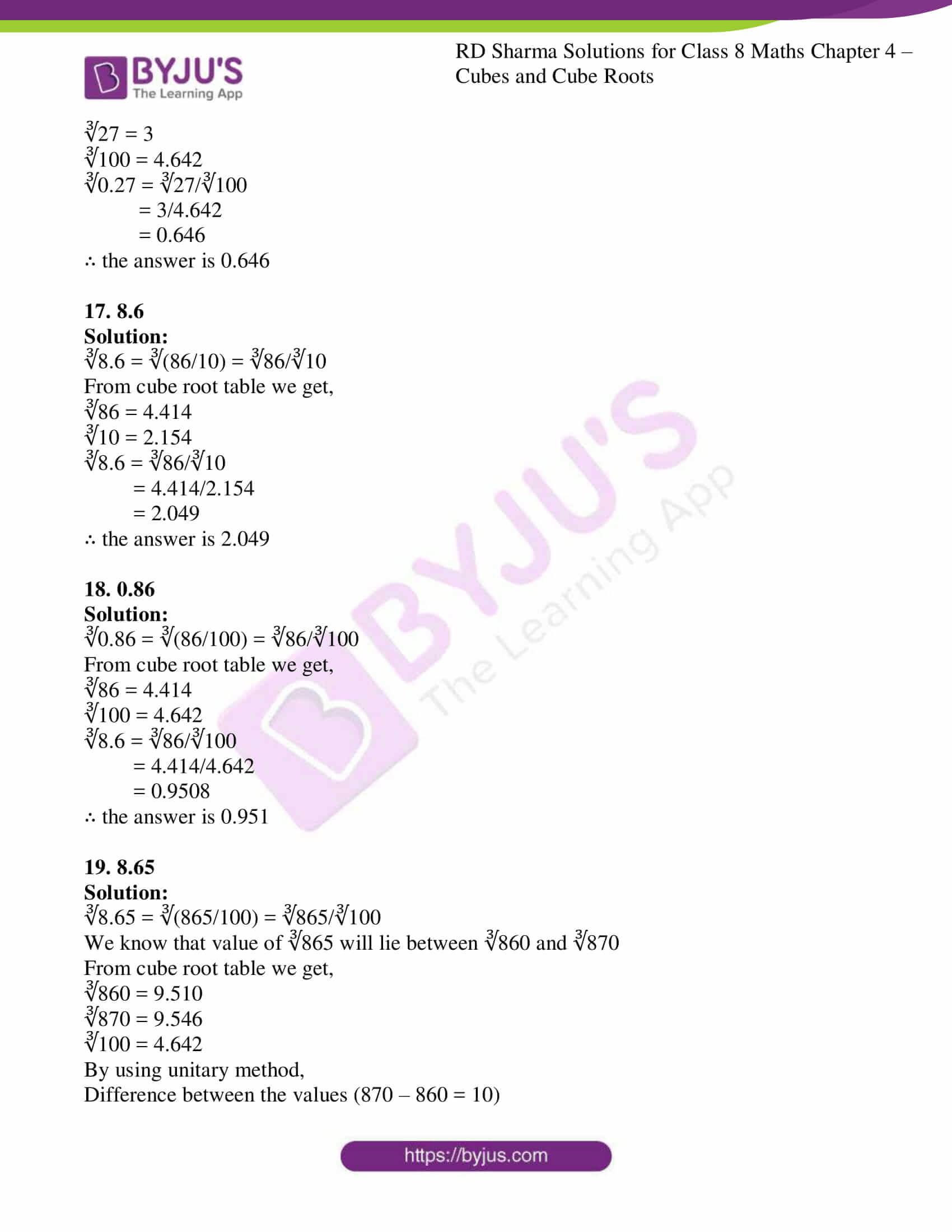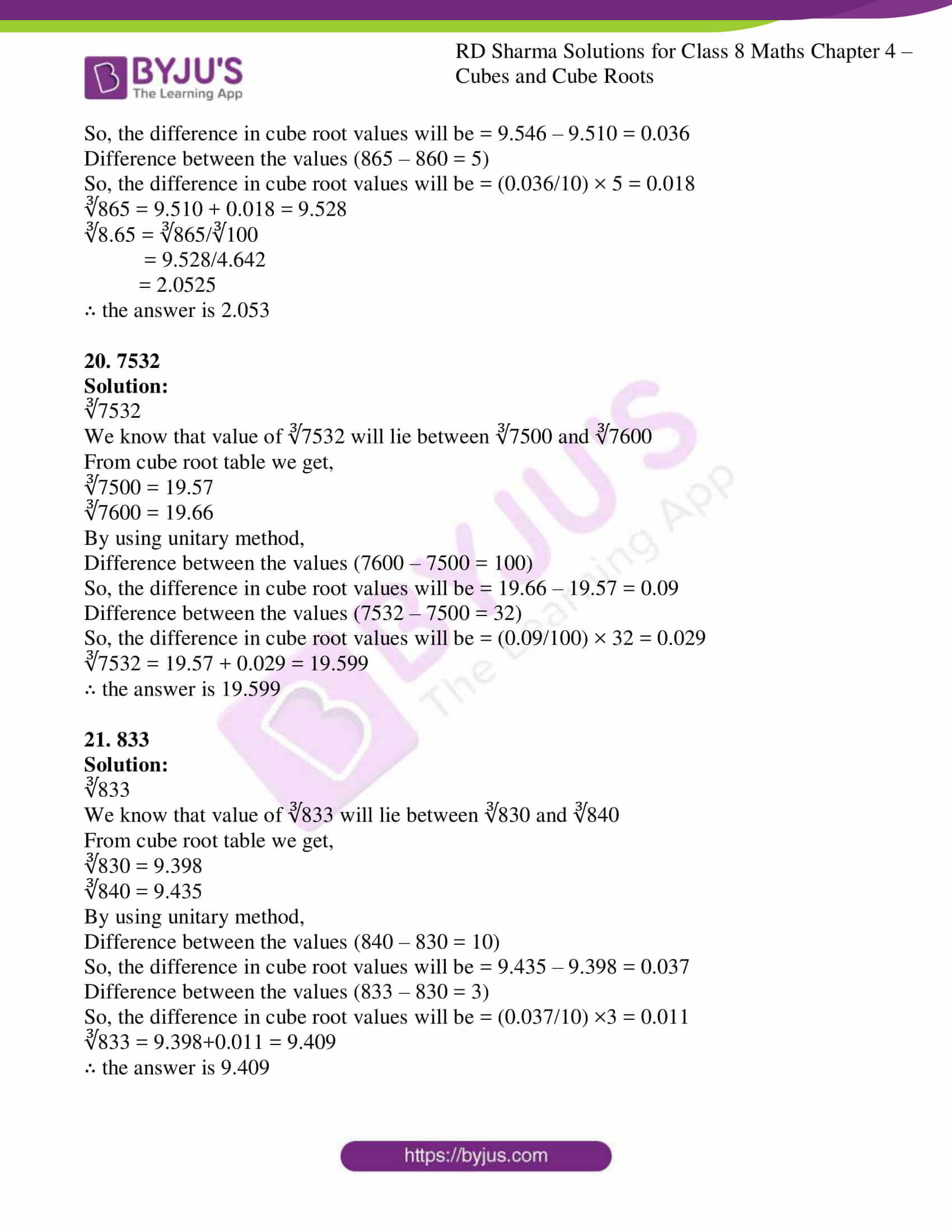## EXERCISE 4.1 PAGE NO: 4.7

1. Find the cubes of the following numbers:
(i) 7 (ii) 12

(iii) 16 (iv) 21

(v) 40 (vi) 55

(vii) 100 (viii) 302

(ix) 301

Solution:

(i) 7

Cube of 7 is

7 = 7× 7 × 7 = 343

(ii) 12

Cube of 12 is

12 = 12× 12× 12 = 1728

(iii) 16

Cube of 16 is

16 = 16× 16× 16 = 4096

(iv) 21

Cube of 21 is

21 = 21 × 21 × 21 = 9261

(v) 40

Cube of 40 is

40 = 40× 40× 40 = 64000

(vi) 55

Cube of 55 is

55 = 55× 55× 55 = 166375

(vii) 100

Cube of 100 is

100 = 100× 100× 100 = 1000000

(viii) 302

Cube of 302 is

302 = 302× 302× 302 = 27543608

(ix) 301

Cube of 301 is

301 = 301× 301× 301 = 27270901

2.Write the cubes of all natural numbers between 1 and 10 and verify the following statements:
(i) Cubes of all odd natural numbers are odd.
(ii) Cubes of all even natural numbers are even.

Solutions:

Firstly let us find the Cube of natural numbers up to 10

13 = 1 × 1 × 1 = 1

23 = 2 × 2 × 2 = 8

33 = 3 × 3 × 3 = 27

43 = 4 × 4 × 4 = 64

53 = 5 × 5 × 5 = 125

63 = 6 × 6 × 6 = 216

73 = 7 × 7 × 7 = 343

83 = 8 × 8 × 8 = 512

93 = 9 × 9 × 9 = 729

103 = 10 × 10 × 10 = 1000

∴ From the above results we can say that

(i) Cubes of all odd natural numbers are odd.

(ii) Cubes of all even natural numbers are even.

3. Observe the following pattern:
13 = 1

13 + 23 = (1+2)2

13 + 23 + 33 = (1+2+3)2
Write the next three rows and calculate the value of 13 + 23 + 33 +…+ 93 by the above pattern.

Solution:

According to given pattern,

13 + 23 + 33 +…+ 93

13 + 23 + 33 +…+ n3 = (1+2+3+…+n) 2

So when n = 10

13 + 23 + 33 +…+ 93 + 103 = (1+2+3+…+10) 2

= (55)2 = 55×55 = 3025

4. Write the cubes of 5 natural numbers which are multiples of 3 and verify the followings:
“The cube of a natural number which is a multiple of 3 is a multiple of 27’

Solution:

We know that the first 5 natural numbers which are multiple of 3 are 3, 6, 9, 12 and 15

So now, let us find the cube of 3, 6, 9, 12 and 15

33 = 3 × 3 × 3 = 27

63 = 6 × 6 × 6 = 216

93 = 9 × 9 × 9 = 729

123 = 12 × 12 × 12 = 1728

153 = 15 × 15 × 15 = 3375

We found that all the cubes are divisible by 27

∴ “The cube of a natural number which is a multiple of 3 is a multiple of 27’

5.Write the cubes of 5 natural numbers which are of the form 3n + 1 (e.g. 4, 7, 10, …) and verify the following:
“The cube of a natural number of the form 3n+1 is a natural number of the same from i.e. when divided by 3 it leaves the remainder 1’

Solution:

We know that the first 5 natural numbers in the form of (3n + 1) are 4, 7, 10, 13 and 16

So now, let us find the cube of 4, 7, 10, 13 and 16

43 = 4 × 4 × 4 = 64

73 = 7 × 7 × 7 = 343

103 = 10 × 10 × 10 = 1000

133 = 13 × 13 × 13 = 2197

163 = 16 × 16 × 16 = 4096

We found that all these cubeswhen divided by ‘3’ leaves remainder 1.

∴ the statement “The cube of a natural number of the form 3n+1 is a natural number of the same from i.e. when divided by 3 it leaves the remainder 1’ is true.

6. Write the cubes 5 natural numbers of the from 3n+2(i.e.5,8,11….) and verify the following:
“The cube of a natural number of the form 3n+2 is a natural number of the same form i.e. when it is dividend by 3 the remainder is 2’

Solution:

We know that the first 5 natural numbers in the form (3n + 2) are 5, 8, 11, 14 and 17

So now, let us find the cubes of 5, 8, 11, 14 and 17

53 = 5 × 5 × 5 = 125

83 = 8 × 8 × 8 = 512

113 = 11 × 11 × 11 = 1331

143 = 14 × 14 × 14 = 2744

173 = 17 × 17 × 17 = 4913

We found that all these cubes when divided by ‘3’ leaves remainder 2.

∴ thestatement“The cube of a natural number of the form 3n+2 is a natural number of the same form i.e. when it is dividend by 3 the remainder is 2’ is true.

7.Write the cubes of 5 natural numbers of which are multiples of 7 and verity the following:
“The cube of a multiple of 7 is a multiple of 73.

Solution:

The first 5 natural numbers which are multiple of 7 are 7, 14, 21, 28 and 35

So, the Cube of 7, 14, 21, 28 and 35

73 = 7 × 7 × 7 = 343

143 = 14 × 14 × 14 = 2744

213 = 21× 21× 21 = 9261

283 = 28 × 28 × 28 = 21952

353 = 35 × 35 × 35 = 42875

We found that all these cubes are multiples of 73(343) as well.

∴The statement“The cube of a multiple of 7 is a multiple of 73 is true.

8. Which of the following are perfect cubes?
(i) 64 (ii) 216
(iii) 243 (iv) 1000
(v) 1728 (vi) 3087
(vii) 4608 (viii) 106480
(ix) 166375 (x) 456533

Solution:

(i) 64

First find the factors of 64

64 = 2 × 2 × 2 × 2 × 2 × 2 = 26 = (22)3 = 43

Hence, it’s a perfect cube.

(ii) 216

First find thefactors of 216

216 = 2 × 2 × 2 × 3 × 3 × 3 = 23 × 33 = 63

Hence, it’s a perfect cube.

(iii) 243

First find thefactors of 243

243 = 3 × 3 × 3 × 3 × 3 = 35 = 33 × 32

Hence, it’s not a perfect cube.

(iv) 1000

First find thefactors of 1000

1000 = 2 × 2 × 2 × 5 × 5 × 5 = 23 × 53 = 103

Hence, it’s a perfect cube.

(v) 1728

First find thefactors of 1728

1728 = 2 × 2 × 2 × 2 × 2 × 2 × 3 × 3 × 3 = 26 × 33 = (4 × 3 )3 = 123

Hence, it’s a perfect cube.

(vi) 3087

First find thefactors of 3087

3087 = 3 × 3 × 7 × 7 × 7 = 32 × 73

Hence, it’s not a perfect cube.

(vii) 4608

First find thefactors of 4608

4608 = 2 × 2 × 3 × 113

Hence, it’s not a perfect cube.

(viii) 106480

First find thefactors of 106480

106480 = 2 × 2 × 2 × 2 × 5 × 11 × 11 × 11

Hence, it’s not a perfect cube.

(ix) 166375

First find thefactors of 166375

166375= 5 × 5 × 5 × 11 × 11 × 11 = 53 × 113 = 553

Hence, it’s a perfect cube.

(x) 456533

First find thefactors of 456533

456533= 11 × 11 × 11 × 7 × 7 × 7 = 113 × 73 = 773

Hence, it’s a perfect cube.

9. Which of the following are cubes of even natural numbers?
216, 512, 729, 1000, 3375, 13824

Solution:

(i) 216 = 23 × 33 = 63

It’s a cube of even natural number.

(ii) 512 = 29 = (23)3 = 83

It’s a cube of even natural number.

(iii) 729 = 33 × 33 = 93

It’s not a cube of even natural number.

(iv) 1000 = 103

It’s a cube of even natural number.

(v) 3375 = 33 × 53 = 153

It’s not a cube of even natural number.

(vi) 13824 = 29 × 33 = (23)3 × 33 = 83×33 = 243

It’s a cube of even natural number.

10. Which of the following are cubes of odd natural numbers?
125, 343, 1728, 4096, 32768, 6859

Solution:

(i) 125 = 5 × 5 × 5 × 5 = 53

It’s a cube of odd natural number.

(ii) 343 = 7 × 7 × 7 = 73

It’s a cube of odd natural number.

(iii) 1728 = 26 × 33 = 43 × 33 = 123

It’s not a cube of odd natural number. As 12 is even number.

(iv) 4096 = 212 = (26)2 = 642

Its not a cube of odd natural number. As 64 is an even number.

(v) 32768 = 215 = (25)3 = 323

It’s not a cube of odd natural number. As 32 is an even number.

(vi) 6859 = 19 × 19 × 19 = 193

It’s a cube of odd natural number.

11. What is the smallest number by which the following numbers must be multiplied, so that the products are perfect cubes?
(i) 675 (ii) 1323
(iii) 2560 (iv) 7803
(v) 107811 (vi) 35721

Solution:

(i) 675

First find the factors of 675

675 = 3 × 3 × 3 × 5 × 5

= 33 × 52

∴To make a perfect cube we need to multiply the product by 5.

(ii) 1323

First find the factors of 1323

1323 = 3 × 3 × 3 × 7 × 7

= 33 × 72

∴To make a perfect cube we need to multiply the product by 7.

(iii) 2560

First find the factors of 2560

2560 = 2 × 2 × 2 × 2 × 2 × 2 × 2 × 2 × 2 × 5

= 23 × 23 × 23 × 5

∴To make a perfect cube we need to multiply the product by 5 × 5 = 25.

(iv) 7803

First find the factors of 7803

7803 = 3 × 3 × 3 × 17 × 17

= 33 × 172

∴To make a perfect cube we need to multiply the product by 17.

(v) 107811

First find the factors of 107811

107811 = 3 × 3 × 3 × 3 × 11 × 11 × 11

= 33 × 3 × 113

∴To make a perfect cube we need to multiply the product by 3 × 3 = 9.

(vi) 35721

First find the factors of 35721

35721 = 3 × 3 × 3 × 3 × 3 × 3 × 7 × 7

= 33 × 33 × 72

∴To make a perfect cube we need to multiply the product by 7.

12. By which smallest number must the following numbers be divided so that the quotient is a perfect cube?
(i) 675 (ii) 8640
(iii) 1600 (iv) 8788
(v) 7803 (vi) 107811
(vii) 35721 (viii) 243000

Solution:

(i) 675

First find the prime factors of 675

675 = 3 × 3 × 3 × 5 × 5

= 33 × 52

Since 675 is not a perfect cube.

To make the quotient a perfect cube we divide it by 52 = 25, which gives 27 as quotient where, 27 is a perfect cube.

∴ 25 is the required smallest number.

(ii) 8640

First find the prime factors of 8640

8640 = 2 × 2 × 2 × 2 × 2 × 2 × 3 × 3 × 3 × 5

= 23 × 23 × 33 × 5

Since 8640 is not a perfect cube.

To make the quotient a perfect cube we divide it by 5, which gives 1728 as quotient and we know that 1728 is a perfect cube.

∴5 is the required smallest number.

(iii) 1600

First find the prime factors of 1600

1600 = 2 × 2 × 2 × 2 × 2 × 2 × 5 × 5

= 23 × 23 × 52

Since 1600 is not a perfect cube.

To make the quotient a perfect cube we divide it by 52 = 25, which gives 64 as quotient and we know that 64 is a perfect cube

∴ 25 is the required smallest number.

(iv) 8788

First find the prime factors of 8788

8788 = 2 × 2 × 13 × 13 × 13

= 22 × 133

Since 8788 is not a perfect cube.

To make the quotient a perfect cube we divide it by 4, which gives 2197 as quotient and we know that 2197 is a perfect cube

∴ 4 is the required smallest number.

(v) 7803

First find the prime factors of 7803

7803 = 3 × 3 × 3 × 17 × 17

= 33 × 172

Since 7803 is not a perfect cube.

To make the quotient a perfect cube we divide it by 172 = 289, which gives 27 as quotient and we know that 27 is a perfect cube

∴ 289 is the required smallest number.

(vi) 107811

First find the prime factors of 107811

107811 = 3 × 3 × 3 × 3 × 11 × 11 × 11

= 33 × 113 × 3

Since 107811 is not a perfect cube.

To make the quotient a perfect cube we divide it by 3, which gives 35937 as quotient and we know that 35937 is a perfect cube.

∴ 3 is the required smallest number.

(vii) 35721

First find the prime factors of 35721

35721 = 3 × 3 × 3 × 3 × 3 × 3 × 7 × 7

= 33 × 33 × 72

Since 35721 is not a perfect cube.

To make the quotient a perfect cube we divide it by 72 = 49, which gives 729 as quotient and we know that 729 is a perfect cube

∴ 49 is the required smallest number.

(viii) 243000

First find the prime factors of 243000

243000 = 2 × 2 × 2 × 3 × 3 × 3 × 3 × 3 × 5 × 5 × 5

= 23 × 33 × 53 × 32

Since 243000 is not a perfect cube.

To make the quotient a perfect cube we divide it by 32 = 9, which gives 27000 as quotient and we know that 27000 is a perfect cube

∴ 9 is the required smallest number.

13. Prove that if a number is trebled then its cube is 27 time the cube of the given number.

Solution:

Let us consider a number as a

So the cube of the assumed number is = a3

Now, the number is trebled = 3 × a = 3a

So the cube of new number = (3a) 3 = 27a3

∴New cube is 27 times of the original cube.

Hence, proved.

14. What happens to the cube of a number if the number is multiplied by
(i) 3?
(ii) 4?
(iii) 5?

Solution:

(i) 3?

Let us consider the number as a

So its cube will be = a3

According to the question, the number is multiplied by 3

New number becomes = 3a

So the cube of new number will be = (3a) 3 = 27a3

Hence, number will become 27 times the cube of the number.

(ii) 4?

Let us consider the number as a

So its cube will be = a3

According to the question, the number is multiplied by 4

New number becomes = 4a

So the cube of new number will be = (4a) 3 = 64a3

Hence, number will become 64 times the cube of the number.

(iii) 5?

Let us consider the number as a

So its cube will be = a3

According to the question, the number is multiplied by 5

New number becomes = 5a

So the cube of new number will be = (5a) 3 = 125a3

Hence, number will become 125 times the cube of the number.

15. Find the volume of a cube, one face of which has an area of 64m2.

Solution:

We know that the given area of one face of cube = 64 m2

Let the length of edge of cube be ‘a’ metre

a2 = 64

a = √ 64

= 8m

Now, volume of cube = a3

a3 = 83 = 8 × 8 × 8

= 512m3

∴Volume of a cube is 512m3

16. Find the volume of a cube whose surface area is 384m2.

Solution:

We know that the surface area of cube = 384 m2

Let us consider the length of each edge of cube be ‘a’ meter

6a2 = 384

a2 = 384/6

= 64

a = √64

= 8m

Now, volume of cube = a3

a3 = 83 = 8 × 8 × 8

= 512m3

∴ Volume of a cube is 512m3

17. Evaluate the following:
(i) {(52 + 122)1/2}3
(ii) {(62 + 82)1/2}3

Solution:

(i) {(52 + 122)1/2}3

When simplified above equation we get,

{(25 + 144)1/2}3

{(169)1/2}3

{(132)1/2}3

(13)3

2197

(ii) {(62 + 82)1/2}3

When simplified above equation we get,

{(36 + 64)1/2}3

{(100)1/2}3

{(102)1/2}3

(10)3

1000

18. Write the units digit of the cube of each of the following numbers:
31, 109, 388, 4276, 5922, 77774, 44447, 125125125

Solution:

31

To find unit digit of cube of a number we perform the cube of unit digit only.

Unit digit of 31 is 1

Cube of 1 = 13 = 1

∴ Unit digit of cube of 31 is always 1

109

To find unit digit of cube of a number we perform the cube of unit digit only.

Unit digit of 109 is = 9

Cube of 9 = 93 = 729

∴ Unit digit of cube of 109 is always 9

388

To find unit digit of cube of a number we perform the cube of unit digit only.

Unit digit of 388 is = 8

Cube of 8 = 83 = 512

∴ Unit digit of cube of 388 is always 2

4276

To find unit digit of cube of a number we perform the cube of unit digit only.

Unit digit of 4276 is = 6

Cube of 6 = 63 = 216

∴ Unit digit of cube of 4276 is always 6

5922

To find unit digit of cube of a number we perform the cube of unit digit only.

Unit digit of 5922 is = 2

Cube of 2 = 23 = 8

∴ Unit digit of cube of 5922 is always 8

77774

To find unit digit of cube of a number we perform the cube of unit digit only.

Unit digit of 77774 is = 4

Cube of 4 = 43 = 64

∴ Unit digit of cube of 77774 is always 4

44447

To find unit digit of cube of a number we perform the cube of unit digit only.

Unit digit of 44447 is = 7

Cube of 7 = 73 = 343

∴ Unit digit of cube of 44447 is always 3

125125125

To find unit digit of cube of a number we perform the cube of unit digit only.

Unit digit of 125125125 is = 5

Cube of 5 = 53 = 125

∴ Unit digit of cube of 125125125 is always 5

19. Find the cubes of the following numbers by column method:
(i) 35
(ii) 56
(iii) 72

Solution:

(i) 35

We have, a = 3 and b = 5

 Column I a3 Column II 3×a2×b Column III 3×a×b2 Column IV b3 33 = 27 3×9×5 = 135 3×3×25 = 225 53 = 125 +15 +23 +12 125 42 158 237 42 8 7 5

∴ The cube of 35 is 42875

(ii) 56

We have, a = 5 and b = 6

 Column I a3 Column II 3×a2×b Column III 3×a×b2 Column IV b3 53 = 125 3×25×6 = 450 3×5×36 = 540 63 = 216 +50 +56 +21 126 175 506 561 175 6 1 6

∴ The cube of 56 is 175616

(iii) 72

We have, a = 7 and b = 2

 Column I a3 Column II 3×a2×b Column III 3×a×b2 Column IV b3 73 = 343 3×49×2 = 294 3×7×4 = 84 23 = 8 +30 +8 +0 8 373 302 84 373 2 4 8

∴ The cube of 72 is 373248

20. Which of the following numbers are not perfect cubes?
(i) 64
(ii) 216
(iii) 243
(iv) 1728

Solution:

(i) 64

Firstly let us find the prime factors of 64

64 = 2 × 2 × 2 × 2 × 2 × 2

= 23 × 23

= 43

Hence, it’s a perfect cube.

(ii) 216

Firstly let us find the prime factors of 216

216 = 2 × 2 × 2 × 3 × 3 × 3

= 23 × 33

= 63

Hence, it’s a perfect cube.

(iii) 243

Firstly let us find the prime factors of 243

243 = 3 × 3 × 3 × 3 × 3

= 33 × 32

Hence, it’s not a perfect cube.

(iv) 1728

Firstly let us find the prime factors of 1728

1728 = 2 × 2 × 2 × 2 × 2 × 2 × 3 × 3 × 3

= 23 × 23 × 33

= 123

Hence, it’s a perfect cube.

21. For each of the non-perfect cubes in Q. No 20 find the smallest number by which it must be
(a) Multiplied so that the product is a perfect cube.
(b) Divided so that the quotient is a perfect cube.

Solution:

Only non-perfect cube in previous question was = 243

(a) Multiplied so that the product is a perfect cube.

Firstly let us find the prime factors of 243

243 = 3 × 3 × 3 × 3 × 3 = 33 × 32

Hence, to make it a perfect cube we should multiply it by 3.

(b) Divided so that the quotient is a perfect cube.

Firstly let us find the prime factors of 243

243 = 3 × 3 × 3 × 3 × 3 = 33 × 32

Hence, to make it a perfect cube we have to divide it by 9.

22. By taking three different, values of n verify the truth of the following statements:
(i) If n is even, then n3 is also even.
(ii) If n is odd, then n3 is also odd.
(ii) If n leaves remainder 1 when divided by 3, then n3 also leaves 1 as remainder when divided by 3.
(iv) If a natural number n is of the form 3p+2 then n3 also a number of the same type.

Solution:

(i) If n is even, then n3 is also even.

Let us consider three even natural numbers 2, 4, 6

So now, Cubes of 2, 4 and 6 are

23 = 8

43 = 64

63 = 216

Hence, we can see that all cubes are even in nature.

Statement is verified.

(ii) If n is odd, then n3 is also odd.

Let us consider three odd natural numbers 3, 5, 7

So now, cubes of 3, 5 and 7 are

33 = 27

53 = 125

73 = 343

Hence, we can see that all cubes are odd in nature.

Statement is verified.

(iii) If n leaves remainder 1 when divided by 3, then n3 also leaves 1 as remainder when divided by 3.

Let us consider three natural numbers of the form (3n+1) are 4, 7 and 10

So now, cube of 4, 7, 10 are

43 = 64

73 = 343

103 = 1000

We can see that if we divide these numbers by 3, we get 1 as remainder in each case.

Hence, statement is verified.

(iv) If a natural number n is of the form 3p+2 then n3 also a number of the same type.

Let us consider three natural numbers of the form (3p+2) are 5, 8 and 11

So now, cube of 5, 8 and 10 are

53 = 125

83 = 512

113 = 1331

Now, we try to write these cubes in form of (3p + 2)

125 = 3 × 41 + 2

512 = 3 × 170 + 2

1331 = 3 × 443 + 2

Hence, statement is verified.

23. Write true (T) or false (F) for the following statements:
(i) 392 is a perfect cube.
(ii) 8640 is not a perfect cube.
(iii) No cube can end with exactly two zeros.
(iv) There is no perfect cube which ends in 4.
(v) For an integer a, a3 is always greater than a2.
(vi) If a and b are integers such that a2>b2, then a3>b3.
(vii) If a divides b, then a3 divides b3.
(viii) If a2 ends in 9, then a3 ends in 7.
(ix) If a2 ends in an even number of zeros, then a3 ends in 25.
(x) If a2 ends in an even number of zeros, then a3 ends in an odd number of zeros.

Solution:

(i) 392 is a perfect cube.

Firstly let’s find the prime factors of 392 = 2 × 2 × 2 × 7 × 7 = 23 × 72

Hence the statement is False.

(ii) 8640 is not a perfect cube.

Prime factors of 8640 = 2 × 2 × 2 × 2 × 2 × 2 × 3 × 3 × 3 × 5 = 23 × 23 × 33 × 5

Hence the statement is True

(iii) No cube can end with exactly two zeros.

Statement is True.

Because a perfect cube always have zeros in multiple of 3.

(iv) There is no perfect cube which ends in 4.

We know 64 is a perfect cube = 4 × 4 × 4 and it ends with 4.

Hence the statement is False.

(v) For an integer a, a3 is always greater than a2.

Statement is False.

Because in case of negative integers ,

(-2)2 = 4 and (-2)3 = -8

(vi) If a and b are integers such that a2>b2, then a3>b3.

Statement is False.

In case of negative integers,

(-5)2 > (-4)2 = 25 > 16

But, (-5)3 > (-4)3 = -125 > -64 is not true.

(vii) If a divides b, then a3 divides b3.

Statement is True.

If a divides b

b/a = k, so b=ak

b3/a3 = (ak)3/a3 = a3k3/a3 = k3,

For each value of b and a its true.

(viii) If a2 ends in 9, then a3 ends in 7.

Statement is False.

Let a = 7

72 = 49 and 73 = 343

(ix) If a2 ends in an even number of zeros, then a3 ends in 25.

Statement is False.

Since, when a = 20

a2 = 202 = 400 and a3 = 8000 (a3 doesn’t end with 25)

(x) If a2 ends in an even number of zeros, then a3 ends in an odd number of zeros.

Statement is False.

Since, when a = 100

a2 = 1002 = 10000 and a3 = 1003 = 1000000 (a3 doesn’t end with odd number of zeros)

## EXERCISE 4.2 PAGE NO: 4.13

1. Find the cubes of:
(i) -11
(ii) -12
(iii) -21

Solution:

(i) -11

The cube of 11 is

(-11)3 = -11× -11× -11 = -1331

(ii) -12

The cube of 12 is

(-12)3 = -12× -12× -12 = -1728

(iii) -21

The cube of 21 is

(-21)3 = -21× -21× -21 = -9261

2. Which of the following integers are cubes of negative integers
(i) -64
(ii) -1056
(iii) -2197
(iv) -2744
(v) -42875

Solution:

(i) -64

The prime factors of 64 are

64 = 2 × 2 × 2 × 2 × 2 × 2

= 23 × 23

= 43

∴ 64 is a perfect cube of negative integer – 4.

(ii) -1056

The prime factors of 1056 are

1056 = 2 × 2 × 2 × 2 × 2 × 3 × 11

1056 is not a perfect cube.

∴ -1056 is not a cube of negative integer.

(iii) -2197

The prime factors of 2197 are

2197 = 13 × 13 × 13

= 133

∴ 2197 is a perfect cube of negative integer – 13.

(iv) -2744

The prime factors of 2744 are

2744 = 2 × 2 × 2 × 7 × 7 × 7

= 23 × 73

= 143

2744 is a perfect cube.

∴ -2744 is a cube of negative integer – 14.

(v) -42875

The prime factors of 42875 are

42875 = 5 × 5 × 5 × 7 × 7 × 7

= 53 × 73

= 353

42875 is a perfect cube.

∴ -42875 is a cube of negative integer – 35.

3. Show that the following integers are cubes of negative integers. Also, find the integer whose cube is the given integer.
(i) -5832
(ii) -2744000

Solution:

(i) -5832

The prime factors of 5832 are

5823 = 2 × 2 × 2 × 3 × 3 × 3 × 3 × 3 × 3

= 23 × 33 × 33

= 183

5832 is a perfect cube.

∴ -5832 is a cube of negative integer – 18.

(ii) -2744000

The prime factors of 2744000 are

2744000 = 2 × 2 × 2 × 2 × 2 × 2 × 5 × 5 × 5 × 7 × 7 × 7

= 23 × 23× 53 × 73

2744000 is a perfect cube.

∴ -2744000 is a cube of negative integer – 140.

4. Find the cube of:
(i) 7/9 (ii) -8/11
(iii) 12/7 (iv) -13/8
(v) $$2\frac{2}{5}$$ (vi) $$3\frac{1}{4}$$ (vii) 0.3 (viii) 1.5
(ix) 0.08 (x) 2.1

Solution:

(i) 7/9

The cube of 7/9 is

(7/9)3 = 73/93 = 343/729

(ii) -8/11

The cube of -8/11 is

(-8/11)3 = -83/113 = -512/1331

(iii) 12/7

The cube of 12/7 is

(12/7)3 = 123/73 = 1728/343

(iv) -13/8

The cube of -13/8 is

(-13/8)3 = -133/83 = -2197/512

(v) $$2\frac{2}{5}$$

The cube of 12/5 is

(12/5)3 = 123/53 = 1728/125

(vi) $$2\frac{2}{5}$$

The cube of 13/4 is

(13/4)3 = 133/43 = 2197/64

(vii) 0.3

The cube of 0.3 is

(0.3)3 = 0.3×0.3×0.3 = 0.027

(viii) 1.5

The cube of 1.5 is

(1.5)3 = 1.5×1.5×1.5 = 3.375

(ix) 0.08

The cube of 0.08 is

(0.08)3 = 0.08×0.08×0.08 = 0.000512

(x) 2.1

The cube of 2.1 is

(2.1)3 = 2.1×2.1×2.1 = 9.261

5. Find which of the following numbers are cubes of rational numbers:
(i) 27/64
(ii) 125/128
(iii) 0.001331
(iv) 0.04

Solution:

(i) 27/64

We have,

27/64 = (3×3×3)/ (4×4×4) = 33/43 = (3/4)3

∴ 27/64 is a cube of 3/4.

(ii) 125/128

We have,

125/128 = (5×5×5)/ (2×2×2×2×2×2×2) = 53/ (23×23×2)

∴ 125/128 is not a perfect cube.

(iii) 0.001331

We have,

1331/1000000 = (11×11×11)/ (100×100×100) = 113/1003 = (11/100)3

∴ 0.001331 is a perfect cube of 11/100

(iv) 0.04

We have,

4/10 = (2×2)/(2×5) = 22/(2×5)

∴ 0.04 is not a perfect cube.

## EXERCISE 4.3 PAGE NO: 4.21

1. Find the cube roots of the following numbers by successive subtraction of numbers:
1, 7, 19, 37, 61, 91, 127, 169, 217, 271, 331, 397, …
(i) 64
(ii) 512
(iii) 1728

Solution:

(i) 64

Let’s perform subtraction

64 – 1 = 63

63 – 7 = 56

56 – 19 =37

37 – 37 = 0

Subtraction is performed 4 times.

∴ Cube root of 64 is 4.

(ii) 512

Let’s perform subtraction

512 – 1 = 511

511 – 7 = 504

504 – 19 = 485

485 – 37 = 448

448 – 61 = 387

387 – 91 = 296

296 – 127 = 169

169 – 169 = 0

Subtraction is performed 8 times.

∴ Cube root of 512 is 8.

(iii) 1728

Let’s perform subtraction

1728 – 1 = 1727

1727 – 7 = 1720

1720 – 19 = 1701

1701 – 37 = 1664

1664 – 91 = 1512

1512 – 127 = 1385

1385 – 169 = 1216

1216 – 217 = 999

999 – 271 = 728

728 – 331 = 397

397 – 397 = 0

Subtraction is performed 12 times.

∴ Cube root of 1728 is 12.

2. Using the method of successive subtraction examine whether or not the following numbers are perfect cubes:
(i) 130
(ii) 345
(iii) 792
(iv) 1331

Solution:

(i) 130

Let’s perform subtraction

130 – 1 = 129

129 – 7 = 122

122 – 19 = 103

103 – 37 = 66

66 – 61 = 5

Next number to be subtracted is 91, which is greater than 5

∴130 is not a perfect cube.

(ii) 345

Let’s perform subtraction

345 – 1 = 344

344 – 7 = 337

337 – 19 = 318

318 – 37 = 281

281 – 61 = 220

220 – 91 = 129

129 – 127 = 2

Next number to be subtracted is 169, which is greater than 2

∴ 345 is not a perfect cube

(iii) 792

Let’s perform subtraction

792 – 1 = 791

791 – 7 = 784

784 – 19 = 765

765 – 37 = 728

728 – 61 = 667

667 – 91 = 576

576 – 127 = 449

449 – 169 = 280

280 – 217 = 63

Next number to be subtracted is 271, which is greater than 63

∴ 792 is not a perfect cube

(iv) 1331

Let’s perform subtraction

1331 – 1 = 1330

1330 – 7 = 1323

1323 – 19 = 1304

1304 – 37 = 1267

1267 – 61 = 1206

1206 – 91 = 1115

1115 – 127 = 988

988 – 169 = 819

819 – 217 = 602

602 – 271 = 331

331 – 331 = 0

Subtraction is performed 11 times

Cube root of 1331 is 11

∴ 1331 is a perfect cube.

3. Find the smallest number that must be subtracted from those of the numbers in question 2 which are not perfect cubes, to make them perfect cubes. What are the corresponding cube roots?

Solution:

In previous question there are three numbers which are not perfect cubes.

(i) 130

Let’s perform subtraction

130 – 1 = 129

129 – 7 = 122

122 – 19 = 103

103 – 37 = 66

66 – 61 = 5

Next number to be subtracted is 91, which is greater than 5

Since, 130 is not a perfect cube. So, to make it perfect cube we subtract 5 from the given number.

130 – 5 = 125 (which is a perfect cube of 5)

(ii) 345

Let’s perform subtraction

345 – 1 = 344

344 – 7 = 337

337 – 19 = 318

318 – 37 = 281

281 – 61 = 220

220 – 91 = 129

129 – 127 = 2

Next number to be subtracted is 169, which is greater than 2

Since, 345 is not a perfect cube. So, to make it a perfect cube we subtract 2 from the given number.

345 – 2 = 343 (which is a perfect cube of 7)

(iii) 792

Let’s perform subtraction

792 – 1 = 791

791 – 7 = 784

784 – 19 = 765

765 – 37 = 728

728 – 61 = 667

667 – 91 = 576

576 – 127 = 449

449 – 169 = 280

280 – 217 = 63

Next number to be subtracted is 271, which is greater than 63

Since, 792 is not a perfect cube. So, to make it a perfect cube we subtract 63 from the given number.

792 – 63 = 729 (which is a perfect cube of 9)

4. Find the cube root of each of the following natural numbers:
(i) 343 (ii) 2744

(iii) 4913 (iv) 1728
(v) 35937 (vi) 17576
(vii) 134217728 (viii) 48228544
(ix) 74088000 (x) 157464
(xi) 1157625 (xii) 33698267

Solution:

(i) 343

By using prime factorization method

∛343 = ∛ (7×7×7) = 7

(ii) 2744

By using prime factorization method

∛2744 = ∛ (2×2×2×7×7×7) = ∛ (23×73) = 2×7 = 14

(iii) 4913

By using prime factorization method,

∛4913 = ∛ (17×17×17) = 17

(iv) 1728

By using prime factorization method,

∛1728 = ∛(2×2×2×2×2×2×3×3×3) = ∛ (23×23×33) = 2×2×3 = 12

(v) 35937

By using prime factorization method,

∛35937 = ∛ (3×3×3×11×11×11) = ∛ (33×113) = 3×11 = 33

(vi) 17576

By using prime factorization method,

∛17576 = ∛ (2×2×2×13×13×13) = ∛ (23×133) = 2×13 = 26

(vii) 134217728

By using prime factorization method

∛134217728 = ∛ (227) = 29 = 512

(viii) 48228544

By using prime factorization method

∛48228544 = ∛ (2×2×2×2×2×2×7×7×7×13×13×13) = ∛ (23×23×73×133) = 2×2×7×13 = 364

(ix) 74088000

By using prime factorization method

∛74088000 = ∛ (2×2×2×2×2×2×3×3×3×5×5×5×7×7×7) = ∛ (23×23×33×53×73) = 2×2×3×5×7 = 420

(x) 157464

By using prime factorization method

∛157464 = ∛ (2×2×2×3×3×3×3×3×3×3×3×3) = ∛ (23×33×33×33) = 2×3×3×3 = 54

(xi) 1157625

By using prime factorization method

∛1157625 = ∛ (3×3×3×5×5×5×7×7×7) = ∛ (33×53×73) = 3×5×7 = 105

(xii) 33698267

By using prime factorization method

∛33698267 = ∛ (17×17×17×19×19×19) = ∛ (173×193) = 17×19 = 323

5. Find the smallest number which when multiplied with 3600 will make the product a perfect cube. Further, find the cube root of the product.

Solution:

Firstly let’s find the prime factors for 3600

3600 = 2 × 2 × 2 × 2 × 3 × 3 × 5 × 5

= 23 × 32 × 52 × 2

Since only one triples is formed and three factors remained ungrouped in triples.

The given number 3600 is not a perfect cube.

To make it a perfect cube we have to multiply it by (2 × 2 × 3 × 5) = 60

3600 × 60 = 216000

Cube root of 216000 is

∛216000 = ∛ (60×60×60) = ∛ (603) = 60

∴ the smallest number which when multiplied with 3600 will make the product a perfect cube is 60 and the cube root of the product is 60.

6. Multiply 210125 by the smallest number so that the product is a perfect cube. Also, find out the cube root of the product.

Solution:

The prime factors of 210125 are

210125 = 5 × 5 × 5 × 41 × 41

Since, one triples remained incomplete, 210125 is not a perfect cube.

To make it a perfect cube we need to multiply the factors by 41, we will get 2 triples as 23 and 413.

And the product become:

210125 × 41 = 8615125

8615125 = 5 × 5 × 5 × 41 × 41 × 41

Cube root of product = ∛8615125 = ∛ (5×41) = 205

7. What is the smallest number by which 8192 must be divided so that quotient is a perfect cube? Also, find the cube root of the quotient so obtained.

Solution:

The prime factors of 8192 are

8192 = 2×2×2×2×2×2×2×2×2×2×2 = 23×23×23×2

Since, one triples remain incomplete, hence 8192 is not a perfect cube.

So, we divide 8192 by 2 to make its quotient a perfect cube.

8192/2 = 4096

4096 = 2×2×2×2×2×2×2×2×2×2×2×2 = 23×23×23×23

Cube root of 4096 = ∛4096 = ∛ (23×23×23×23) = 2×2×2×2 = 16

8. Three numbers are in the ratio 1:2:3. The sum of their cubes is 98784. Find the numbers.

Solution:

Let us consider the ratio 1:2:3 as x, 2x and 3x

According to the question,

X3 + (2x) 3 + (3x) 3 = 98784

x3 + 8x3 + 27x3 = 98784

36x3 = 98784

x3 = 98784/36

= 2744

x = ∛2744 = ∛ (2×2×2×7×7×7) = 2×7 = 14

So, the numbers are,

x = 14

2x = 2 × 14 = 28

3x = 3 × 14 = 42

9. The volume of a cube is 9261000 m3. Find the side of the cube.

Given, volume of cube = 9261000 m3

Let us consider the side of cube be ‘a’ metre

So, a3 = 9261000

a = ∛9261000 = ∛ (2×2×2×3×3×3×5×5×5×7×7×7) = ∛ (23×33×53×73) = 2×3×5×7 = 210

∴ the side of cube = 210 metre

## EXERCISE 4.4 PAGE NO: 4.30

1. Find the cube roots of each of the following integers:
(i)-125 (ii) -5832
(iii)-2744000 (iv) -753571
(v) -32768

Solution:

(i) -125

The cube root of -125 is

-125 = ∛-125 = -∛125 = -∛ (5×5×5) = -5

(ii) -5832

The cube root of -5832 is

-5832 = ∛-5832 = -∛5832

To find the cube root of 5832, we shall use the method of unit digits.

Let us consider the number 5832. Where, unit digit of 5832 = 2

Unit digit in the cube root of 5832 will be 8

After striking out the units, tens and hundreds digits of 5832,

Now we left with 5 only.

We know that 1 is the Largest number whose cube is less than or equal to 5.

So, the tens digit of the cube root of 5832 is 1.

∛-5832 = -∛5832 = -18

(iii) -2744000

∛-2744000 = -∛2744000

We shall use the method of factorization to find the cube root of 2744000

So let’s find the prime factors for 2744000

2744000 = 2×2×2×2×2×2×5×5×5×7×7×7

Now by grouping the factors into triples of equal factors, we get,

2744000 = (2×2×2) × (2×2×2) × (5×5×5) × (7×7×7)

Since all the prime factors of 2744000 is grouped in to triples of equal factors and no factor is left over.

So now take one factor from each group and by multiplying we get,

2×2×5×7 = 140

Thereby we can say that 2744000 is a cube of 140

∴ ∛-2744000 = -∛2744000 = -140

(iv) -753571

∛-753571 = -∛753571

We shall use the unit digit method,

Let us consider the number 753571, where unit digit = 1

Unit digit in the cube root of 753571 will be 1

After striking out the units, tens and hundreds digits of 753571,

Now we left with 753.

We know that 9 is the Largest number whose cube is less than or equal to 753(93<753<103).

So, the tens digit of the cube root of 753571 is 9.

∛753571 = 91

∛-753571 = -∛753571 = -91

(v) -32768

∛-32765 = -∛32768

We shall use the unit digit method,

Let us consider the Number = 32768, where unit digit = 8

Unit digit in the cube root of 32768 will be 2

After striking out the units, tens and hundreds digits of 32768,

Now we left with 32.

As we know that 9 is the Largest number whose cube is less than or equals to 32(33<32<43).

So, the tens digit of the cube root of 32768 is 3.

∛32765 = 32

∛-32765 = -∛32768 = -32

2. Show that:

(i) ∛27 × ∛64 = ∛ (27×64)

(ii) ∛ (64×729) = ∛64 × ∛729

(iii) ∛ (-125×216) = ∛-125 × ∛216

(iv) ∛ (-125×-1000) = ∛-125 × ∛-1000

Solution:

(i) ∛27 × ∛64 = ∛ (27×64)

Let us consider LHS ∛27 × ∛64

∛27 × ∛64 = ∛(3×3×3) × ∛(4×4×4)

= 3×4

= 12

Let us consider RHS ∛ (27×64)

∛ (27×64) = ∛ (3×3×3×4×4×4)

= 3×4

= 12

∴ LHS = RHS, the given equation is verified.

(ii) ∛ (64×729) = ∛64 × ∛729

Let us consider LHS ∛ (64×729)

∛ (64×729) = ∛ (4×4×4×9×9×9)

= 4×9

= 36

Let us consider RHS ∛64 × ∛729

∛64 × ∛729 = ∛(4×4×4) × ∛(9×9×9)

= 4×9

= 36

∴ LHS = RHS, the given equation is verified.

(iii) ∛ (-125×216) = ∛-125 × ∛216

Let us consider LHS ∛ (-125×216)

∛ (-125×216) = ∛ (-5×-5×-5×2×2×2×3×3×3)

= -5×2×3

= -30

Let us consider RHS ∛-125 × ∛216

∛-125 × ∛216 = ∛(-5×-5×-5) × ∛(2×2×2×3×3×3)

= -5×2×3

= -30

∴ LHS = RHS, the given equation is verified.

(iv) ∛ (-125×-1000) = ∛-125 × ∛-1000

Let us consider LHS ∛ (-125×-1000)

∛ (-125×-1000) = ∛ (-5×-5×-5×-10×-10×-10)

= -5×-10

= 50

Let us consider RHS ∛-125 × ∛-1000

∛-125 × ∛-1000 = ∛(-5×-5×-5) × ∛(-10×-10×-10)

= -5×-10

= 50

∴ LHS = RHS, the given equation is verified.

3. Find the cube root of each of the following numbers:
(i) 8×125

(ii) -1728×216
(iii) -27×2744

(iv) -729×-15625

Solution:

(i) 8×125

We know that for any two integers a and b, ∛ (a×b) = ∛a × ∛b

By using the property

∛ (8×125) = ∛8 × ∛125

= ∛ (2×2×2) × ∛ (5×5×5)

= 2×5

= 10

(ii) -1728×216

We know that for any two integers a and b, ∛ (a×b) = ∛a × ∛b

By using the property

∛ (-1728×216) = ∛-1728 × ∛216

We shall use the unit digit method

Let us consider the number 1728, where Unit digit = 8

The unit digit in the cube root of 1728 will be 2

After striking out the units, tens and hundreds digits of the given number, we are left with the 1.

We know 1 is the largest number whose cube is less than or equal to 1.

So, the tens digit of the cube root of 1728 = 1

∛1728 = 12

Now, let’s find the prime factors for 216 = 2×2×2×3×3×3

By grouping the factors in triples of equal factor, we get,

216 = (2×2×2) × (3×3×3)

By taking one factor from each group we get,

∛216 = 2×3 = 6

∴ by equating the values in the given equation we get,

∛ (-1728×216) = ∛-1728 × ∛216

= -12 × 6

= -72

(iii) -27×2744

We know that for any two integers a and b, ∛ (a×b) = ∛a × ∛b

By using the property

∛ (-27×2744) = ∛-27 × ∛2744

We shall use the unit digit method

Let us consider the number 2744, where Unit digit = 4

The unit digit in the cube root of 2744 will be 4

After striking out the units, tens and hundreds digits of the given number, we are left with the 2.

We know 2 is the largest number whose cube is less than or equal to 2.

So, the tens digit of the cube root of 2744 = 2

∛2744 = 14

Now, let’s find the prime factors for 27 = 3×3×3

By grouping the factors in triples of equal factor, we get,

27 = (3×3×3)

Cube root of 27 is

∛27 = 3

∴ by equating the values in the given equation we get,

∛ (-27×2744) = ∛-27 × ∛2744

= -3 × 14

= -42

(iv) -729×-15625

We know that for any two integers a and b, ∛ (a×b) = ∛a × ∛b

By using the property

∛ (-729×-15625) = ∛-729 × ∛-15625

We shall use the unit digit method

Let us consider the number 15625, where Unit digit = 5

The unit digit in the cube root of 15625 will be 5

After striking out the units, tens and hundreds digits of the given number, we are left with the 15.

We know 15 is the largest number whose cube is less than or equal to 15(23<15<33).

So, the tens digit of the cube root of 15625 = 2

∛15625 = 25

Now, let’s find the prime factors for 729 = 9×9×9

By grouping the factors in triples of equal factor, we get,

729 = (9×9×9)

Cube root of 729 is

∛729 = 9

∴ by equating the values in the given equation we get,

∛ (-729×-15625) = ∛-729 × ∛-15625

= -9 × -25

= 225

4. Evaluate:

(i) ∛ (43 × 63)

(ii) ∛ (8×17×17×17)

(iii) ∛ (700×2×49×5)

(iv) 125 ∛a6 – ∛125a6

Solution:

(i) ∛ (43 × 63)

We know that for any two integers a and b, ∛ (a×b) = ∛a × ∛b

By using the property

∛ (43 × 63) = ∛43 × ∛63

= 4 × 6

= 24

(ii) ∛ (8×17×17×17)

We know that for any two integers a and b, ∛ (a×b) = ∛a × ∛b

By using the property

∛ (8×17×17×17) = ∛8 × ∛17×17×17

= ∛23 × ∛173

= 2 × 17

= 34

(iii) ∛ (700×2×49×5)

Firstly let us find the prime factors for the above numbers

∛ (700×2×49×5) = ∛ (2×2×5×5×7×2×7×7×5)

= ∛ (23×53×73)

= 2×5×7

= 70

(iv) 125 ∛a6 – ∛125a6

125 ∛a6 – ∛125a6 = 125 ∛(a2)3 – ∛53(a2)3

= 125a2 – 5a2

= 120a2

5. Find the cube root of each of the following rational numbers:

(i) -125/729

(ii) 10648/12167

(iii) -19683/24389

(iv) 686/-3456

(v) -39304/-42875

Solution:

(i) -125/729

Let us find the prime factors of 125 and 729

-125/729 = – (∛ (5×5×5)) / (∛ (9×9×9))

= – (∛ (53)) / (∛ (93))

= – 5/9

(ii) 10648/12167

Let us find the prime factors of 10648 and 12167

10648/12167 = (∛ (2×2×2×11×11×11)) / (∛ (23×23×23))

= (∛ (23×113)) / (∛ (233))

= (2×11)/23

= 22/23

(iii) -19683/24389

Let us find the prime factors of 19683 and 24389

-19683/24389 = -(∛ (3×3×3×3×3×3×3×3×3)) / (∛ (29×29×29))

= – (∛ (33×33×33)) / (∛ (293))

= – (3×3×3)/29

= – 27/29

(iv) 686/-3456

Let us find the prime factors of 686 and -3456

686/-3456 = = -(∛ (2×7×7×7)) / (∛ (27×33))

= – (∛ (2×73)) / (∛ (27×33))

= – (∛ (73)) / (∛ (26×33))

= – 7/(2×2×3)

= – 7/12

(v) -39304/-42875

Let us find the prime factors of -39304 and -42875

-39304/-42875 = -(∛ (2×2×2×17×17×17)) / -(∛ (5×5×5×7×7×7))

= – (∛ (23×173)) / -(∛ (53×73))

= – (2×17)/-( 5×7)

= – 34/-35

= 34/35

6. Find the cube root of each of the following rational numbers:
(i) 0.001728
(ii) 0.003375
(iii) 0.001
(iv) 1.331

Solution:

(i) 0.001728

0.001728 = 1728/1000000

∛ (0.001728) = ∛1728 / ∛1000000

Let us find the prime factors of 1728 and 1000000

∛(0.001728) = ∛(23×23×33) / ∛(1003)

= (2×2×3)/100

= 12/100

= 0.12

(ii) 0.003375

0.003375 = 3375/1000000

∛ (0.003375) = ∛3375 / ∛1000000

Let us find the prime factors of 3375 and 1000000

∛(0.003375) = ∛(33×53) / ∛(1003)

= (3×5)/100

= 15/100

= 0.15

(iii) 0.001

0.001 = 1/1000

∛ (0.001) = ∛1 / ∛1000

= 1/ ∛103

= 1/10

= 0.1

(iv) 1.331

1.331 = 1331/1000

∛ (1.331) = ∛1331 / ∛1000

Let us find the prime factors of 1331 and 1000

∛(1.331) = ∛(113) / ∛(103)

= 11/10

= 1.1

7. Evaluate each of the following:

(i) ∛27 + ∛0.008 + ∛0.064

(ii) ∛1000 + ∛0.008 – ∛0.125

(iii) ∛(729/216) × 6/9

(iv) ∛(0.027/0.008) ÷ ∛(0.09/0.04) – 1
(v) ∛(0.1×0.1×0.1×13×13×13)

Solution:

(i) ∛27 + ∛0.008 + ∛0.064

Let us simplify

∛ (3×3×3) + ∛ (0.2×0.2×0.2) + ∛ (0.4×0.4×0.4)

∛ (3)3 + ∛ (0.2)3 + ∛ (0.4)3

3 + 0.2 + 0.4

3.6

(ii) ∛1000 + ∛0.008 – ∛0.125

Let us simplify

∛ (10×10×10) + ∛ (0.2×0.2×0.2) – ∛ (0.5×0.5×0.5)

∛ (10)3 + ∛ (0.2)3 – ∛ (0.5)3

10 + 0.2 – 0.5

9.7

(iii) ∛ (729/216) × 6/9

Let us simplify

∛ (9×9×9/6×6×6) × 6/9

(∛ (9)3 / ∛ (6)3)× 6/9

9/6 × 6/9

1

(iv) ∛(0.027/0.008) ÷ √(0.09/0.04) – 1

Let us simplify ∛(0.027/0.008) ÷ √ (0.09/0.04)

∛(0.3×0.3×0.3/0.2×0.2×0.2) ÷ √(0.3×0.3/0.2×0.2)

(∛(0.3)3 / ∛(0.2)3) ÷ (√(0.3)2 / √(0.2)2)

(0.3/0.2) ÷ (0.3/0.2) -1

(0.3/0.2 × 0.2/0.3) -1

1 – 1

0

(v) ∛(0.1×0.1×0.1×13×13×13)

∛(0.13×133)

0.1 × 13 = 1.3

8. Show that:

(i) ∛ (729)/ ∛ (1000) = ∛ (729/1000)

(ii) ∛ (-512)/ ∛ (343) = ∛ (-512/343)

Solution:

(i) ∛ (729)/ ∛ (1000) = ∛ (729/1000)

Let us consider LHS ∛ (729)/ ∛ (1000)

∛ (729)/ ∛ (1000) = ∛ (9×9×9)/ ∛ (10×10×10)

= ∛ (93/103)

= 9/10

Let us consider RHS ∛ (729/1000)

∛ (729/1000) = ∛ (9×9×9/10×10×10)

= ∛ (93/103)

= 9/10

∴ LHS = RHS

(ii) ∛ (-512)/ ∛ (343) = ∛ (-512/343)

Let us consider LHS ∛ (-512)/ ∛ (343)

∛ (-512)/ ∛ (343) = ∛-(8×8×8)/ ∛ (7×7×7)

= ∛-(83/73)

= -8/7

Let us consider RHS ∛ (-512/343)

∛ (-512/343) = = ∛-(8×8×8/7×7×7)

= ∛-(83/73)

= -8/7

∴ LHS = RHS

9. Fill in the blanks:

(i) ∛(125×27) = 3 × …

(ii) ∛(8×…) = 8
(iii) ∛1728 = 4 × …

(iv) ∛480 = ∛3×2× ∛..

(v) ∛… = ∛7 × ∛8

(vi) ∛..= ∛4 × ∛5 × ∛6

(vii) ∛(27/125) = …/5

(viii) ∛(729/1331) = 9/…

(ix) ∛(512/…) = 8/13

Solution:

(i) ∛(125×27) = 3 × …

Let us consider LHS ∛(125×27)

∛(125×27) = ∛(5×5×5×3×3×3)

= ∛(53×33)

= 5×3 or 3×5

(ii) ∛(8×…) = 8

Let us consider LHS ∛(8×…)

∛(8×8×8) = ∛83 = 8

(iii) ∛1728 = 4 × …

Let us consider LHS

∛1728 = ∛(2×2×2×2×2×2×3×3×3)

= ∛(23×23×33)

= 2×2×3

= 4×3

(iv) ∛480 = ∛3×2× ∛..

Let us consider LHS

∛480 = ∛(2×2×2×2×2×3×5)

= ∛(23×22×3×5)

= ∛23× ∛3 × ∛2×2×5

= 2 × ∛3 × ∛20

(v) ∛… = ∛7 × ∛8

Let us consider RHS

∛7 × ∛8 = ∛(7 × 8)

= ∛56

(vi) ∛..= ∛4 × ∛5 × ∛6

Let us consider RHS

∛4 × ∛5 × ∛6 = ∛(4 × 5 × 6)

= ∛120

(vii) ∛(27/125) = …/5

Let us consider LHS

∛(27/125) = ∛(3×3×3)/(5×5×5)

= ∛(33)/(53)

= 3/5

(viii) ∛(729/1331) = 9/…

Let us consider LHS

∛(729/1331) = ∛(9×9×9)/(11×11×11)

= ∛(93)/(113)

= 9/11

(ix) ∛(512/…) = 8/13

Let us consider LHS

∛(512/…) = ∛(2×2×2×2×2×2×2×2×2)

= ∛(23×23×23)

= 2×2×2

= 8

So, 8/∛… = 8/13

when numerators are same the denominators will also become equal.

8 × 13 = 8 × ∛…

∛…= 13

… = (13)3

= 2197

10. The volume of a cubical box is 474. 552 cubic metres. Find the length of each side of the box.

Solution:

Volume of a cubical box is 474.552 cubic metres

V = 83,

Let ‘S’ be the side of the cube

83 = 474.552 cubic metres

8 = ∛474.552

= ∛ (474552/1000)

Let us factorise 474552 into prime factors, we get:

474552 = 2×2×2×3×3×3×13×13×13

By grouping the factors in triples of equal factors, we get:

474552 = (2×2×2) × (3×3×3) × (13×13×13)

Now, ∛474.552 = ∛ ((2×2×2) × (3×3×3) × (13×13×13))

= 2×3×13

= 78

Also,

∛1000 = ∛ (10×10×10)

= ∛ (10)3

= 10

So now let us equate in the above equation we get,

8 = ∛ (474552/1000)

= 78/10

= 7.8

∴ length of the side is 7.8m.

11. Three numbers are to one another 2:3:4. The sum of their cubes is 0.334125. Find the numbers.

Solution:

Let us consider the ratio 2:3:4 be 2a, 3a, and 4a.

So according to the question:

(2a)3+ (3a)3+(4a)3 = 0.334125

8a3+27 a3+64 a3 = 0.334125

99a3 = 0.334125

a3 = 334125/1000000×99

= 3375/1000000

a = ∛ (3375/1000000)

= ∛((15×15×15)/ 100×100×100)

= 15/100

= 0.15

∴ The numbers are:

2×0.15 = 0.30

3×0.15 = 0.45

4×0.15 = 0.6

12. Find the side of a cube whose volume is 24389/216m3.

Solution:

Volume of the side s = 24389/216 = v

V= 8.3

8 = ∛v

= ∛(24389/216)

By performing factorisation we get,

= ∛(29×29×29/2×2×2×3×3×3)

= 29/(2×3)

= 29/6

∴ The length of the side is 29/6.

13. Evaluate:

(i) ∛36 × ∛384

(ii) ∛96 × ∛144

(iii) ∛100 × ∛270

(iv) ∛121 × ∛297

Solution:

(i) ∛36 × ∛384

We know that ∛a × ∛b = ∛ (a×b)

By using the above formula let us simplify

∛36 × ∛384 = ∛ (36×384)

The prime factors of 36 and 384 are

= ∛ (2×2×3×3) × (2×2×2×2×2×2×3×3×3)

= ∛(23×23×23×33)

= 2×2×2×3

= 24

(ii) ∛96 × ∛144

We know that ∛a × ∛b = ∛ (a×b)

By using the above formula let us simplify

∛96 × ∛144 = ∛ (96×144)

The prime factors of 96 and 144 are

= ∛ (2×2×2×2×2×3) × (2×2×2×2×3×3)

= ∛(23×23×23×33)

= 2×2×2×3

= 24

(iii) ∛100 × ∛270

We know that ∛a × ∛b = ∛ (a×b)

By using the above formula let us simplify

∛100 × ∛270 = ∛ (100×270)

The prime factors of 100 and 270 are

= ∛ (2×2×5×5) × (2×3×3×3×5)

= ∛(23×33×53)

= 2×3×5

= 30

(iv) ∛121 × ∛297

We know that ∛a × ∛b = ∛ (a×b)

By using the above formula let us simplify

∛121 × ∛297 = ∛ (121×297)

The prime factors of 121 and 297 are

= ∛ (11×11) × (3×3×3×11)

= ∛(113×33)

= 11×3

= 33

14. Find the cube roots of the numbers 2460375, 20346417, 210644875, 57066625 using the fact that
(i) 2460375 = 3375 × 729
(ii) 20346417 = 9261 × 2197
(iii) 210644875 = 42875 × 4913
(iv) 57066625 = 166375 × 343

Solution:

(i) 2460375 = 3375 × 729

By taking the cube root for the whole we get,

∛2460375 = ∛3375 × ∛729

Now perform factorization

= ∛3×3×3×5×5×5 × ∛9×9×9

= ∛33×53 × ∛93

= 3×5×9

= 135

(ii) 20346417 = 9261 × 2197

By taking the cube root for the whole we get,

∛20346417 = ∛9261 × ∛2197

Now perform factorization

= ∛3×3×3×7×7×7 × ∛13×13×13

= ∛33×73 × ∛133

= 3×7×13

= 273

(iii) 210644875 = 42875 × 4913

By taking the cube root for the whole we get,

∛210644875 = ∛42875 × ∛4913

Now perform factorization

= ∛5×5×5×7×7×7 × ∛17×17×17

= ∛53×73 × ∛173

= 5×7×17

= 595

(iv) 57066625 = 166375 × 343

By taking the cube root for the whole we get,

∛57066625 = ∛166375 × ∛343

Now perform factorization

= ∛5×5×5×11×11×11 × ∛7×7×7

= ∛53×113 × ∛73

= 5×11×7

= 385

15. Find the unit of the cube
root of the following numbers:
(i) 226981
(ii) 13824
(iii) 571787
(iv) 175616

Solution:

(i) 226981

The given number is 226981.

Unit digit of 226981 = 1

The unit digit of the cube root of 226981 = 1

(ii) 13824

The given number is 13824.

Unit digit of 13824 = 4

The unit digit of the cube root of 13824 = 4

(iii) 571787

The given number is 571787.

Unit digit of 571787 = 7

The unit digit of the cube root of 571787 = 7

(iv) 175616

The given number is 175616.

Unit digit of 175616 = 6

The unit digit of the cube root of 175616 = 6

16. Find the tens digit of the cube root of each of the numbers in Q.No.15.
(i) 226981
(ii) 13824
(iii) 571787
(iv) 175616

Solution:

(i) 226981

The given number is 226981.

Unit digit of 226981 = 1

The unit digit in the cube root of 226981 = 1

After striking out the units, tens and hundreds digits of 226981, now we left with 226 only.

We know that 6 is the Largest number whose cube root is less than or equal to 226(63<226<73).

∴ The tens digit of the cube root of 226981 is 6.

(ii) 13824

The given number is 13824.

Unit digit of 13824 = 4

The unit digit in the cube root of 13824 = 4

After striking out the units, tens and hundreds digits of 13824, now we left with 13 only.

We know that 2 is the Largest number whose cube root is less than or equal to 13(23<13<33).

∴ The tens digit of the cube root of 13824 is 2.

(iii) 571787

The given number is 571787.

Unit digit of 571787 = 7

The unit digit in the cube root of 571787 = 3

After striking out the units, tens and hundreds digits of 571787, now we left with 571 only.

We know that 8 is the Largest number whose cube root is less than or equals to 571(83<571<93).

∴ The tens digit of the cube root of 571787 is 8.

(iv) 175616

The given number is 175616.

Unit digit of 175616 = 6

The unit digit in the cube root of 175616 = 6

After striking out the units, tens and hundreds digits of 175616, now we left with 175 only.

We know that 5 is the Largest number whose cube root is less than or equals to 175(53<175<63).

∴ The tens digit of the cube root of 175616 is 5.

## EXERCISE 4.5 PAGE NO: 4.36

Making use of the cube root table, find the cube root of the following (correct to three decimal places):

1. 7

Solution:

As we know that 7 lies between 1 and 100 so by using cube root table we get,

∛7 = 1.913

2. 70

Solution:

As we know that 70 lies between 1 and 100 so by using cube root table from column x

We get,

∛70 = 4.121

3. 700

Solution:

700 = 70×10

By using cube root table 700 will be in the column ∛10x against 70.

So we get,

∛700 = 8.879

4. 7000

Solution:

7000 = 70×100

∛7000 = ∛(7×1000) = ∛7 × ∛1000

By using cube root table,

We get,

∛7 = 1.913

∛1000 = 10

∛7000 = ∛7 × ∛1000

= 1.913 × 10

= 19.13

5. 1100

Solution:

1100 = 11×100

∛1100 = ∛(11×100) = ∛11 × ∛100

By using cube root table,

We get,

∛11 = 2.224

∛100 = 4.6642

∛1100 = ∛11 × ∛100

= 2.224 × 4.642

= 10.323

6.780

Solution:

780 = 78×10

By using cube root table 780 would be in column ∛10x against 78.

We get,

∛780 = 9.205

7. 7800

Solution:

7800 = 78×100

∛7800 = ∛(78×100) = ∛78 × ∛100

By using cube root table,

We get,

∛78 = 4.273

∛100 = 4.6642

∛7800 = ∛78 × ∛100

= 4.273 × 4.642

= 19.835

8. 1346

Solution:

Let us find the factors by using factorisation method,

We get,

1346 = 2×673

∛1346 = ∛(2×676) = ∛2 × ∛673

Since, 670<673<680 = ∛670 < ∛673 < ∛680

By using cube root table,

∛670 = 8.750

∛680 = 8.794

For the difference (680-670) which is 10.

So the difference in the values = 8.794 – 8.750 = 0.044

For the difference (673-670) which is 3.

So the difference in the values = (0.044/10) × 3 = 0.0132

∛673 = 8.750 + 0.013 = 8.763

∛1346 = ∛2 × ∛673

= 1.260 × 8.763

= 11.041

9. 250

Solution:

250 = 25×100

By using cube root table 250 would be in column ∛10x against 25.

We get,

∛250 = 6.3

10. 5112

Solution:

Let us find the factors by using factorisation method,

∛5112 = ∛2×2×2×3×3×71

= ∛23×32×71

= 2 × ∛32 × ∛71

= 2 × ∛9 × ∛71

From cube root table we get,

∛9 = 2.080

∛71 = 4.141

∛5112 = 2 × ∛9 × ∛71

= 2 × 2.080 × 4.141

= 17.227

11. 9800

Solution:

∛9800 = ∛98 × ∛100

From cube root table we get,

∛98 = 4.610

∛100 = 4.642

∛9800 = ∛98 × ∛100

= 4.610 × 4.642

= 21.40

12. 732

Solution:

∛732

We know that value of ∛732 will lie between ∛730 and ∛740

From cube root table we get,

∛730 = 9.004

∛740 = 9.045

By using unitary method,

Difference between the values (740 – 730 = 10)

So, the difference in cube root values will be = 9.045 – 9.004 = 0.041

Difference between the values (732 – 730 = 2)

So, the difference in cube root values will be = (0.041/10) ×2 = 0.008

∛732 = 9.004+0.008 = 9.012

13. 7342

Solution:

∛7342

We know that value of ∛7342 will lie between ∛7300 and ∛7400

From cube root table we get,

∛7300 = 19.39

∛7400 = 19.48

By using unitary method,

Difference between the values (7400 – 7300 = 100)

So, the difference in cube root values will be = 19.48 – 19.39 = 0.09

Difference between the values (7342 – 7300 = 42)

So, the difference in cube root values will be = (0.09/100) × 42 = 0.037

∛7342 = 19.39+0.037 = 19.427

14. 133100

Solution:

∛133100 = ∛ (1331×100)

= ∛1331 × ∛100

= ∛ 113 × ∛100

= 11 × ∛100

From cube root table we get,

∛100 = 4.462

∛133100 = 11 × ∛100

= 11 × 4.462

= 51.062

15. 37800

Solution:

∛37800

Firstly let us find the factors for 37800

∛37800 = ∛(2×2×2×3×3×3×175)

= ∛(23×33×175)

= 6 × ∛175

We know that value of ∛175 will lie between ∛170 and ∛180

From cube root table we get,

∛170 = 5.540

∛180 = 5.646

By using unitary method,

Difference between the values (180 – 170 = 10)

So, the difference in cube root values will be = 5.646 – 5.540 = 0.106

Difference between the values (175 – 170 = 5)

So, the difference in cube root values will be = (0.106/10) × 5 = 0.053

∛175 = 5.540 + 0.053 = 5.593

∛37800 = 6 × ∛175

= 6 × 5.593

= 33.558

16. 0.27

Solution:

∛0.27 = ∛(27/100) = ∛27/∛100

From cube root table we get,

∛27 = 3

∛100 = 4.642

∛0.27 = ∛27/∛100

= 3/4.642

= 0.646

17. 8.6

Solution:

∛8.6 = ∛(86/10) = ∛86/∛10

From cube root table we get,

∛86 = 4.414

∛10 = 2.154

∛8.6 = ∛86/∛10

= 4.414/2.154

= 2.049

18. 0.86

Solution:

∛0.86 = ∛(86/100) = ∛86/∛100

From cube root table we get,

∛86 = 4.414

∛100 = 4.642

∛8.6 = ∛86/∛100

= 4.414/4.642

= 0.9508

19. 8.65

Solution:

∛8.65 = ∛(865/100) = ∛865/∛100

We know that value of ∛865 will lie between ∛860 and ∛870

From cube root table we get,

∛860 = 9.510

∛870 = 9.546

∛100 = 4.642

By using unitary method,

Difference between the values (870 – 860 = 10)

So, the difference in cube root values will be = 9.546 – 9.510 = 0.036

Difference between the values (865 – 860 = 5)

So, the difference in cube root values will be = (0.036/10) × 5 = 0.018

∛865 = 9.510 + 0.018 = 9.528

∛8.65 = ∛865/∛100

= 9.528/4.642

= 2.0525

20. 7532

Solution:

∛7532

We know that value of ∛7532 will lie between ∛7500 and ∛7600

From cube root table we get,

∛7500 = 19.57

∛7600 = 19.66

By using unitary method,

Difference between the values (7600 – 7500 = 100)

So, the difference in cube root values will be = 19.66 – 19.57 = 0.09

Difference between the values (7532 – 7500 = 32)

So, the difference in cube root values will be = (0.09/100) × 32 = 0.029

∛7532 = 19.57 + 0.029 = 19.599

21. 833

Solution:

∛833

We know that value of ∛833 will lie between ∛830 and ∛840

From cube root table we get,

∛830 = 9.398

∛840 = 9.435

By using unitary method,

Difference between the values (840 – 830 = 10)

So, the difference in cube root values will be = 9.435 – 9.398 = 0.037

Difference between the values (833 – 830 = 3)

So, the difference in cube root values will be = (0.037/10) ×3 = 0.011

∛833 = 9.398+0.011 = 9.409

22. 34.2

Solution:

∛34.2 = ∛(342/10) = ∛342/∛10

We know that value of ∛342 will lie between ∛340 and ∛350

From cube root table we get,

∛340 = 6.980

∛350 = 7.047

∛10 = 2.154

By using unitary method,

Difference between the values (350 – 340 = 10)

So, the difference in cube root values will be = 7.047 – 6.980 = 0.067

Difference between the values (342 – 340 = 2)

So, the difference in cube root values will be = (0.067/10) × 2 = 0.013

∛342 = 6.980 + 0.013 = 6.993

∛34.2 = ∛342/∛10

= 6.993/2.154

= 3.246

23. What is the length of the side of a cube whose volume is 275 cm3. Make use of the table for the cube root.

Solution:

The given volume of the cube = 275cm3

Let us consider the side of the cube as ‘a’cm

a3 = 275

a = ∛275

We know that value of ∛275 will lie between ∛270 and ∛280

From cube root table we get,

∛270 = 6.463

∛280 = 6.542

By using unitary method,

Difference between the values (280 – 270 = 10)

So, the difference in cube root values will be = 6.542 – 6.463 = 0.079

Difference between the values (275 – 270 = 5)

So, the difference in cube root values will be = (0.079/10) × 5 = 0.0395

∛275 = 6.463 + 0.0395 = 6.5025